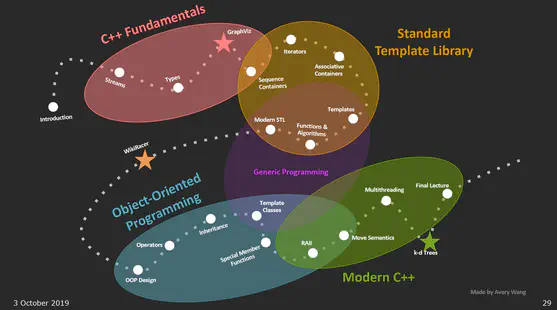# CS 106L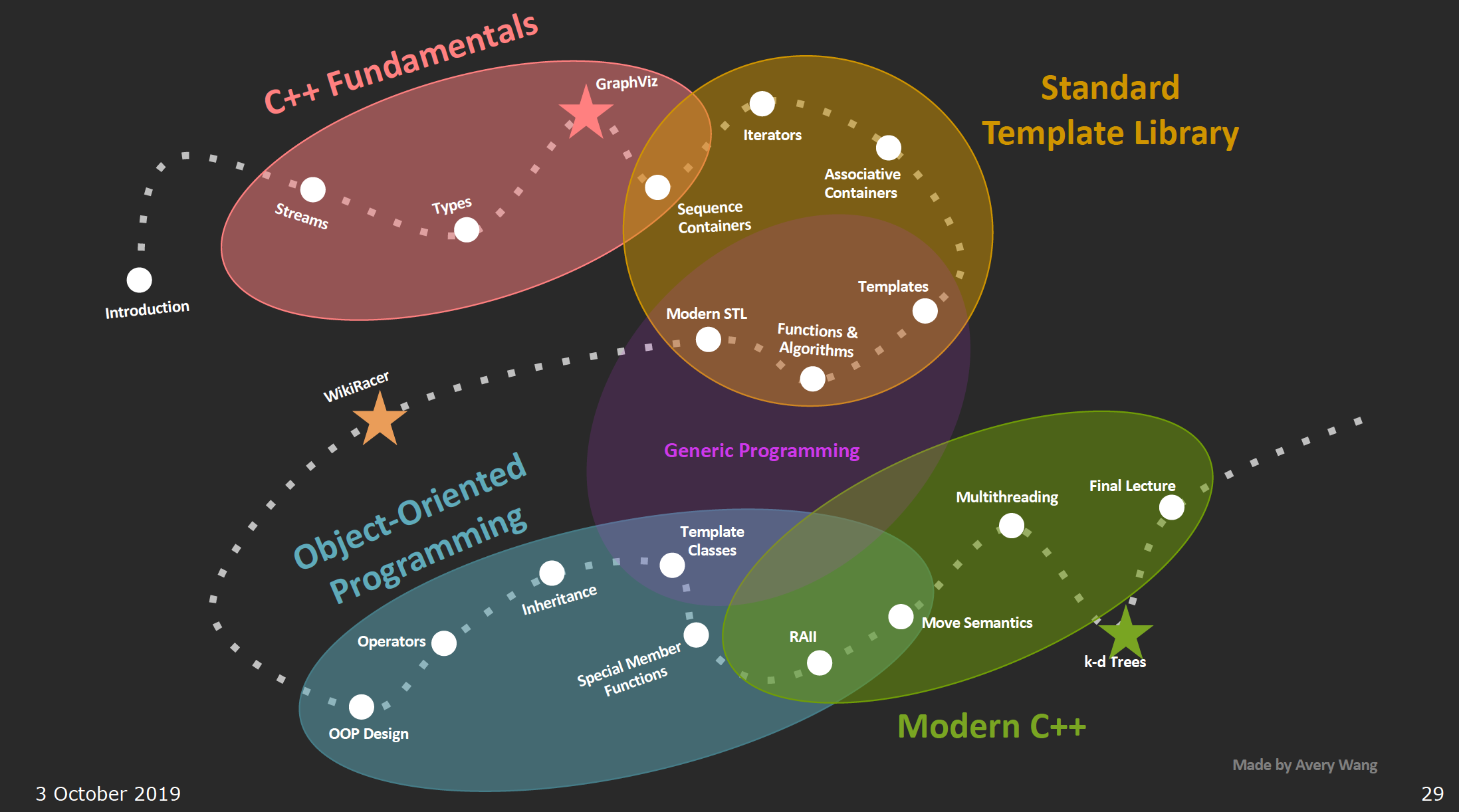## stream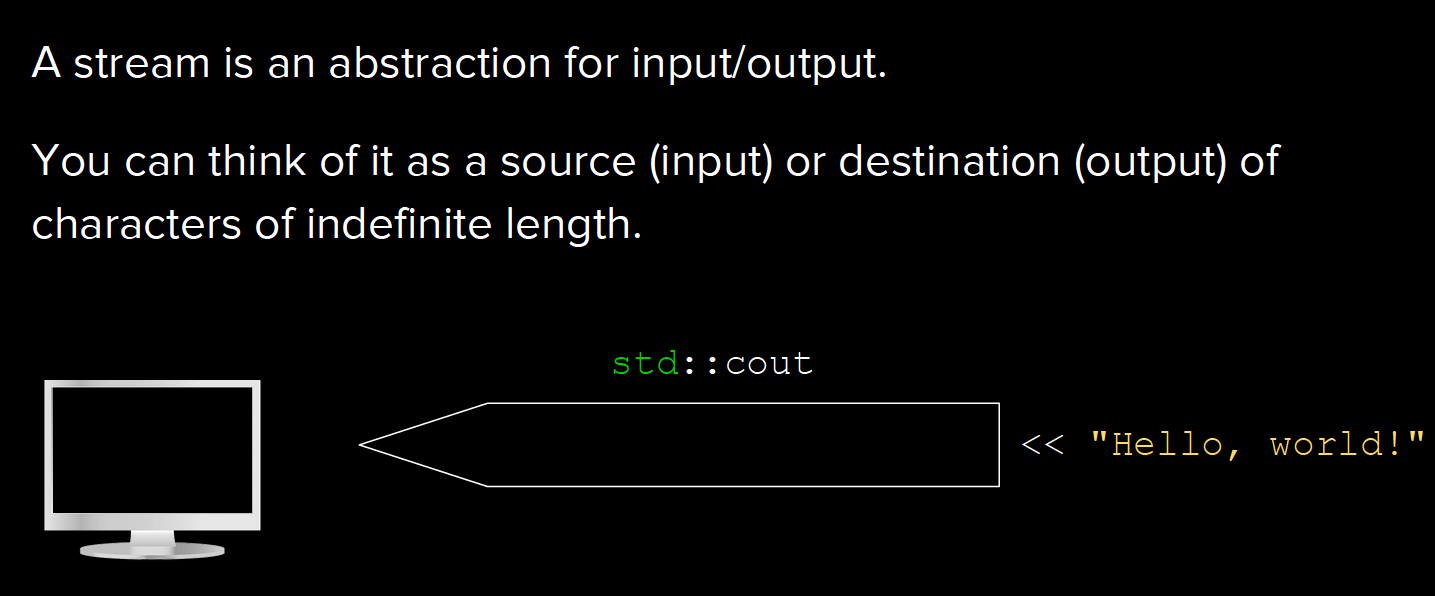### stringstream

Stream class to operate on strings.

Objects of this class use a string buffer that contains a sequence of characters. This sequence of characters can be accessed directly as a string object, using member str.

Characters can be inserted and/or extracted from the stream using any operation allowed on both input and output streams.

stream buffer: “A stream buffer is an object in charge of performing the reading and writing operations of the stream object it is associated with: the stream delegates all such operations to its associated stream buffer object, which is an intermediary between the stream and its controlled input and output sequences.”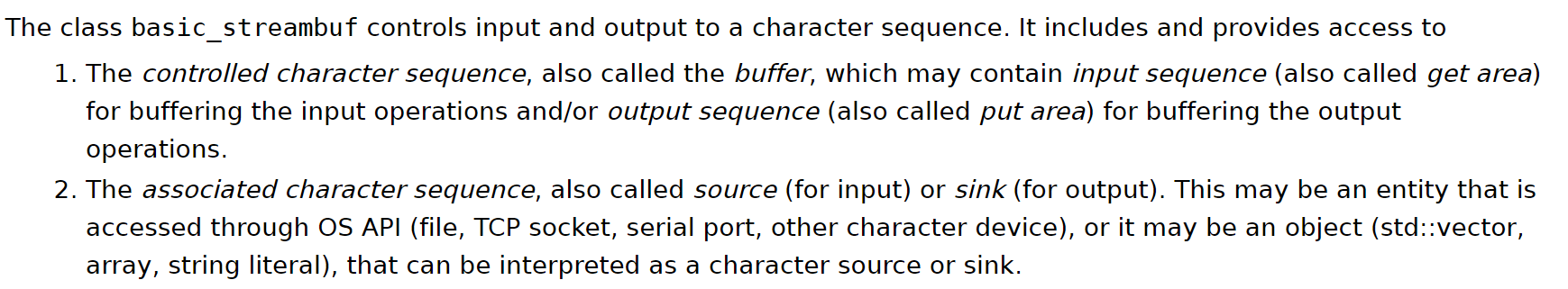All stream objects, no matter whether buffered or unbuffered, have an associated stream buffer: Some stream buffer types may then be set to either use an intermediate buffer or not.

stringstream类继承自几个ios基础类：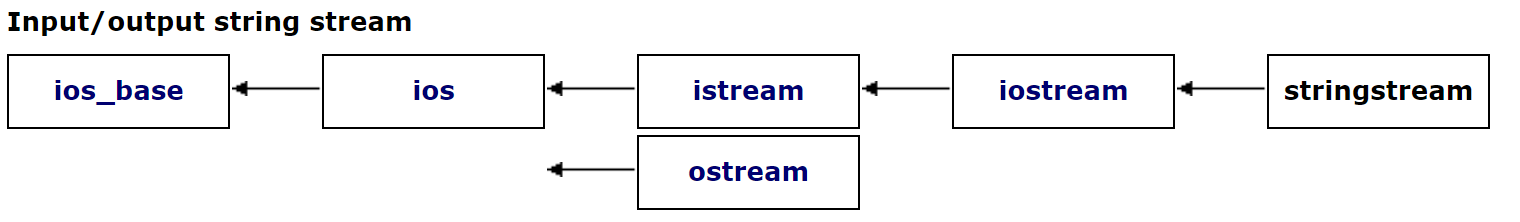stringbuf继承自streambuf: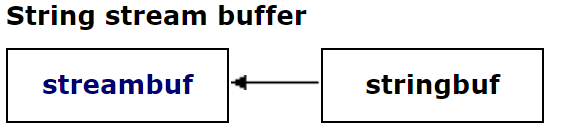Internally, its iostream base constructor is passed a pointer to a stringbuf object constructed with str and which as arguments.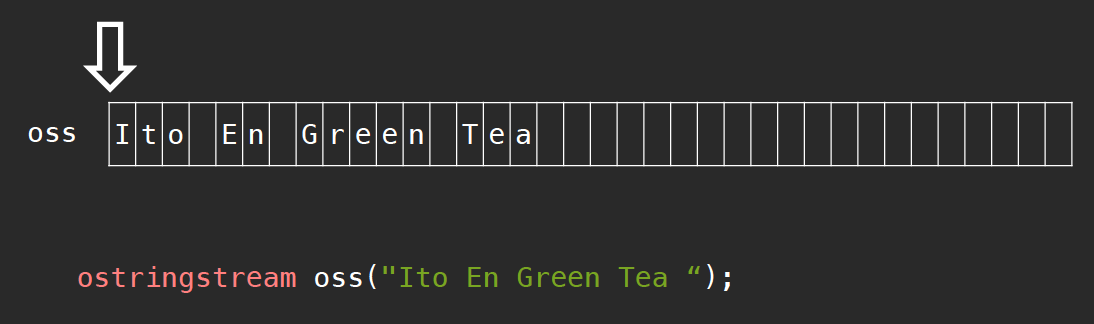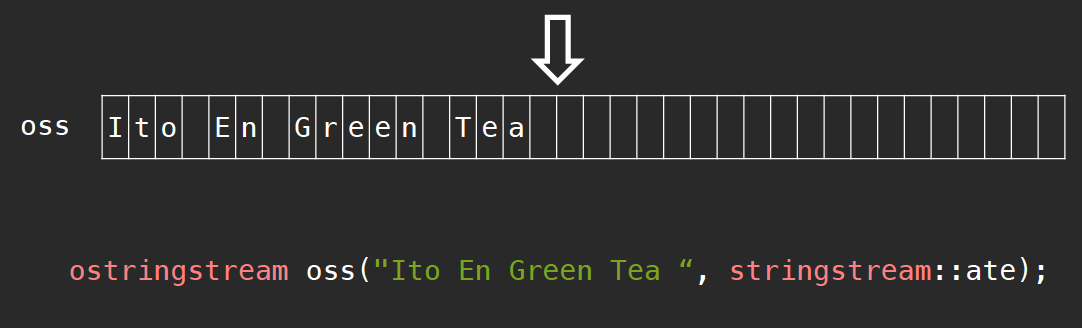• arithmetic types
• stream buffers
• manipulators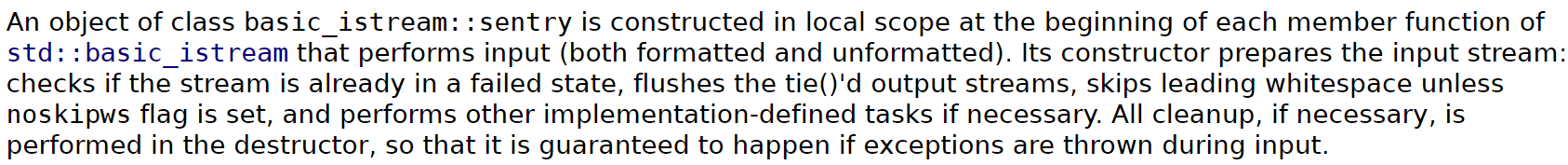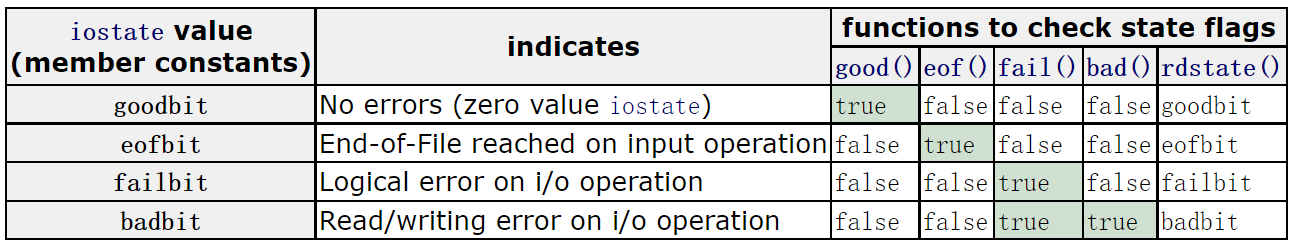#define EOF (-1)


“Reaching the End-of-File sets the eofbit. But note that operations that reach the End-of-File may also set the failbit if this makes them fail (thus setting both eofbit and failbit).”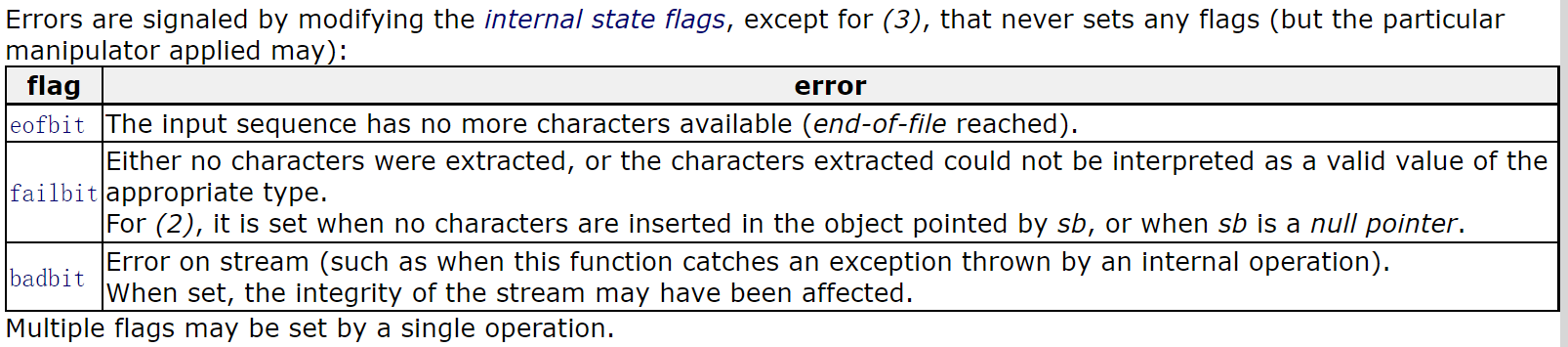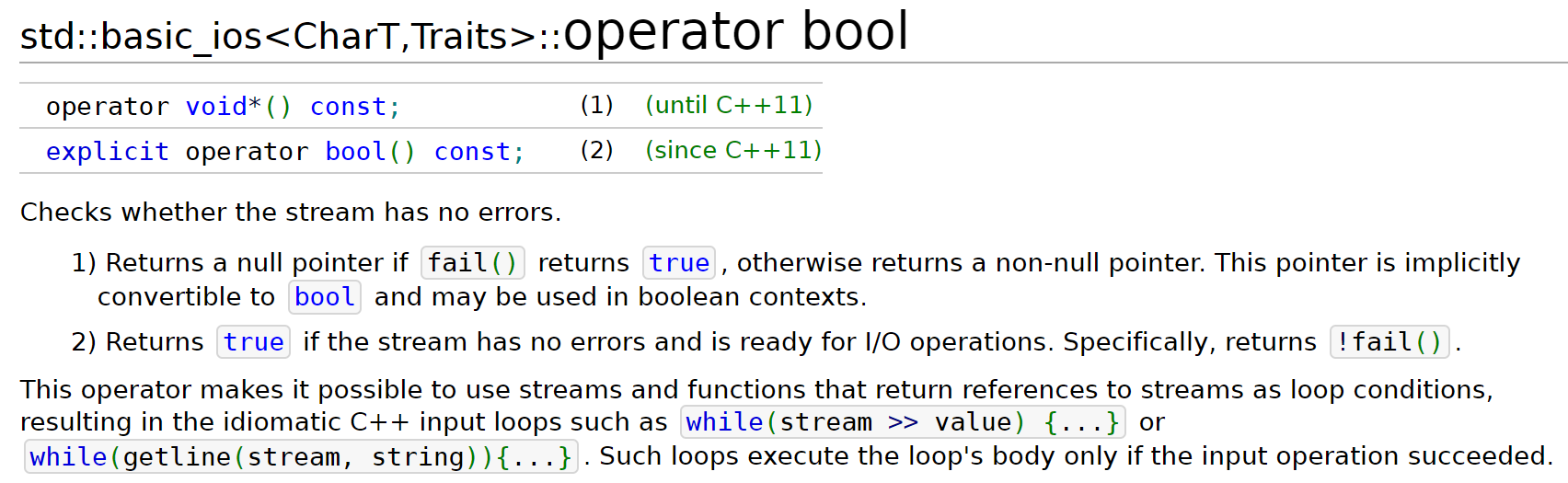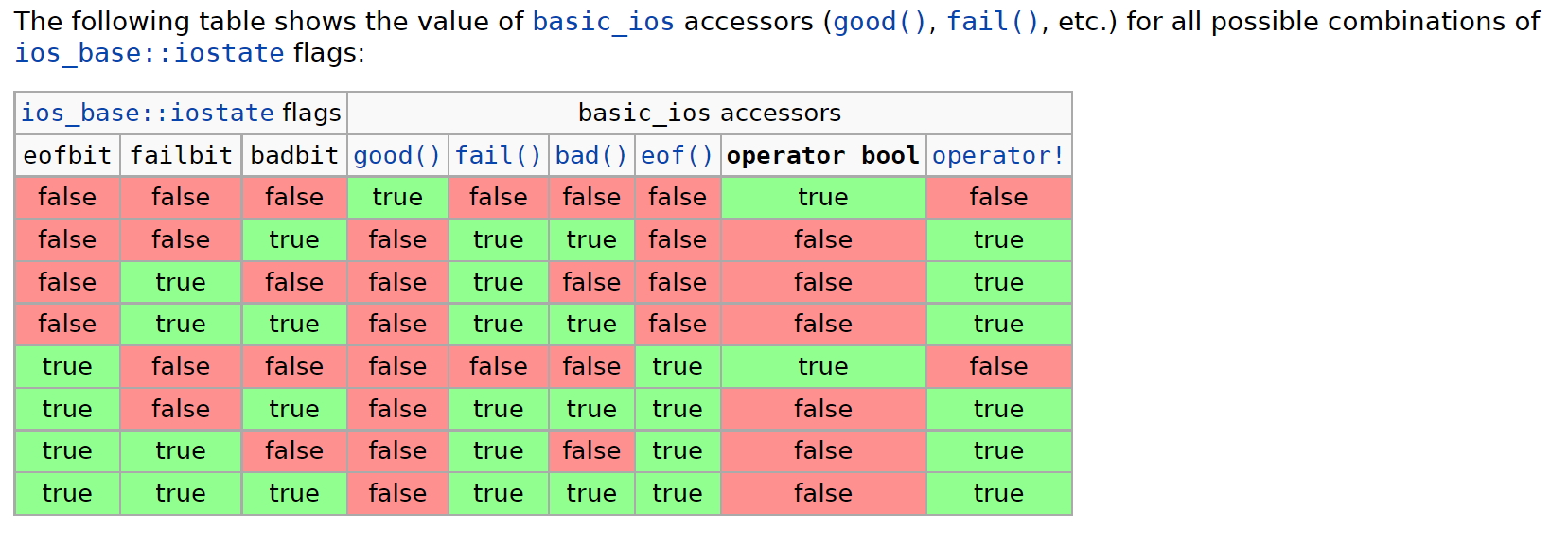int stringToInteger(const string& str) {
istringstream iss(str);
int result;
iss >> result;
cout << "result: " << result << endl;
if (iss.fail())
throw domain_error("error1");
char remain;
iss >> remain;
cout << "remain: " << remain << endl;
if (!iss.fail())
throw domain_error("error2");
return result;
}

// case 1:
// result: 5, remain: l
// error2
stringToInetger("5lol");
// case 2:
// result: 0
// error1
stringToInteger("lol");


“Extracts and parses characters sequentially from the stream to interpret them as the representation of a value of the proper type, which is stored as the value of val. Internally, the function accesses the input sequence by first constructing a sentry object (with noskipws set to false). Then (if good), it calls num_get::get (using the stream’s selected locale) to perform both the extraction and the parsing operations, adjusting the stream’s internal state flags accordingly. Finally, it destroys the sentry object before returning.”

• “The function stops reading characters from the sequence as soon as one character cannot be part of a valid numerical expression (or end is reached). The next character in the sequence is pointed by the iterator returned by the function.”

• Return value: The next character in the sequence right after where the extraction operation ended.”

•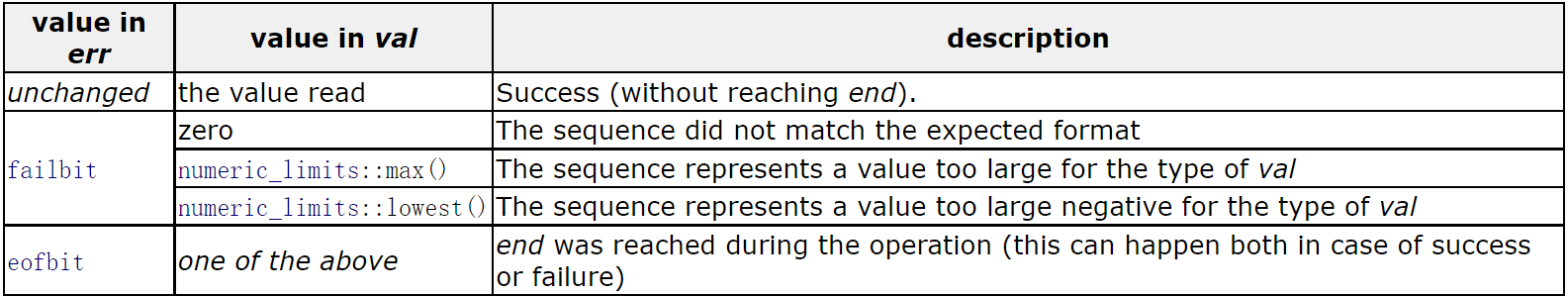#### white space separating？

istream& operator>> (istream& is, string& str);


Notice that the istream extraction operations use whitespaces as separators; Therefore, this operation will only extract what can be considered a word from the stream. To extract entire lines of text, see the string overload of global function getline.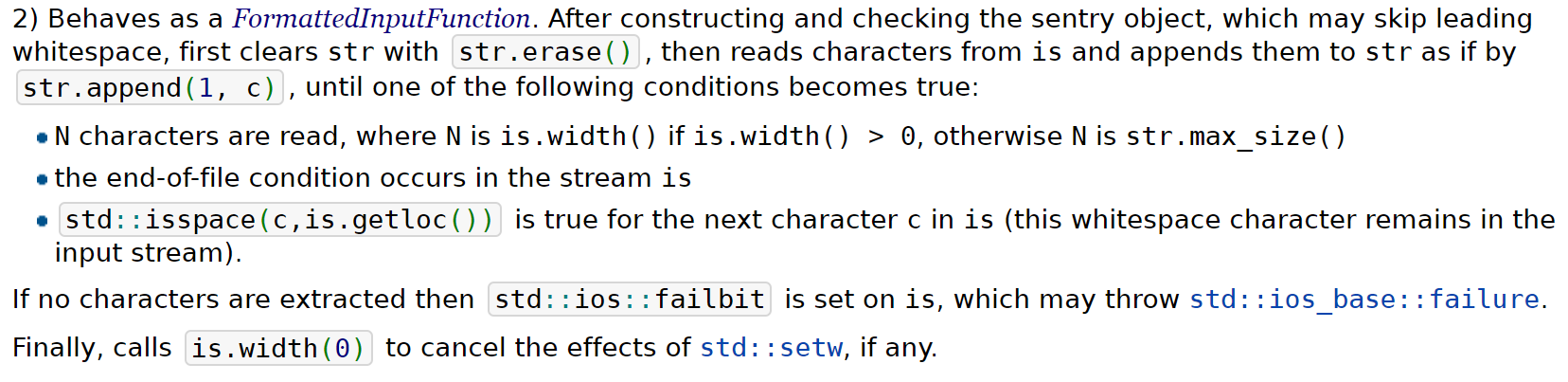1. string a = " 12 3";
stringstream iss(a);
char b, c;
iss >> std::noskipws >> b >> c;
cout << "b: " << b << " c: " << c << endl;
// result: b:  c: 1

string a = " 12 3";
stringstream iss(a);
char b, c;
iss >> b >> c;
cout << "b: " << b << " c: " << c << endl;
// result: b:1  c: 2

2. string a = " 12 3";
stringstream iss(a);
string b, c;
iss >> std::noskipws >> b >> c;
cout << "b: " << b << " c: " << c << endl;
// result: b:  c:

string a = " 12 3";
stringstream iss(a);
string b, c;
iss >> b >> c;
cout << "b: " << b << " c: " << c << endl;
// result: b: 12 c: 3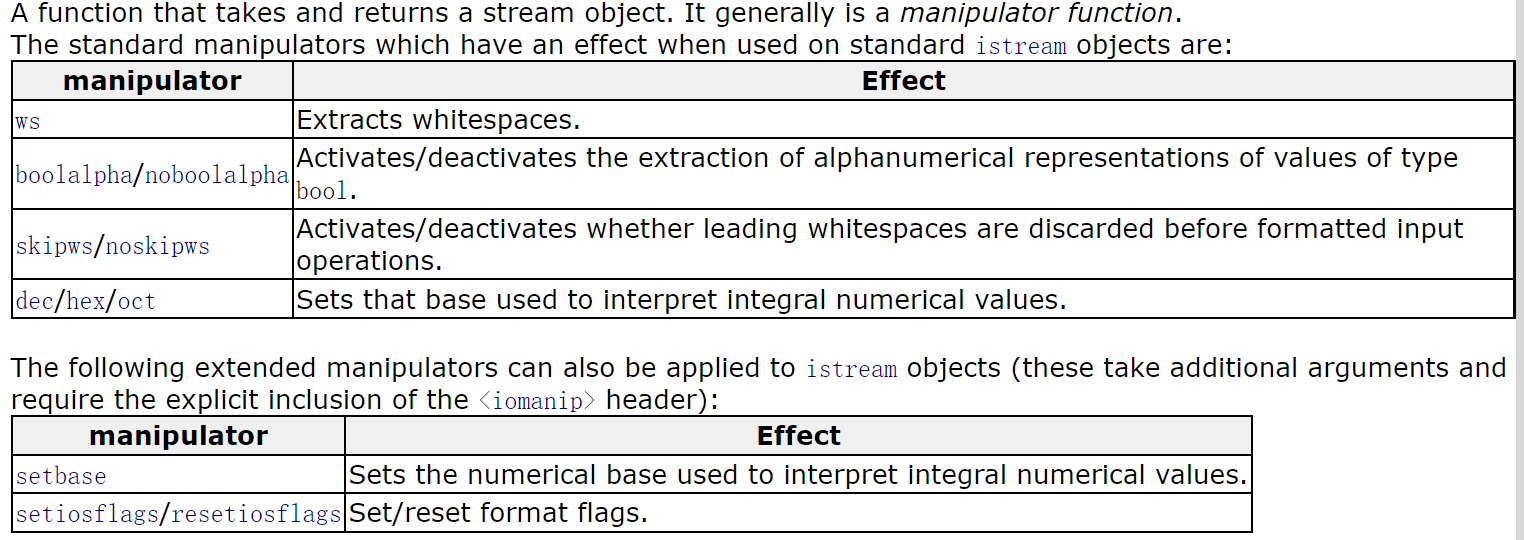“For standard streams, the skipws flag is set on initialization.”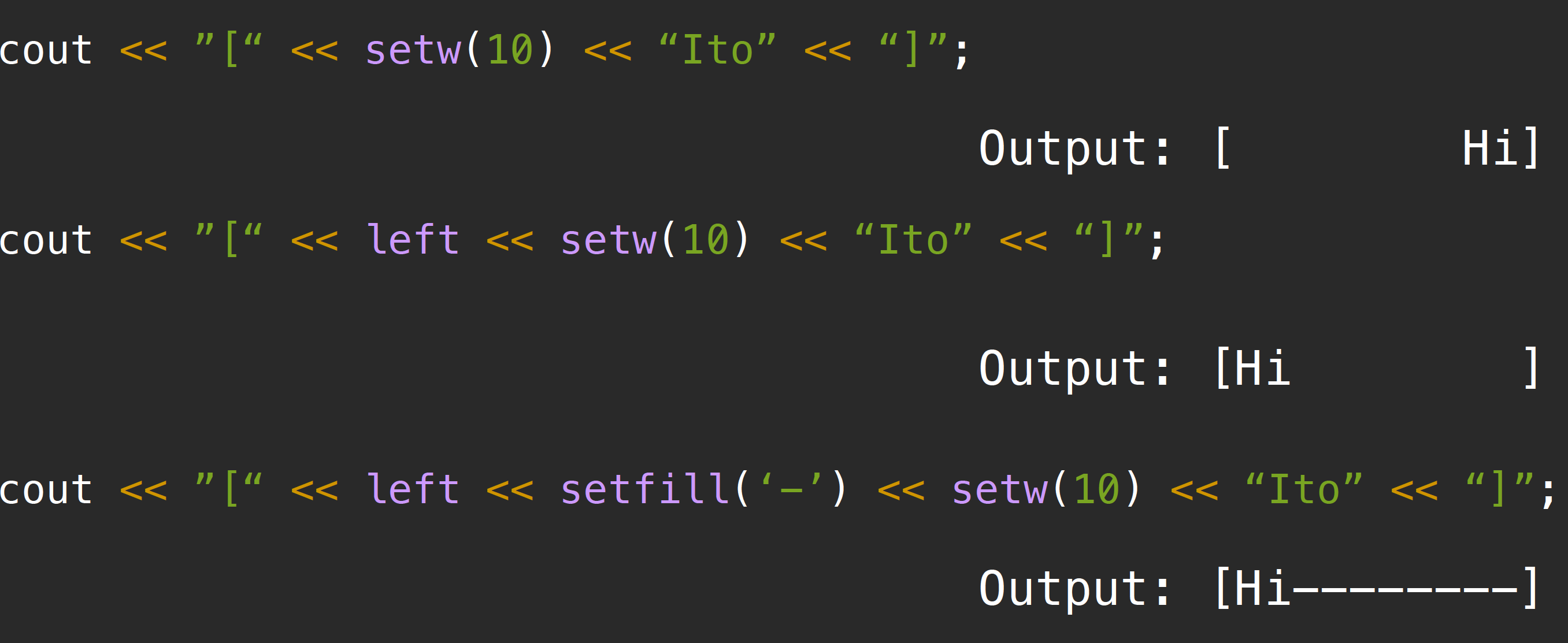#### set position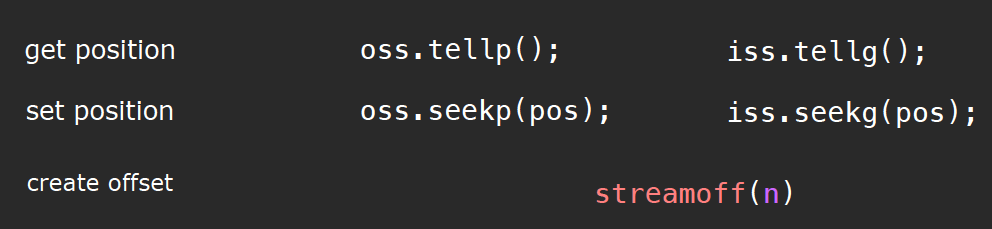fpos = oss.tellp() + streamoff(3);
oss.seekp(pos);
// or
oss.seekp(streamoff(1), stringstream::cur);


### cin & cout

1. 为什么明明输入流默认设置skipws，但是我们输入一个\n时，console内仍然会打印出新的一行？

1. cin is tied to the standard output stream cout (see ios::tie), which indicates that cout’s buffer is flushed (see ostream::flush) before each i/o operation performed on cin.

The tied stream is an output stream object which is flushed before each i/o operation in this stream object.

For stream buffer objects that implement intermediate buffers, this function requests all characters to be written to the controlled sequence.

1. 如果我们注意在输入过程中internal error flag的变化，则在每次flush之后，以及每次cin读取完数据后，内置错误标志位都会被设置成eof. 因为输入流意识到自己读到了字符串的末尾。需要注意的是，如果在输入过程中，标志位在某一刻被设置成fail，那么此后的所有尝试输入的操作均会失效，这一点我们在前边提到过，必须使用clear()清空状态才可以继续进行。

#### ignore

istream& ignore (streamsize n = 1, int delim = EOF);


con.ignore(numeric_limits<streamsize>::max(), ‘\n’);


### getline(string)

If the delimiter is found, it is extracted and discarded (i.e. it is not stored and the next input operation will begin after it).

ifstream file(filename);
while(true) {
string line;
getline(file, line);
if (file.fail()) {
break;
}
}


## type deduction## structure

### pair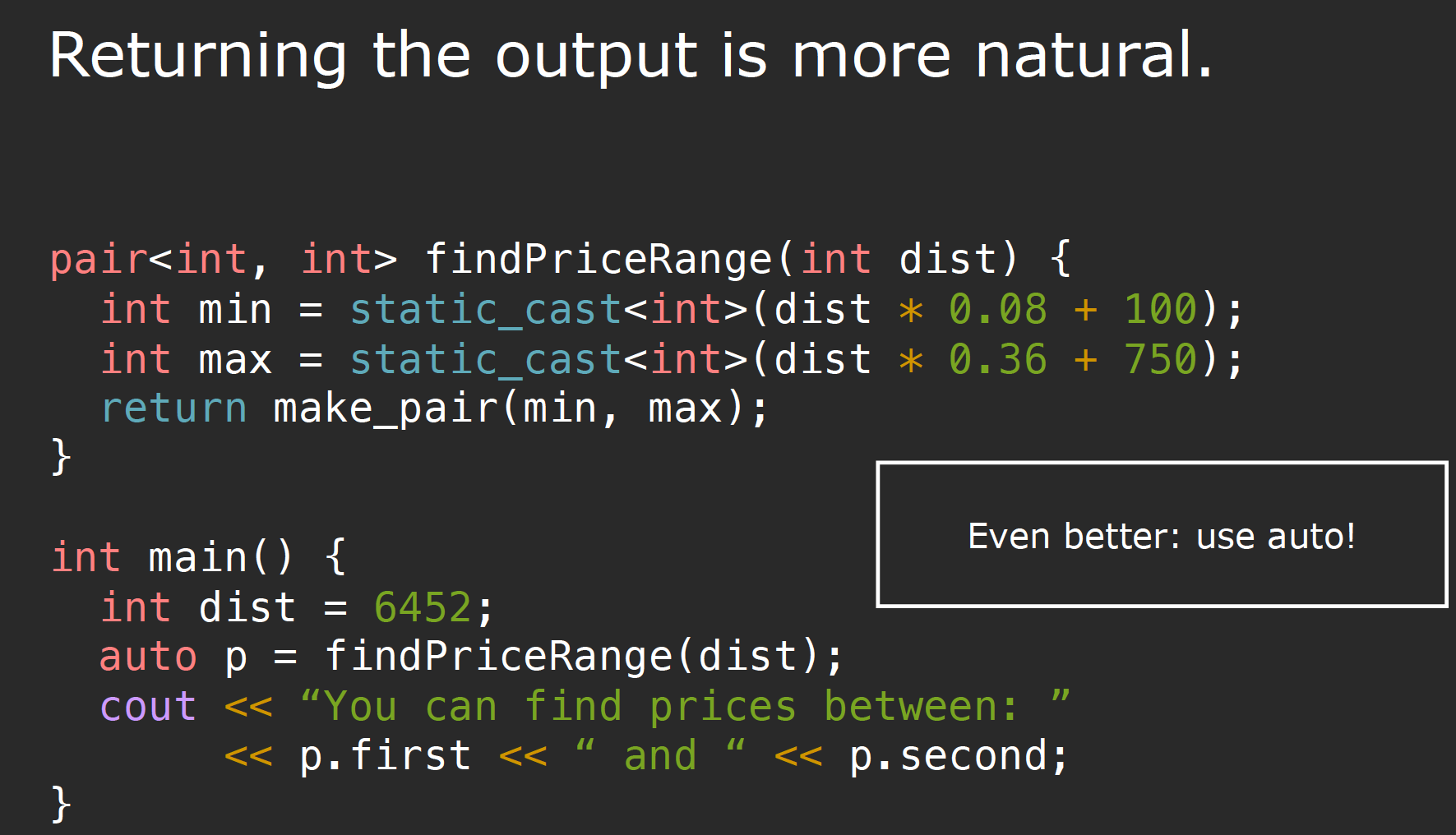std::pair<std::string, std::string> f(std::pair<std::string, std::string> p)
{
return {p.second, p.first}; // list-initialization in return statement
}


### tuple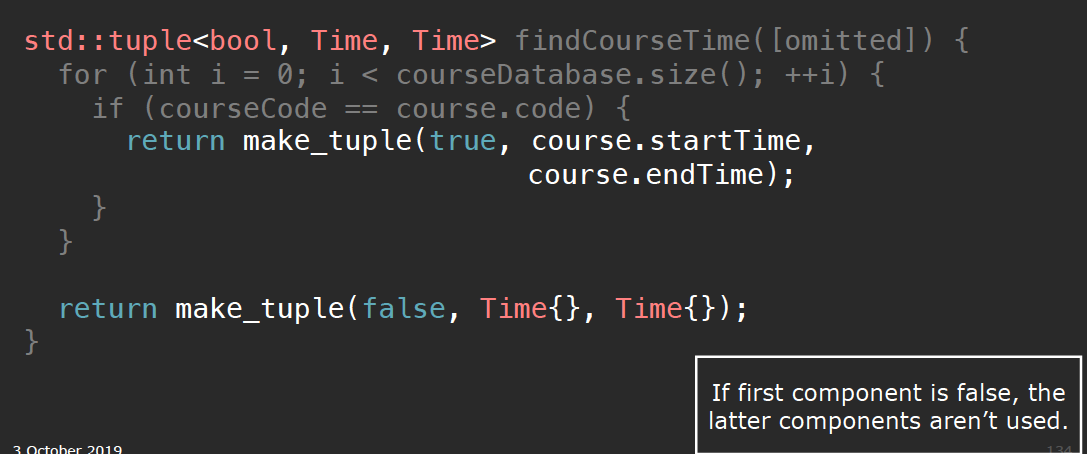### structured binding

auto [min, max] = findPriceRange(dist);


void transformToDST(vector<Course>& courses) {
for (auto& [code, start, end, instructors] : courses) {
start++;
end++;
}
}

void print_map(std::string_view comment, const std::map<std::string, int>& m)
{
std::cout << comment;
for (const auto& [key, value] : m) {
std::cout << key << " = " << value << "; ";
}
std::cout << "\n";
}


### Aggregate initialization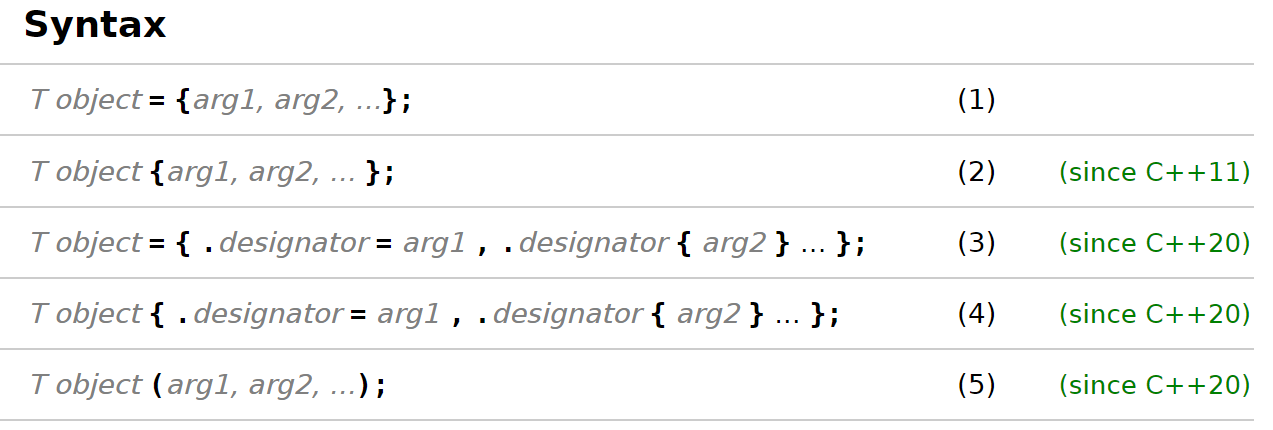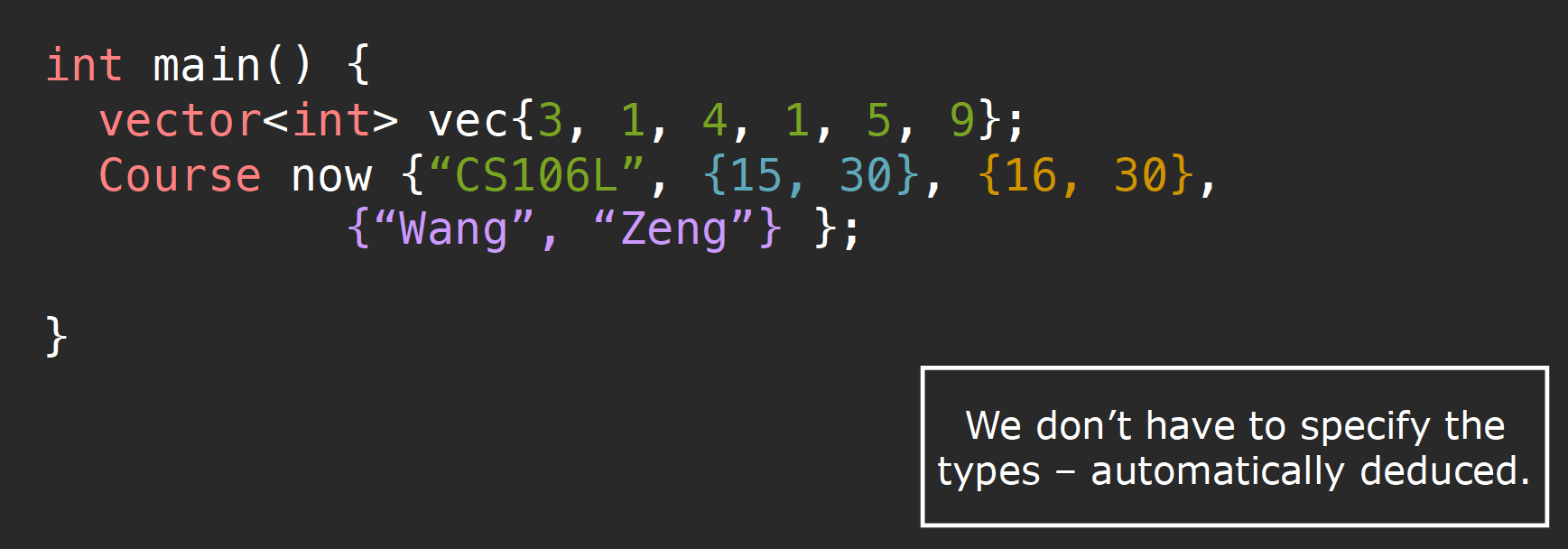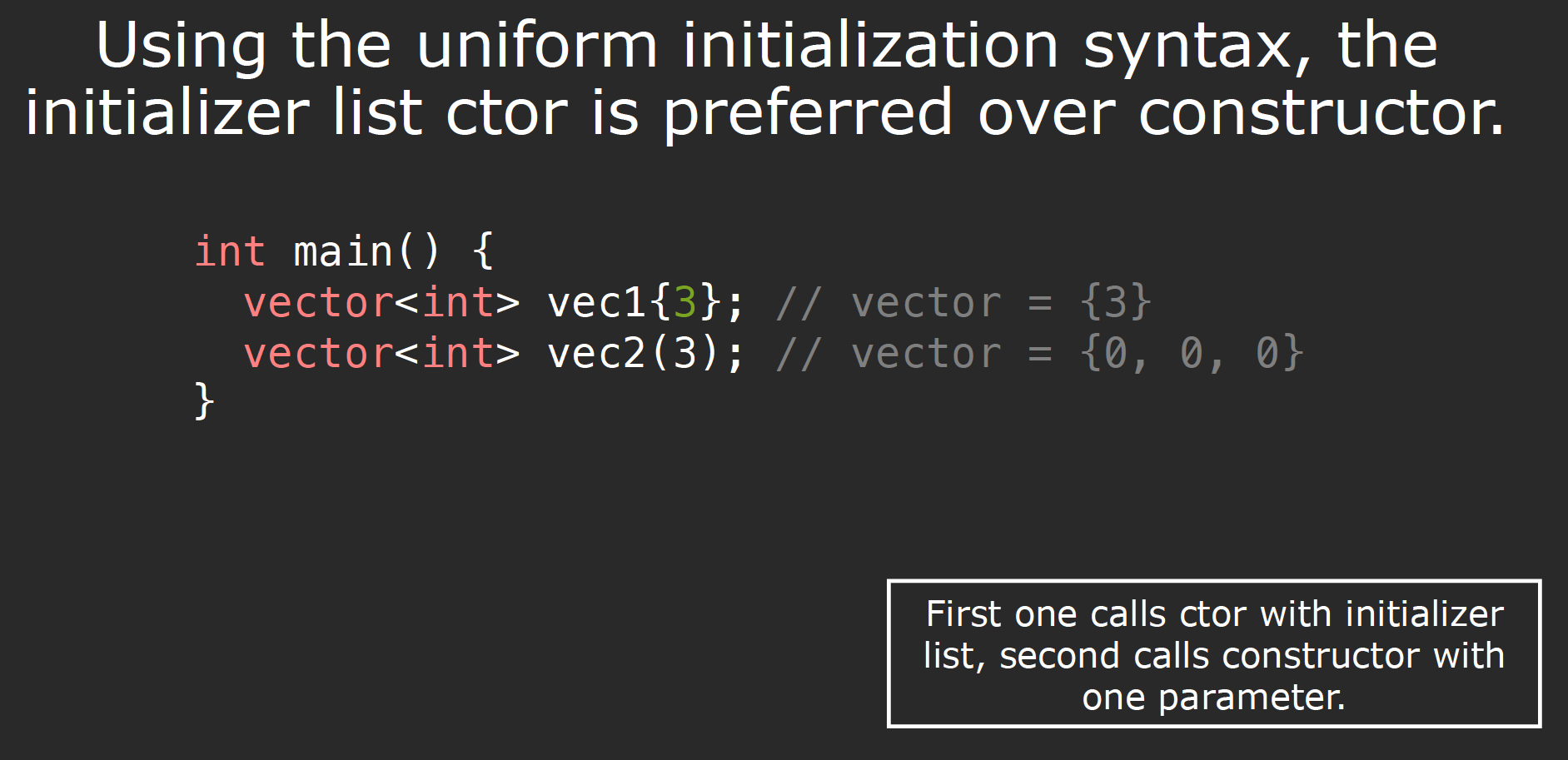foo({ {}, {} }); // OK: call default constructor on both parts
foo({ key, {} }); // OK: call defualt constructor on the second part
foo({ key1, key2 }); // OK


struct A {
string str;
int n = 42;
int m = -1;
};
A{.m=21}  // Initializes str with {}, which calls the default constructor
// then initializes n with = 42
// then initializes m with = 21


struct A { int x; int y; int z; };
A a{.y = 2, .x = 1}; // error; designator order does not match declaration order
A b{.x = 1, .z = 2}; // ok, b.y initialized to 0


union u { int a; const char* b; };
u f = { .b = "asdf" };         // OK, active member of the union is b
u g = { .a = 1, .b = "asdf" }; // Error, only one initializer may be provided


## STL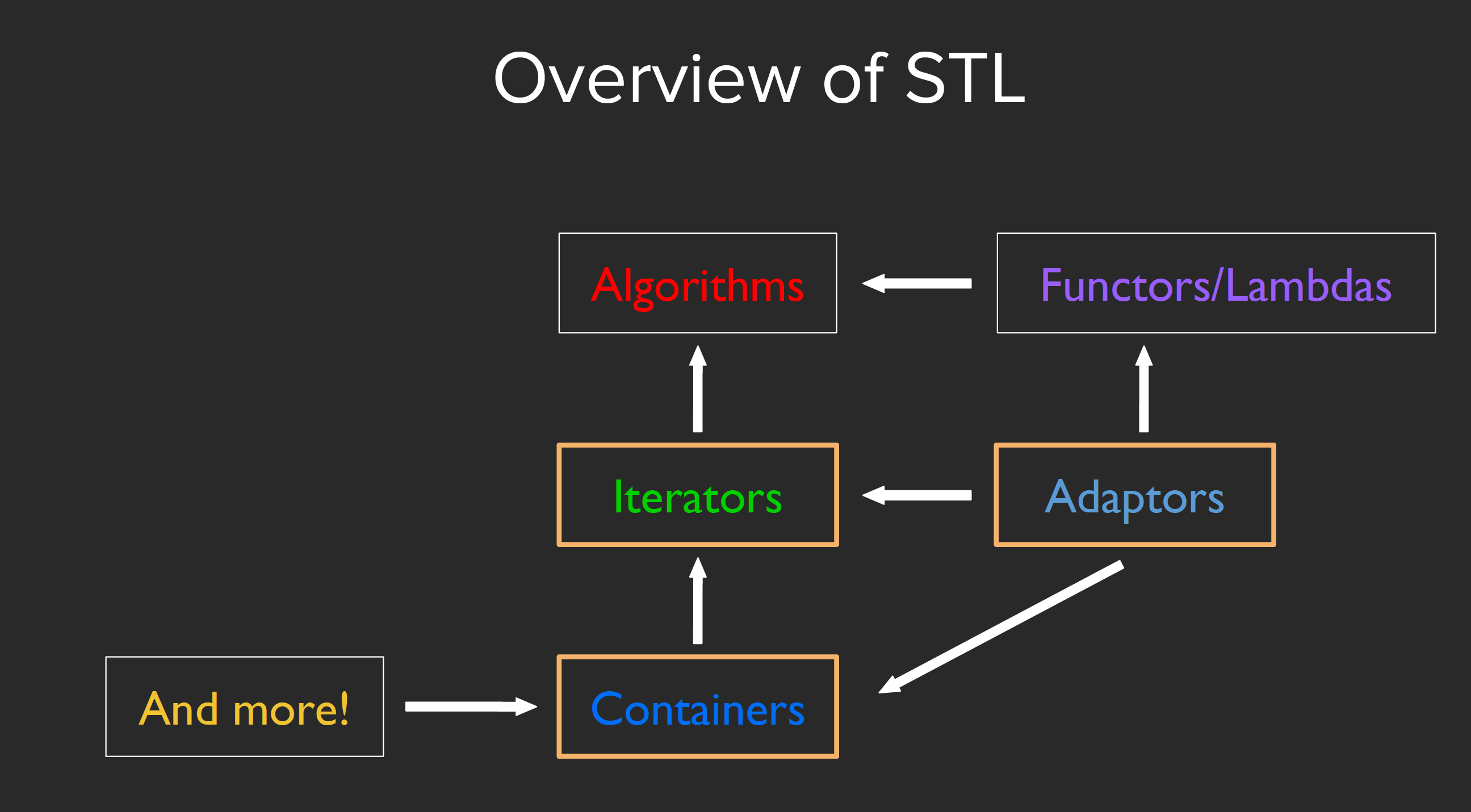vector[i] causes undefined behavior!

• Sequence containers
1. vector
2. deque（双端队列）
3. array
4. list（双向链表）
5. forward_list（单链表）
• Container Adaptors
1. stack
2. queue
3. priority_queue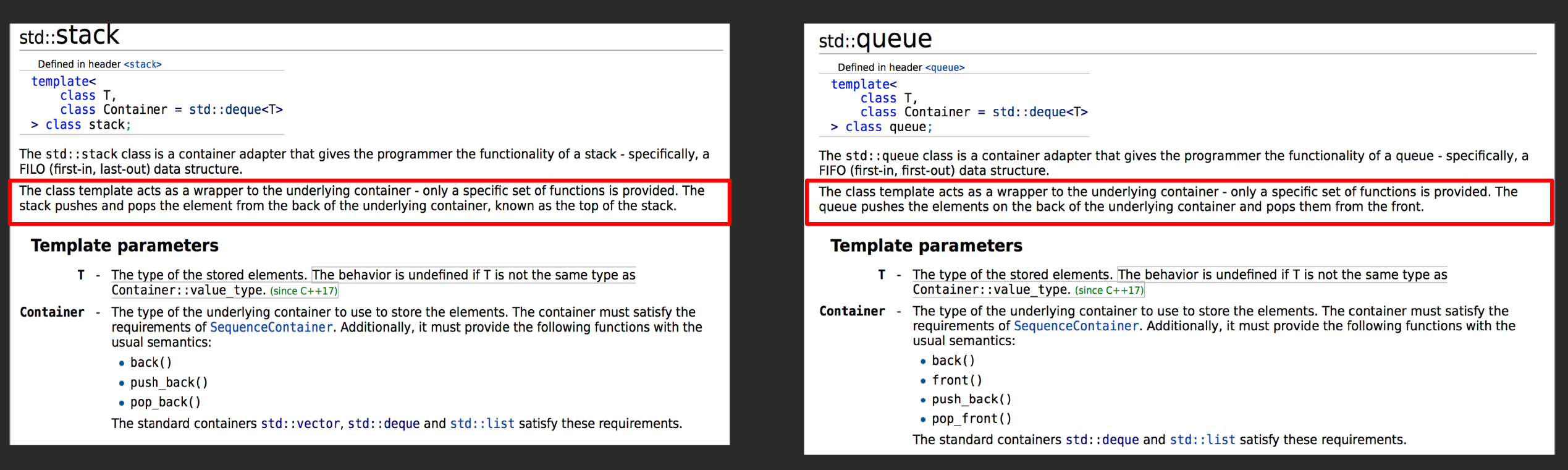• Associative containers
1. map
2. set
3. unordered_map
4. unordered_set
5. multimap/unordered_multimap
6. multiset/unordered_multiset

### deque & vector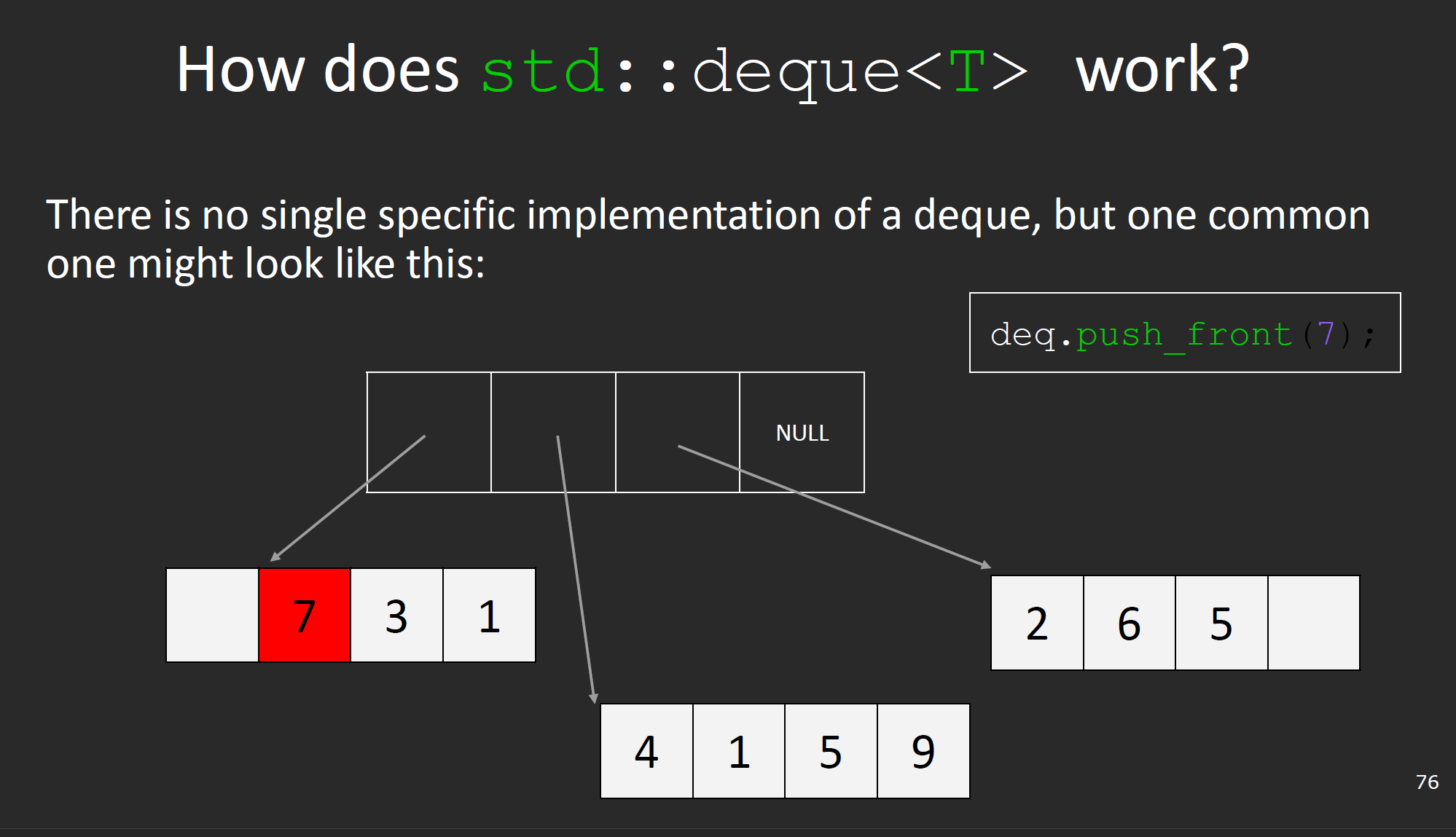### iterator

#### map iterator

map的迭代器比较特殊，它指向一个pair对象，所以我们可以使用a.firsta.second获取键与值：

map<int, int> m;
map<int, int>::iterator i = m.begin();
map<int, int>::iterator end = m.end();
while(i != end) {
cout << (*i).first << (*i).second << endl;
++i;
}


#### lower_bound & upper_bound

lower_bound接受一个值v，返回一个iterator对象，该对象指向第一个不小于元素v的位置，如果没有找到，则指向end

upper_boundlower_bound的工作方式类似，但是其返回的迭代器对象指向第一个大于元素v的位置。

#### Iterator Types Introduction1. Input Iterators

• 连续，表示所应用的数据结构不可以是queuestack等（不包括deque，因为deque实际效果上是一个优化了前序插入的vector
• 单向single-pass），迭代器对应的每个位置只能允许被经过一次，这个限制条件之后会详细解释

input iterators只能作为右值（rvalue）被解引用：

int val = *itr;


1. output iterators

*itr = 12;


1. forward iterators

1. bidirectional iterators

vector<int>::iterator itr = v.begin();
--itr;


1. random Access iterators

Instead of being defined by specific types, each category of iterator is defined by the operations that can be performed on it. This definition means that any type that supports the necessary operations can be used as an iterator – for example, a pointer supports all of the operations required by LegacyRandomAccessIterator, so a pointer can be used anywhere a LegacyRandomAccessIterator is expected.

C++20中，根据新的关键字concept以及requires对迭代器类型根据新的系统设计了一套定义，但是基本的类型是相似的；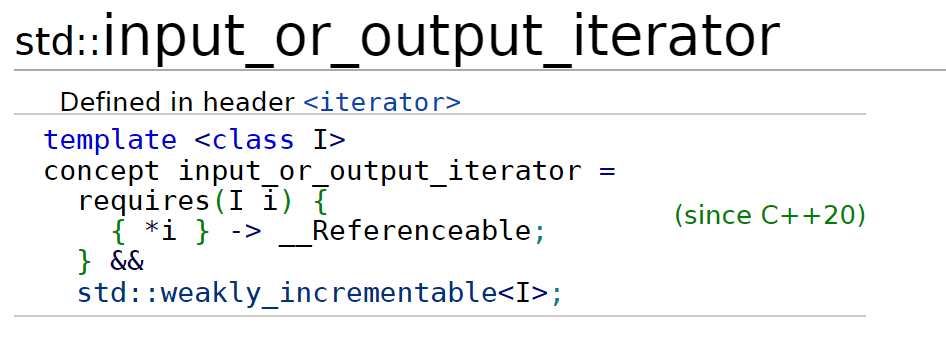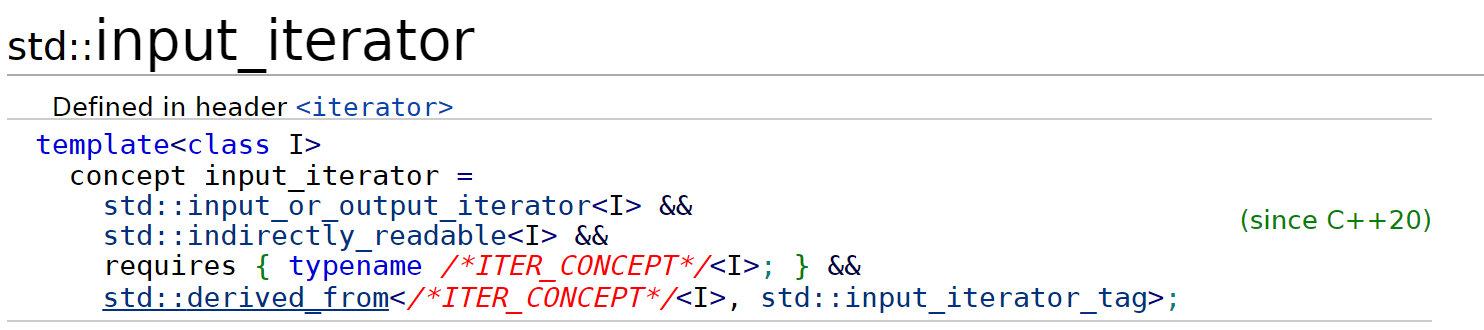#### What is single-pass?

For input iterators, a == b does not imply ++a == ++b. (Equality does not guarantee the substitution property or referential transparency.) Algorithms on input iterators should never attempt to pass through the same iterator twice. They should be single pass algorithms. Value type T is not required to be an Assignable type (23.1). These algorithms can be used with istreams as the source of the input data through the istream_iterator class.

std::istream_iterator is a single-pass input iterator that reads successive objects of type T from the std::basic_istream object for which it was constructed, by calling the appropriate operator>>. The actual read operation is performed when the iterator is incremented, not when it is dereferenced. The first object is read when the iterator is constructed. Dereferencing only returns a copy of the most recently read object.

The default-constructed std::istream_iterator is known as the end-of-stream iterator. When a valid std::istream_iterator reaches the end of the underlying stream, it becomes equal to the end-of-stream iterator. Dereferencing or incrementing it further invokes undefined behavior.

##### Increment/decrement operators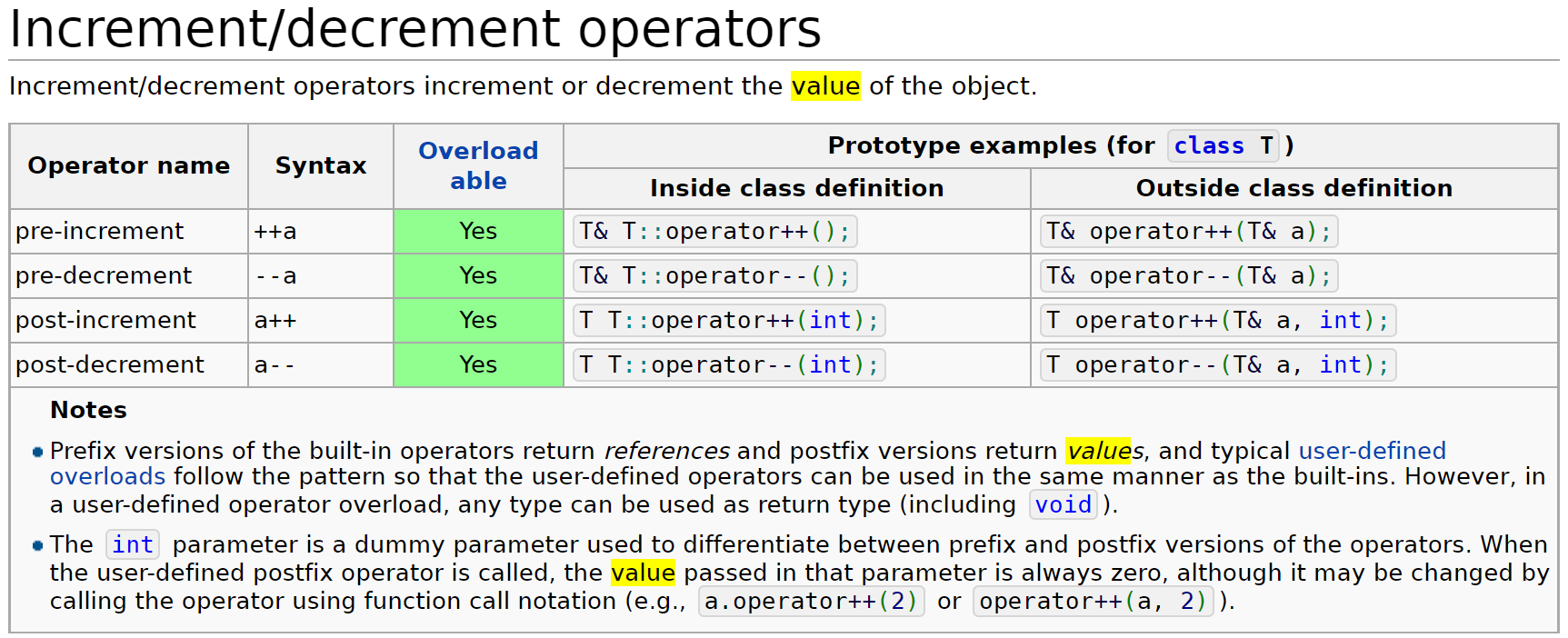#### Write our own iterator?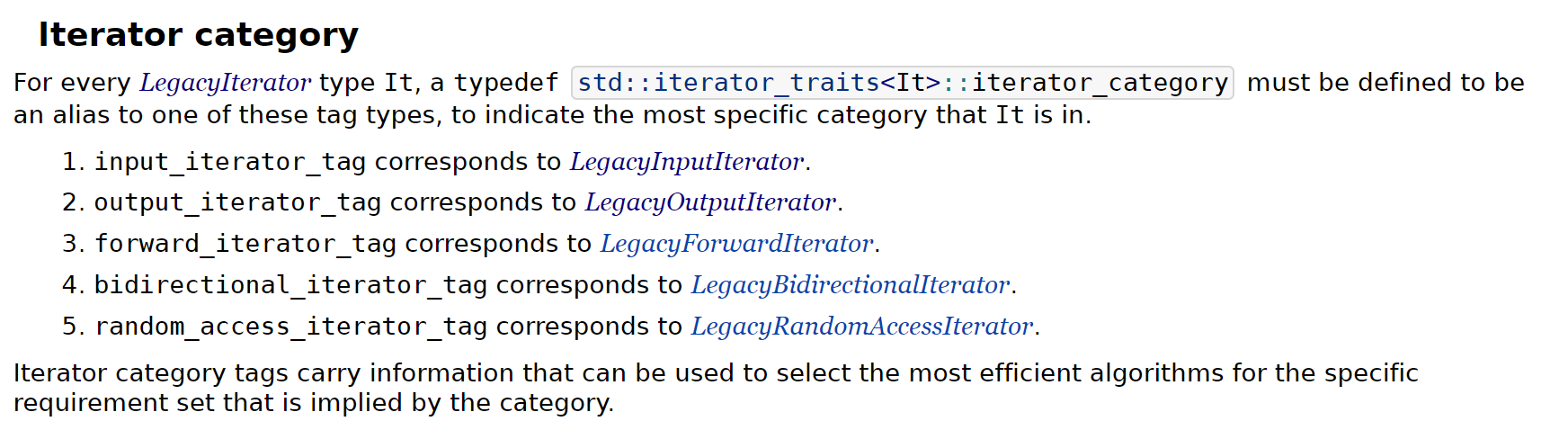##### iterator – friend class

friend iterator_of_hashmap<HashMap, !Const>;

##### iterator – const?

using node_pointer = std::conditional_t<Const, const node*, node*>;

##### iterator – basic requirement
using difference_type = std::ptrdiff_t;
using value_type = std::conditional_t<Const, const _value_type, _value_type>;
using pointer = std::conditional_t<Const, const _value_type*, _value_type*>;
using reference = std::conditional_t<Const, const _value_type&, _value_type&>;
using iterator_category = std::forward_iterator_tag;


/** a conversion operator */
operator iterator_of_hashmap<HashMap, true>() const {
return iterator_of_hashmap<HashMap, true>{_buckets_array, _ptr, curr_bucket};
}


## Template–Generic Programming

### function template

template<typename T>
T getInteger(const string& prompt, const string& reprompt) {
while (true) {
cout << prompt;
string line;
T result;
char extra;
if (!getline(cin, line))
throw domain_error(“[shortened]”);
istringstream iss(line);
if (iss >> result && !(iss >> extra))
return result;
cout << reprompt << endl;
}
}


### class template

e.g.

template<class T, class Container = std::vector<T>>
class Priority_Q {
// do something
};


### template parameters & template arguments

1. non-type template parameter

• An integral type
• An enumeration type
• A pointer or reference to a class object
• A pointer or reference to a function
• A pointer or reference to a class member function
• std::nullptr_t
• A floating point type (since C++20)

1. The top-level cv-qualifiers on the template-parameter are ignored when determining its type
// this const will be ignored
template<const int k>
void foo() {
// do something
}

1. When the name of a non-type template parameter is used in an expression within the body of the class template, it is an unmodifiable prvalue unless its type was an lvalue reference type, or unless its type is a class type (since C++20).
template <int N>
void f()
{
// N is a prvalue, so this is invalid! It will produce a compile error
N = 42;
}

1. the expression we give to the template as its argument must can be parsed and substitued during compile time (non-constant expressions cannot do this, since they could change during runtime)

template <int N>
void f()
{
// do something
}

int main() {
int a = 1;
const int b = 0; // constexpr int b = 0;
// error
f<a>();
// ok
f<b>();
}

1. type template parameter

template<typename... Ts> concept C2 = true; // variadic concept
template<C2... T> struct s3;      // constraint-expression is (C2<T> && ...)

1. template template parameter

// two type template parameters and one template template parameter:
template<typename K, typename V, template<typename> typename C = my_array>
class Map
{
C<K> key;
C<V> value;
};


#### implicit inference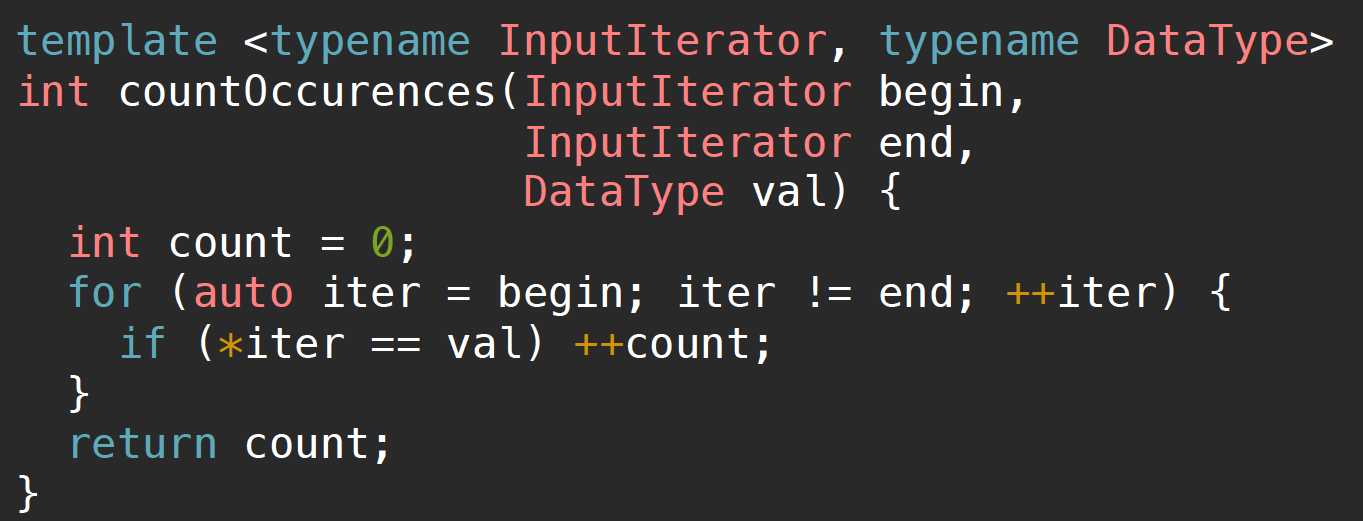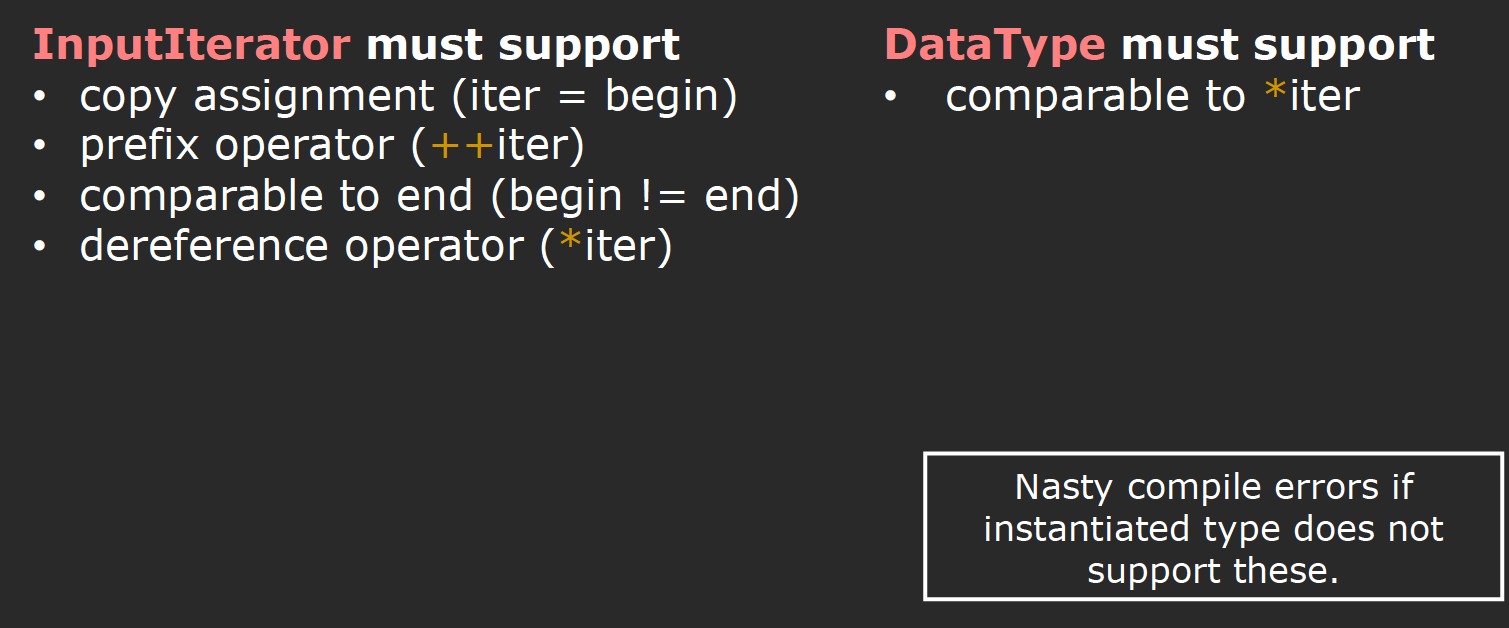C++20中给出了新的关键字conceptsrequires，也就是在之前我们提到过用于重写iterator的两个关键字，他们可以帮助我们在编译阶段就检查出参数错误，将报错的位置从函数主体转移到这两个关键字的位置上：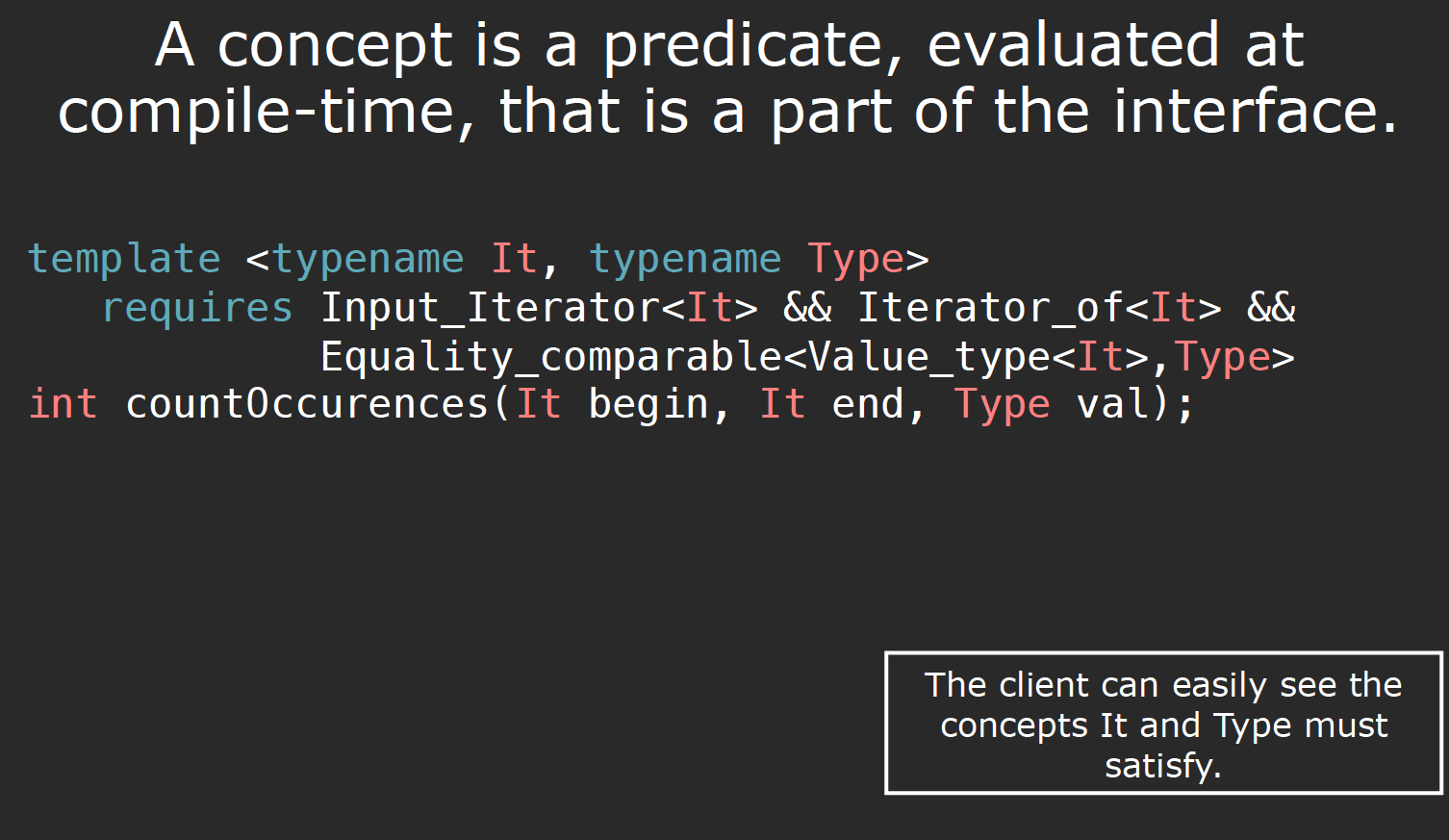### Compile a template function call

struct Bar {
typedef double internalType;
};

template <typename T>
typename T::internalType foo(const T& t) {
cout << "foo<T>\n";
return 0;
}

int main() {
foo(Bar());
foo(0); // << error!
}


#### name lookup

std::cout << std::endl;


##### unqualified name lookup & qualified name lookup

qualified name即在作用域解析符::右侧出现的名称，所以他包括：

• class member
• namespace member
• enumerator

#include <iostream>
int main() {
struct std{};
std::cout << "fail\n"; // Error: unqualified lookup for 'std' finds the struct
::std::cout << "ok\n"; // OK: ::std finds the namespace std
}


#include <iostream>
int main()
{
std::cout << "Test\n"; // There is no operator<< in global namespace, but ADL
// examines std namespace because the left argument is in
// std and finds std::operator<<(std::ostream&, const char*)
operator<<(std::cout, "Test\n"); // same, using function call notation

// however,
std::cout << endl; // Error: 'endl' is not declared in this namespace.
// This is not a function call to endl(), so ADL does not apply

endl(std::cout); // OK: this is a function call: ADL examines std namespace
// because the argument of endl is in std, and finds std::endl

(endl)(std::cout); // Error: 'endl' is not declared in this namespace.
// The sub-expression (endl) is not a function call expression
}


#### Template Argument Deduction

In order to instantiate a function template, every template argument must be known, but not every template argument has to be specified. When possible, the compiler will deduce the missing template arguments from the function arguments. This occurs when a function call is attempted, when an address of a function template is taken, and in some other contexts.

#include <iostream>

int main()
{
std::cout << "Hello, world" << std::endl;
// operator<< is looked up via ADL as std::operator<<,
// then deduced to operator<<<char, std::char_traits<char>> both times
// std::endl is deduced to &std::endl<char, std::char_traits<char>>
}


#### Template Argument Substitution

When all template arguments have been specified, deduced or obtained from default template arguments, every use of a template parameter in the function parameter list is replaced with the corresponding template arguments.

In order to compile a function call, the compiler must first perform name lookup, which, for functions, may involve argument-dependent lookup, and for function templates may be followed by template argument deduction. If these steps produce more than one candidate function, then overload resolution is performed to select the function that will actually be called.

Informally “A is more specialized than B” means “A accepts fewer types than B”.

StackOverflow上有一篇很详细的文章描述这一机理。这也是为什么在先前的例子中加入一个匹配的新function，就可以编译成功的原因。

#### SFINAE

##### Why we need SFINAE?

• 首先，对于我们自己的函数模板代码，如果其中存在if-else分支，并且我们利用了compile-time-if，那么程序很有可能会在编译阶段报错，因为他会编译if-else两个分支，之后程序发现自己无法reject the invalid code (for this case)
template <typename T>
std::string str(T t) {
if (std::is_convertible_v<T, std::string>)
return t;
else if (std::is_same_v<T, bool>)
return t ? "true" : "false";
else
return std::to_string(t);
}


##### How to leverage SFINAE?

###### Tag Dispatching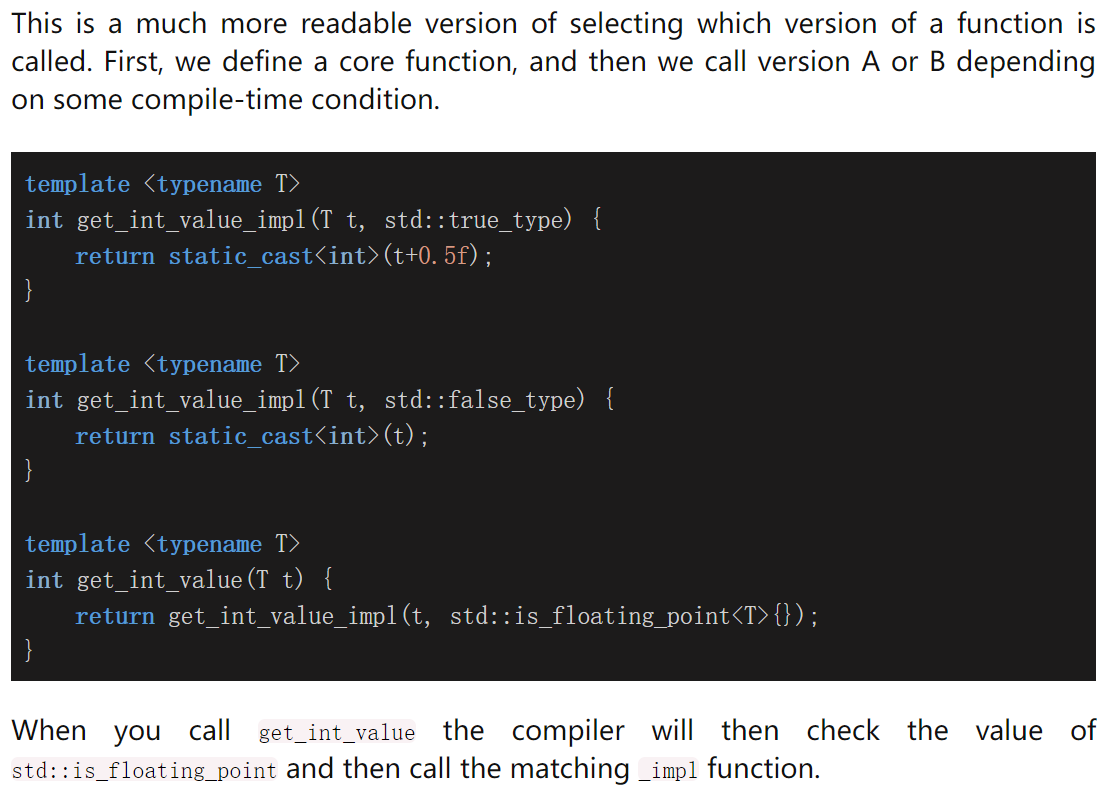###### enable_if

std::enable_if can be used as an additional function argument (not applicable to operator overloads), as a return type (not applicable to constructors and destructors), or as a class template or function template parameter.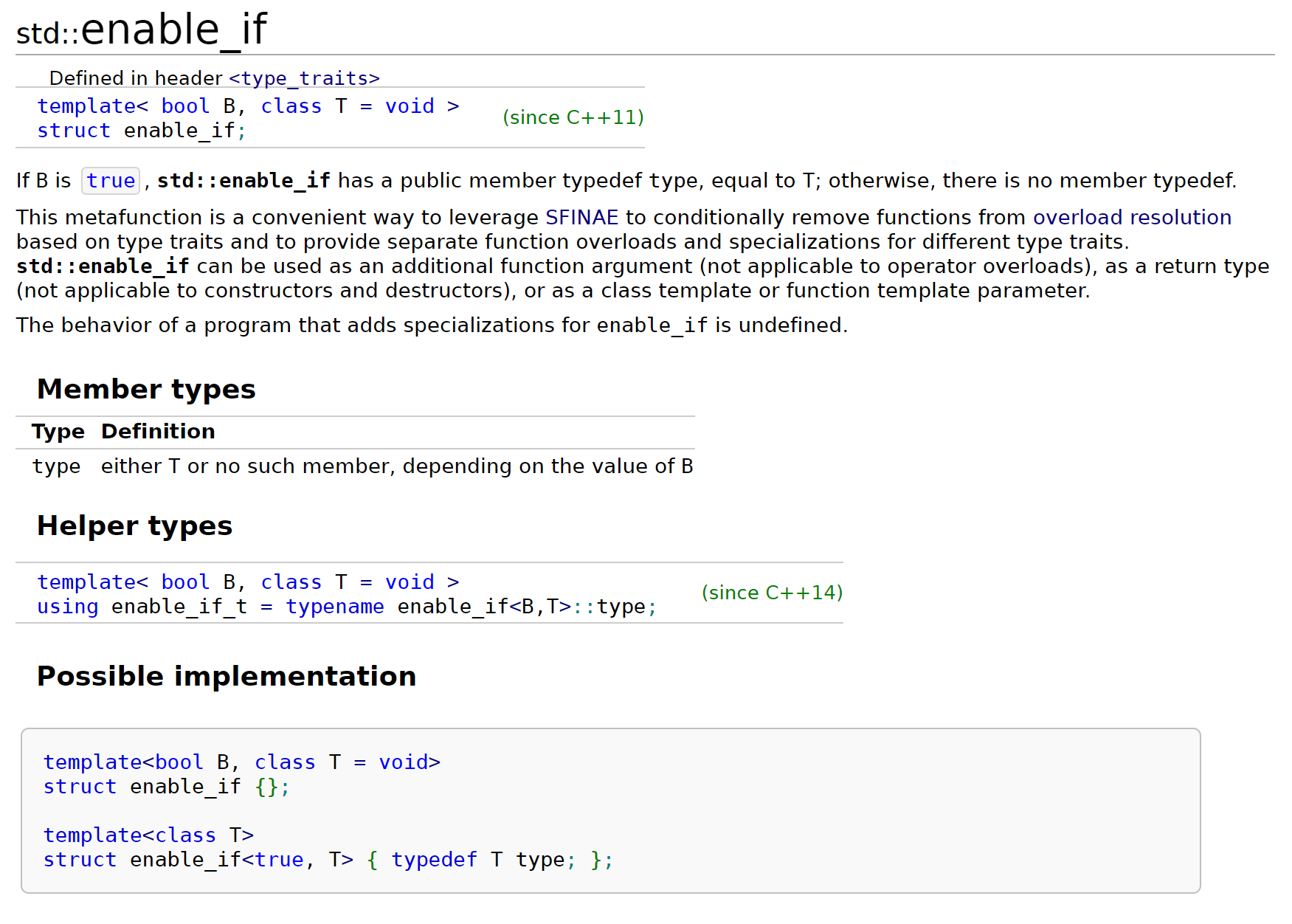template <class T>
typename std::enable_if<std::is_arithmetic<T>::value, T>::type


template <class T>
typename std::enable_if_t<std::is_arithmetic_v<T>, T>


enable_if常与type traits一起使用：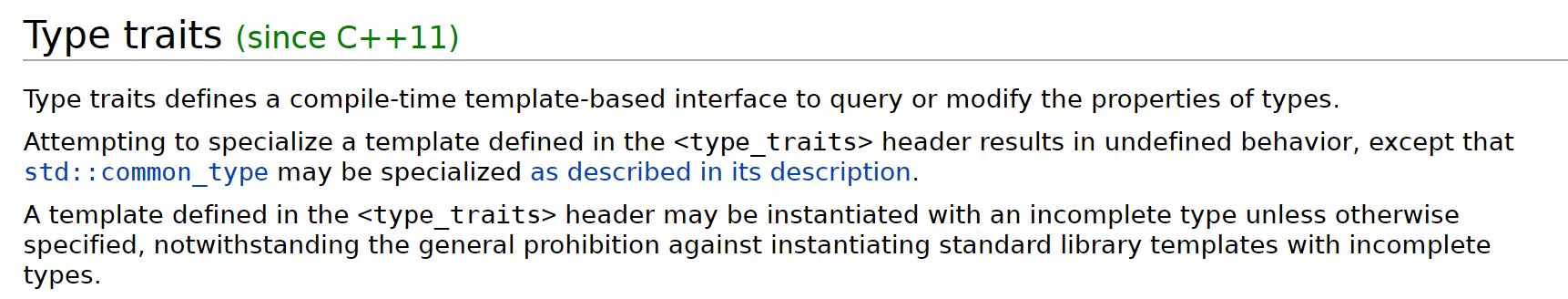type traits编译阶段用以确定模板特征和属性。在C++14中也提出了类似于is_arithmetic_v的形式（原本的valueTarithmetic type是返回true，反之false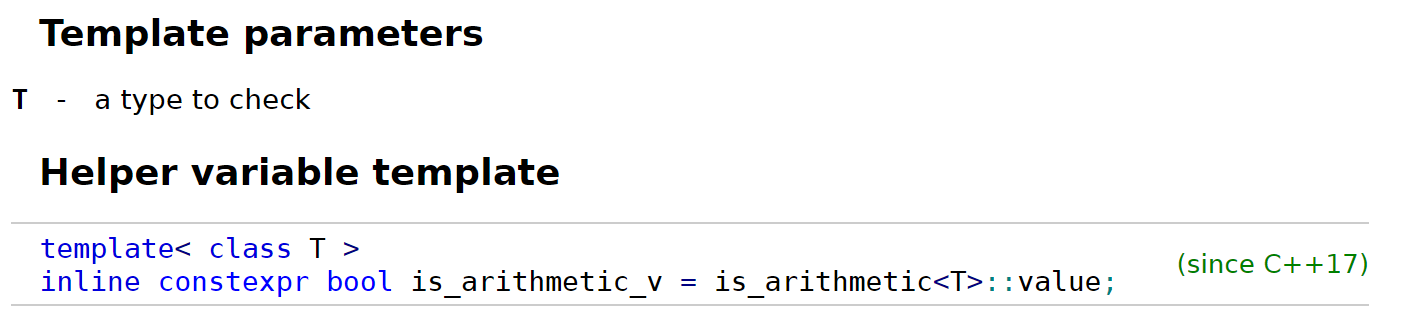template <typename T>
enable_if_t<is_convertible_v<T, string>, string> strOld(T t) {
return t;
}

template <typename T>
enable_if_t<!is_convertible_v<T, string>, string> strOld(T t) {
}


enable_if除了可以放在返回值的位置上以外，还可以直接使用在模板参数、函数参数中，或者在模板偏特化中出现，它的使用方式十分灵活，具体可以看官方文档：

// 2. the second template argument is only valid if T is an integral type:
template < class T,
class = typename std::enable_if<std::is_integral<T>::value>::type>
bool is_even (T i) {return !bool(i%2);}

// the partial specialization of A is enabled via a template parameter
template<class T, class Enable = void>
class A {}; // primary template

template<class T>
class A<T, typename std::enable_if<std::is_floating_point<T>::value>::type> {
}; // specialization for floating point types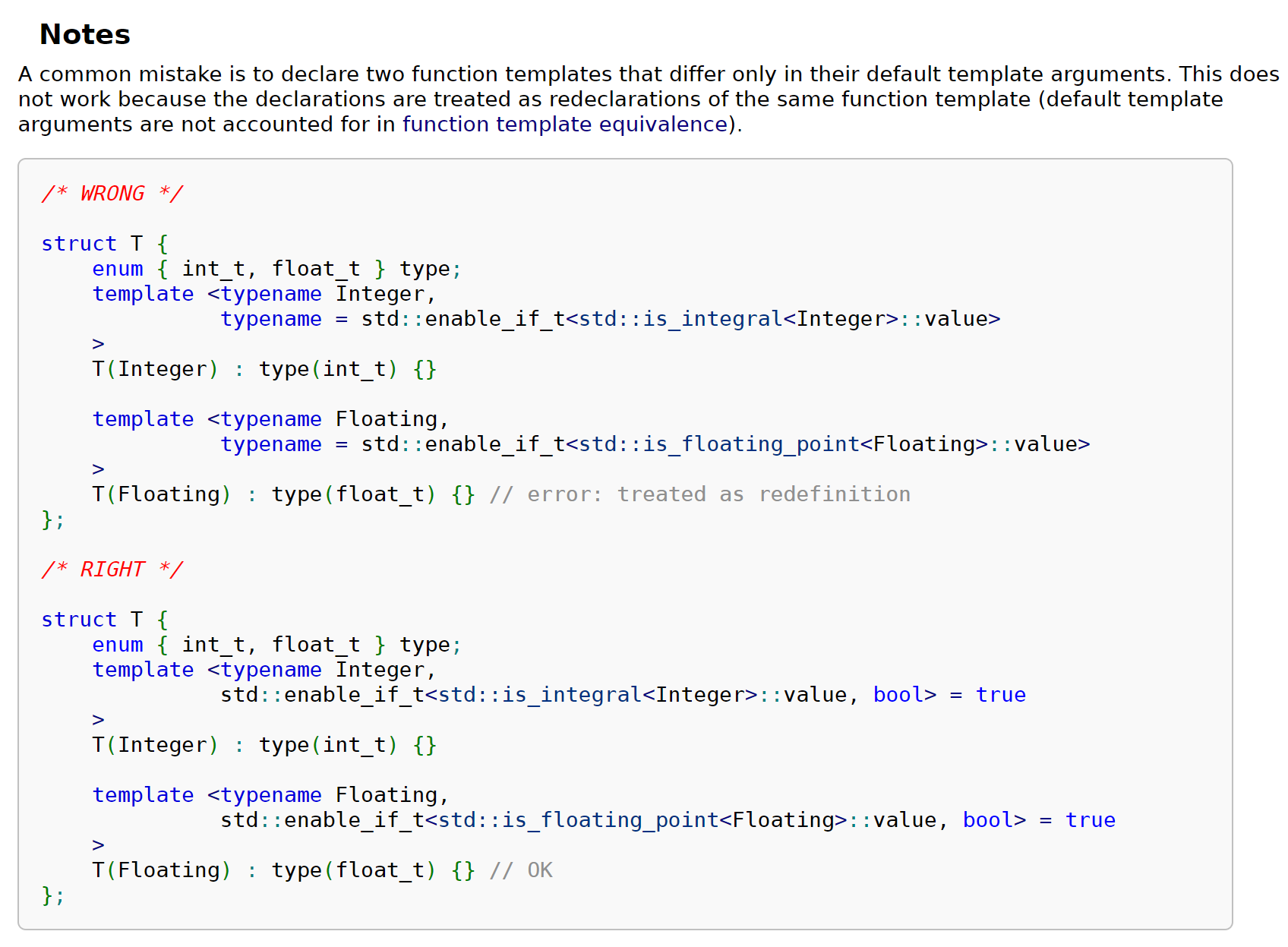###### if constexpr

C++17中，我们可以将if conxtexpr statement使用在模板中，起到与enable_if_t相同的作用：

template <typename T>
auto get_value(T t) {
if constexpr (std::is_pointer_v<T>)
return *t; // deduces return type to int for T = int*
else
return t;  // deduces return type to int for T = int
}

###### concepts-requires

// concept:
template<typename T>
concept Hashable = requires(T a) {
{ std::hash<T>{}(a) } -> std::convertible_to<std::size_t>;
};


• requires clauses
template<typename T>
void f(T&&) requires Eq<T>; // can appear as the last element of a function declarator

template<typename T> requires Addable<T> // or right after a template parameter list
T add(T a, T b) { return a + b; }

• requires expressions
template<typename T>
concept Addable = requires (T x) { x + x; }; // requires-expression

• simple requirements
template<typename T>
requires (T a, T b) {
a + b; // "the expression a+b is a valid expression that will compile"
};


• Type requirements
template<typename T> using Ref = T&;
template<typename T> concept C =
requires {
typename T::inner; // required nested member name
typename S<T>;     // required class template specialization
typename Ref<T>;   // required alias template substitution
};

• Compound requirements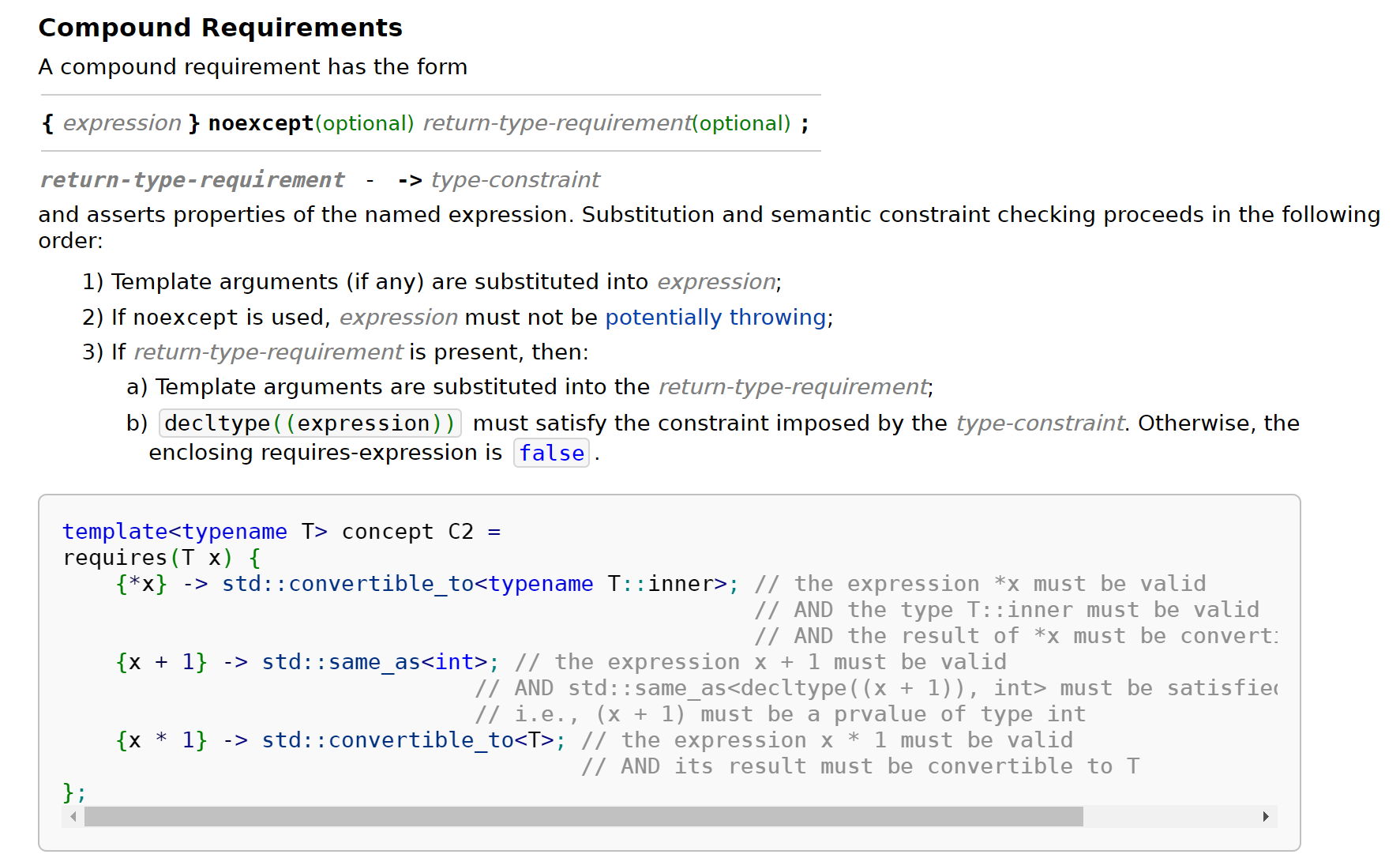• Nested requirements
###### Abbreviated function template

C++20始，提出了Abbreviated function template的做法，即使用placeholder types(auto, concept auto)出现在函数声明或者函数模板声明中：

void f1(auto); // same as template<class T> void f(T)
void f2(C1 auto); // same as template<C1 T> void f2(T), if C1 is a concept
void f3(C2 auto...); // same as template<C2... Ts> void f3(Ts...), if C2 is a concept
void f4(const C3 auto*, C4 auto&); // same as template<C3 T, C4 U> void f4(const T*, U&);
template <class T, C U>
void g(T x, U y, C auto z); // same as template<class T, C U, C W> void g(T x, U y, W z);


// requires:
template <typename T>
requires std::is_floating_point_v<T>
constexpr bool close_enough20(T a, T b) {
return absolute(a - b) < precision_threshold<T>;
}
constexpr bool close_enough20(auto a, auto b) {
return a == b;
}


constexpr bool close_enough20(std::floating_point auto a,
std::floating_point auto b) {
return absolute(a - b) < precision_threshold<std::common_type_t<decltype(a), decltype(b)>>;
}
constexpr bool close_enough20(std::integral auto a, std::integral auto b) {
return a == b;
}


### Template friends

#### Template class friends

template<typename T>
class foo{
template<typename U>
friend class foo_1;
};


Template parameters cannot shadow each other.

template<typename U>
friend class foo_1<U>;


template<typename T>
class foo{
friend class foo_1<T>;
};


template<class T> class A {}; // primary
template<class T> class A<T*> {}; // partial,因为此时T*仍然带有泛型T
template<> class A<int> {}; // full, int已经脱离T了，被“完全特化”了，所以模板参数中也不需要填东西
class X {
template<class T> friend class A<T*>; // error!
friend class A<int>; // OK
};


#### Template function friends

##### The Extrovert

template <typename T>
class Test {
template <typename U>      // all instantiations of this template are my friends
friend std::ostream& operator<<( std::ostream&, const Test<U>& );
};
template <typename T>
std::ostream& operator<<( std::ostream& o, const Test<T>& ) {
// Can access all Test<int>, Test<double>... regardless of what T is
}


##### The Introverts

template <typename T>
class Test {
friend std::ostream& operator<<( std::ostream& o, const Test& t ) {
// can access the enclosing Test. If T is int, it cannot access Test<double>
}
};


or the function template has to be declared as a template before the class body, in which case the friend declaration within Foo<T> can refer to the full specialization of operator<< for its T:

// Forward declare both templates:
template <typename T> class Test;
template <typename T> std::ostream& operator<<( std::ostream&, const Test<T>& );

// Declare the actual templates:
template <typename T>
class Test {
// refers to a full specialization for this particular T
friend std::ostream& operator<< <> (std::ostream&, const Test&);
// note: this relies on template argument deduction in declarations
// can also specify the template argument with operator<< <T>":
// friend std::ostream& operator<< <T>( std::ostream&, const Test<T>& );
};
// Implement the operator
template <typename T>
std::ostream& operator<<( std::ostream& o, const Test<T>& t ) {
// Can only access Test<T> for the same T as is instantiating, that is:
// if T is int, this template cannot access Test<double>, Test<char> ...
}


### Template meta-programming

#### Template Specialization

1. 在定义模板特例之前应当有模板通例（generic template/primary template）在先
2. 模板偏特化（部分特化）partial template specialization）只适用于class templatevariable template
3. 模板特化的匹配规则遵循以下顺序：
• If only one specialization matches the template arguments, that specialization is used

• If more than one specialization matches, partial order rules are used to determine which specialization is more specialized. The most specialized specialization is used, if it is unique (if it is not unique, the program cannot be compiled)

• If no specializations match, the primary template is used

#### Template Specialization Examples

// primary template
template <typename T> class TypeToID
{
public:
static int const ID = -1;
};
// specialization template
template <> class TypeToID<uint8_t>
{
public:
static int const ID = 0;
};


// use float specialization
template <> class TypeToID<float>
{
static int const ID = 0xF10A7;
};
// use void* specialization
template <> class TypeToID<void*>
{
static int const ID = 0x401d;
};


template <typename T>                    // 嗯，需要一个T
class TypeToID<T*>                        // 我要对所有的指针类型特化，所以这里就写T*
{
public:
typedef T         SameAsT;
static int const ID = 0x80000000;    // 用最高位表示它是一个指针
};


// float* -> T:float
template <typename T>
class RemovePointer<T*> {
public:
typedef T Result;
};

// const int -> T:int
template<typename T>
class RemovePointer<const T> {
public:
using type = T;
};


#### Name Resolution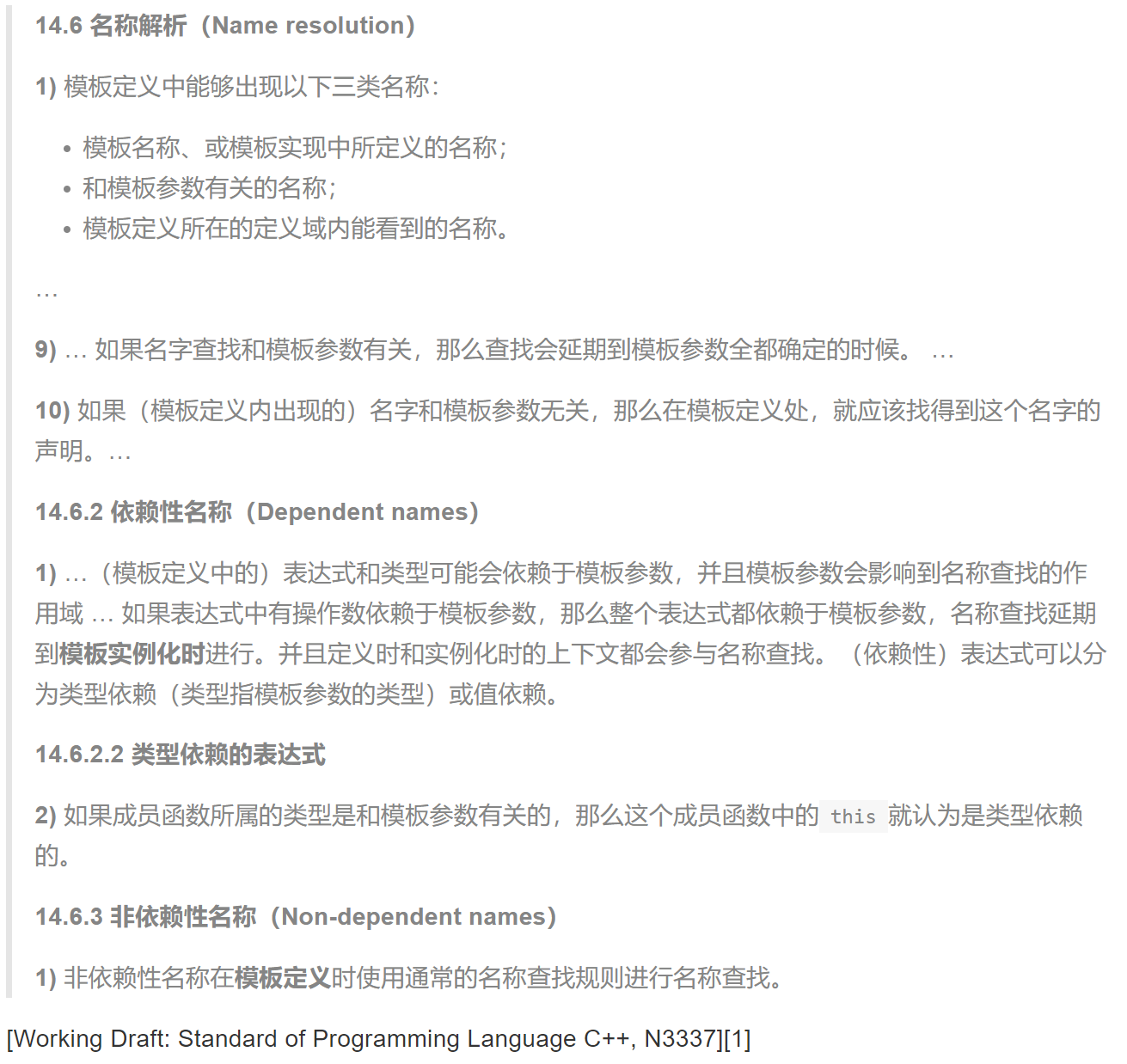A dependent name is a name that depends on the type or the value of a template parameter. For example

template<class T> class U : A<T>
{
typename T::B x;
void f(A<T>& y)
{
*y++;
}
};


- 除此之外，如果成员访问运算符(., ->)依赖于模板参数，则p->x中的x也是依赖性名称

- this->x在模板定义中出现时，也是依赖性名称;

- f(A<T>)中的f也是依赖性名称.

#include <iostream>
using namespace std;

void f(double) { cout << "Function f(double)" << endl; }

template <class A> struct container{ // point of definition of container
void member1(){
// This call is not template dependent,
// because it does not make any use of a template parameter.
// The name is resolved at the point of definition, so f(int) is not visible.
f(1);
}
void member2(A arg);
};

void f(int) { cout << "Function f(int)" << endl; }

void h(double) { cout << "Function h(double)" << endl; }

template <class A> void container<A>::member2(A arg){
// This call is template dependent, so qualified name lookup only finds
// names visible at the point of instantiation.
::h(arg);
}

template struct container<int>; // point of instantiation of container<int>

void h(int) { cout << "Function h(int)" << endl; }

int main(void){
container<int> test;
test.member1();
test.member2(10);
return 0;
}


Function f(double)
Function h(double)


name lookup中提到，名称查找又分为qualified name look-up以及unqualified name look-up。这样一来，在模板名称定义的概念里，我们有如下四种组合：

Because templates are compiled when required, this forces a restriction for multi-file projects: the implementation (definition) of a template class or function must be in the same file as its declaration. That means that we cannot separate the interface in a separate header file, and that we must include both interface and implementation in any file that uses the templates.

##### Why use typename?

template <typename T> struct X {};

template <typename T> struct Y
{
// X[T] is a dependent name on template parameter T
// which will be lookup during the template instantaition
// not the template definition period
typedef X<T> ReboundType;
typedef typename X<T>::MemberType MemberType2;    // 这里的typename是做什么的？
};


template<class T> class A
{
T::x(y);
typedef char C;
A::C d;
}


#### Type Computations

Computations on Types相对应的是Computations on Values:

int s = 3; // computations on values
using S = int; // computations on types

int triple = 3 * s; // computations on values
using cl_ref = const S&; // computations on types

int result = foo(triple); // computations on values
using result = std::remove_reference<cl_ref>::type; // computations on types

bool equals = (result = 0); // computations on values
constexpr bool equals = std::is_same<result, const int>::value; // computations on types

if (equals) exit(1); // computations on values
if constexpr (equals) exit(1); // computations on types


A meta-function is a “function” that operates on some types/values (parameters) and outputs some types/values (return values). Concretely, it is a struct that has public member types/fields which depend on what the template types/values are instantiated with.

// a value meta-function: identity
template<int V>
struct identity {
static const int value = V;
}

// a type meta-function: identity
template<typename T>
struct identity {
using type = T;
}


int val = identity<3>::value;


#### type_traits

// primary/generic template
template<typename T>
struct is_pointer {
static const bool value = false;
}

// specializaed template
template<typename T>
struct is_pointer<T*> {
static const bool value = true;
}


#### Examples

template<typename It>
size_t my_distance(It first, It last) {
// if it_category == random_access_Iterator
// then
// return last - first
else {
size_t result = 0;
while (first != last) {
++first;
++result;
}
return result;
}
}


template<typename It>
size_t my_distance(It first, It last) {
// notice! typename, lol we just introduced its effect
using category = typename std::iterator_traits<It>::iterator_category;
if (std::is_same<category, std::random_access_iterator_tag>::value) {
return last - first;
}
else {
size_t result = 0;
while (first != last) {
++first;
++result;
}
return result;
}
}


C++17中，很简单，我们只需做如下改写：

template<typename It>
size_t my_distance(It first, It last) {
// notice! typename, lol we just introduced its effect
using category = typename std::iterator_traits<It>::iterator_category;
if constexpr (std::is_same<category, std::random_access_iterator_tag>::value) {
return last - first;
}
else {
size_t result = 0;
while (first != last) {
++first;
++result;
}
return result;
}
}


### Lambda Expressions

1话题的引入来自于该函数中的predicate，我们尝试着将它写做一个非固定最大值的版本，即将下边的5替换为一个limit变量：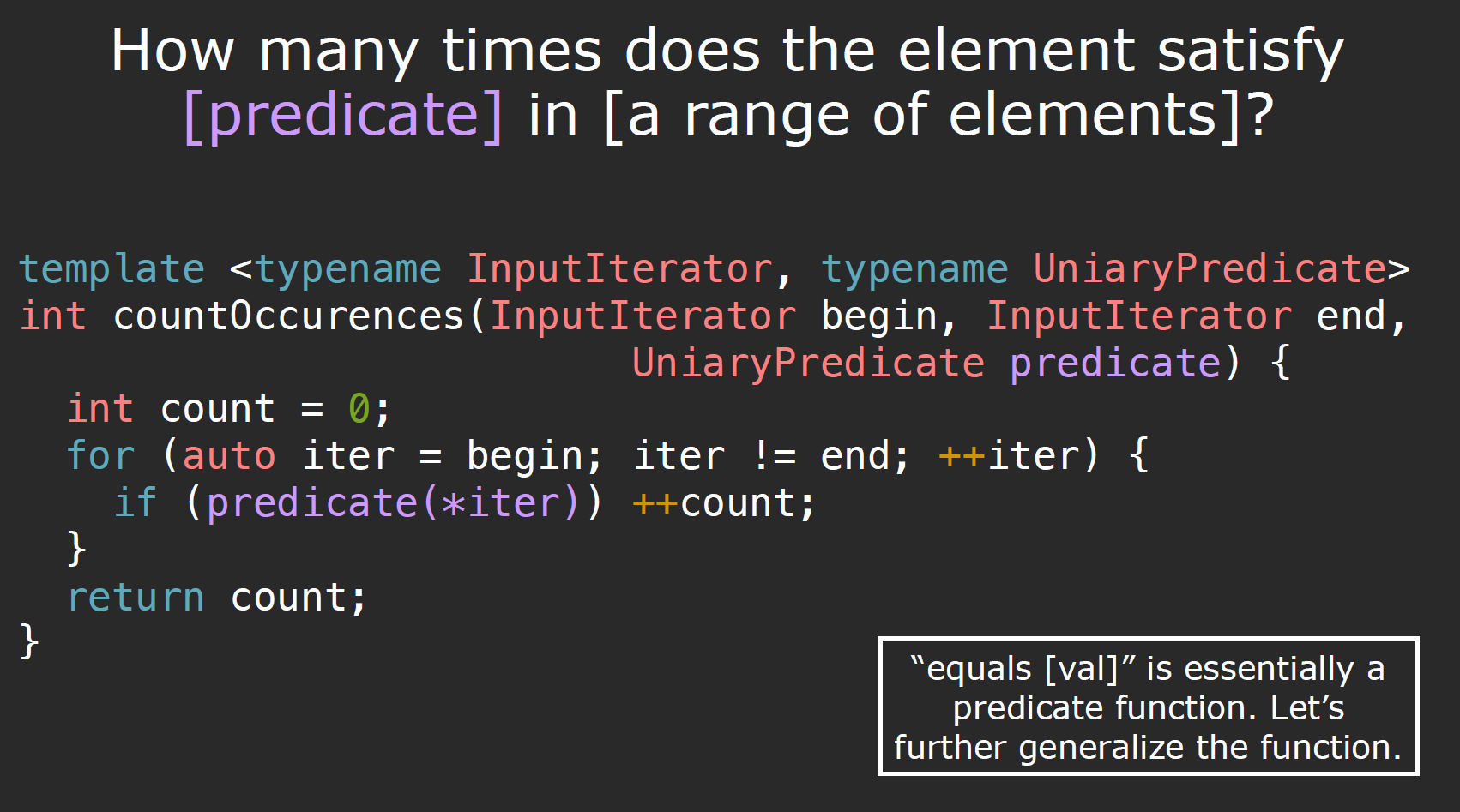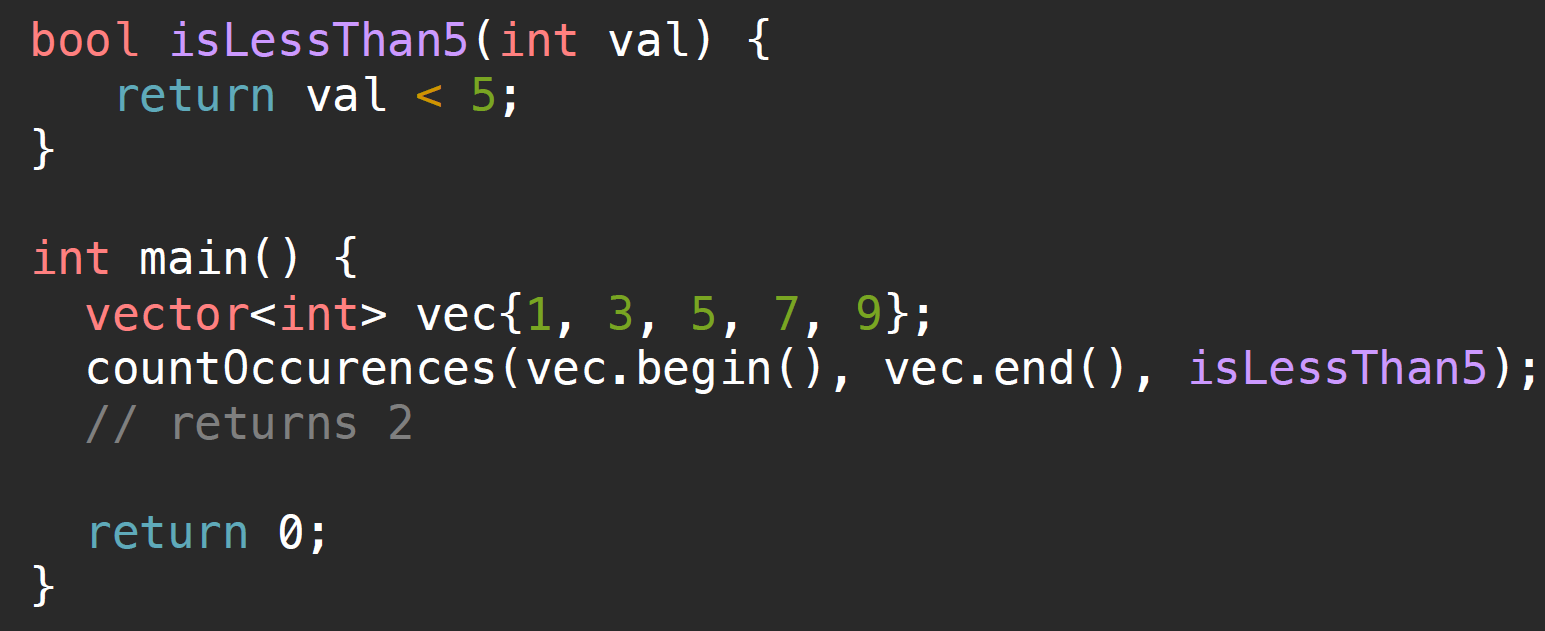call it by using function pointers

#### Pre-C++11(Functors)

C++11之前，我们使用如下方法，这也是我们所说的闭包，之后lambda表达式的基础或者说内部形式：

// by value
class GreaterThan {
public:
GreaterThan(int limit) : limit(limit) {}
// note: this should have a const
bool operator() (int val) const {return val >= limit};
private:
int limit; /* the value we captured outside*/
}

// by reference
class GreaterThan {
public:
GreaterThan(int &limit) : limit(limit) {}/* pass by reference */
// note: this should have a const
bool operator() (int val) const {return val >= limit};
private:
int& limit; /* the value we captured outside, by reference*/
}


C++11中引入了lambda expression:

auto func = [capture-clause](parameters) -> return-value {
// body
};

// the simplest lambda:
[]{};

• return-value is optional
• can use = to capture all by value (not recommend)
• can use & to capture all by reference (not recommend)

auto identifier(argument) -> return type


template<typename T1, typename T2>
auto compose(T1 a, T2 b) -> decltype(a + b);


C++11中规定，如果lambda函数体内部出现了除了return额外的语句，则lambda对象默认返回void，所以我们需要-> return type指定返回类型.

#### C++14

1. can use auto as the parameters to templatize the lambda–generic lambda
auto p = [](auto a) {cout << a << endl; };

1. can capture with an initialiser–z = x + y

#### C++17

1. use constexpr :
constexpr auto Square = [](int n) { return n * n; };

1. can capture *this

#### C++20

We can pass a template tail:

auto ForwardToTestFunc = []<typename ...T>(T&& ...args) {
return TestFunc(std::forward<T>(args)...);
};


#### mutable

void operator()(...) const {
// since there is a const,
// if captured by reference, we can change the values captured
// if captured by value, we cannot change it.
}


class a {

public:
a(int x1): x(x1) {}
mutable int x;

bool changeX(int y) const {
x = y;
}
};


#### globals & statics

lambda表达式仅允许捕捉当前函数中定义的具有automatic storage duration的变量，所以我们不能够捕捉global/static variables，对于定义在当前函数之外的名字，lambda可以直接使用。

##### storage duration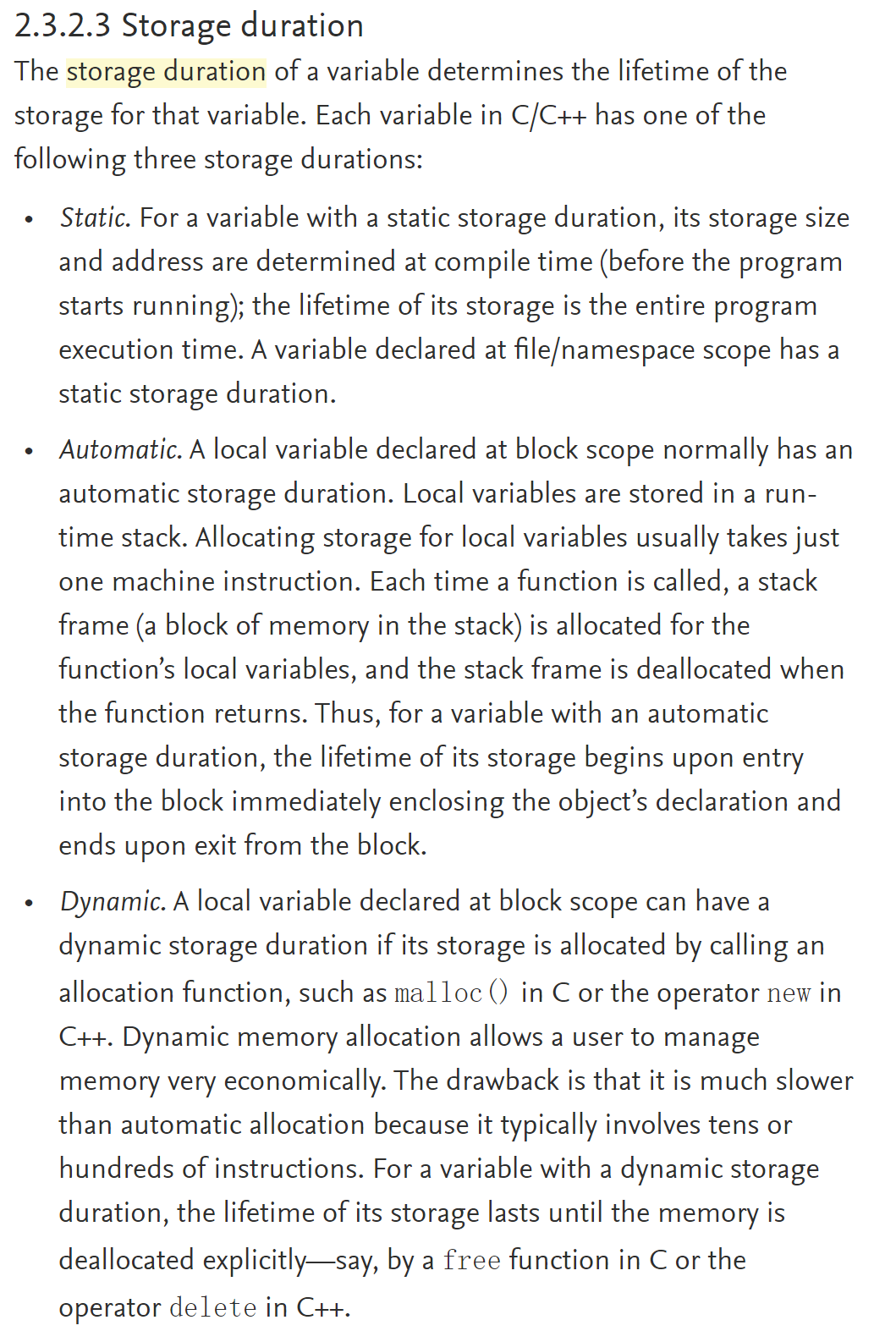// a string literal
char *str = "ABCDEFG";
// NOT a string literal -- 储存在栈上
char str[] = "ABCDEFG";


#### type of lambda?

C++20之前，答案是不行。从定义上来说，lambda表达式的类型是闭包类型（closure type），如果我们尝试使用typeid关键字获取其类型，则即使是同一个类型的lambda对象，每一个lambda对象的typeid值也是不同的：

The type of the lambda-expression (which is also the type of the closure object) is a unique, unnamed non-union class type — called the closure type.

auto f = [](int x){ return x; };
decltype(f) g = f;


decltype([](int x){ return x; })    // error


int(* f)(int, int) = [](int a, int b){ return a + b; };


#### Pass Lambda As Argument?

template< class ForwardIt, class T >
constexpr std::pair<ForwardIt,ForwardIt>
equal_range( ForwardIt first, ForwardIt last,
const T& value );

##### std::function

// normal functions
int add(int i, int j) { return i + j; }
// lambda
auto mod = [](int i, int j) { return i % j; };
// function object class
struct divide {
int operator()(int denominator, int divisor) {
return denominator / divisor;
}
}


std::function<double(double, double)> f_mul = [a](double x, double y) { return x * y + a; };

double calculator(double a, double b, std::function<double(double, double)> fn)
{
return fn(a, b);
}


Class template std::function is a general-purpose polymorphic function wrapper. Instances of std::function can store, copy, and invoke any CopyConstructible Callable target – functions, lambda expressions, bind expressions, or other function objects, as well as pointers to member functions and pointers to data members.

### std::bind

using namespace std::placeholders;  // for _1, _2, _3...

std::cout << "1) argument reordering and pass-by-reference: ";
int n = 7;
// (_1 and _2 are from std::placeholders, and represent future
// arguments that will be passed to f1)
auto f1 = std::bind(f, _2, 42, _1, std::cref(n), n);
n = 10;
f1(1, 2, 1001); // 1 is bound by _1, 2 is bound by _2, 1001 is unused
// makes a call to f(2, 42, 1, n, 7)


std::reference_wrapper is a class template that wraps a reference in a copyable, assignable object. It is frequently used as a mechanism to store references inside standard containers (like std::vector) which cannot normally hold references.

std::vector<std::reference_wrapper<int>> v(l.begin(), l.end());


bind()函数会给原函数创建一个call wrapper，调用wrapper函数相当于invoke f with some of its arguments bound to args.值得注意的是，bind函数声明也是通过可变函数参数实现的。

1C++11之前，如果我们想让一个函数接受任意参数，那么我们需要使用...的语法配合va_系列的宏来使用，文档给出了一个例子：

#include <iostream>
#include <cstdarg>

void simple_printf(const char* fmt...) // C-style "const char* fmt, ..." is also valid
{
va_list args;
va_start(args, fmt);

while (*fmt != '\0') {
if (*fmt == 'd') {
int i = va_arg(args, int);
std::cout << i << '\n';
} else if (*fmt == 'c') {
// note automatic conversion to integral type
int c = va_arg(args, int);
std::cout << static_cast<char>(c) << '\n';
} else if (*fmt == 'f') {
double d = va_arg(args, double);
std::cout << d << '\n';
}
++fmt;
}

va_end(args);
}

int main()
{
simple_printf("dcff", 3, 'a', 1.999, 42.5);
}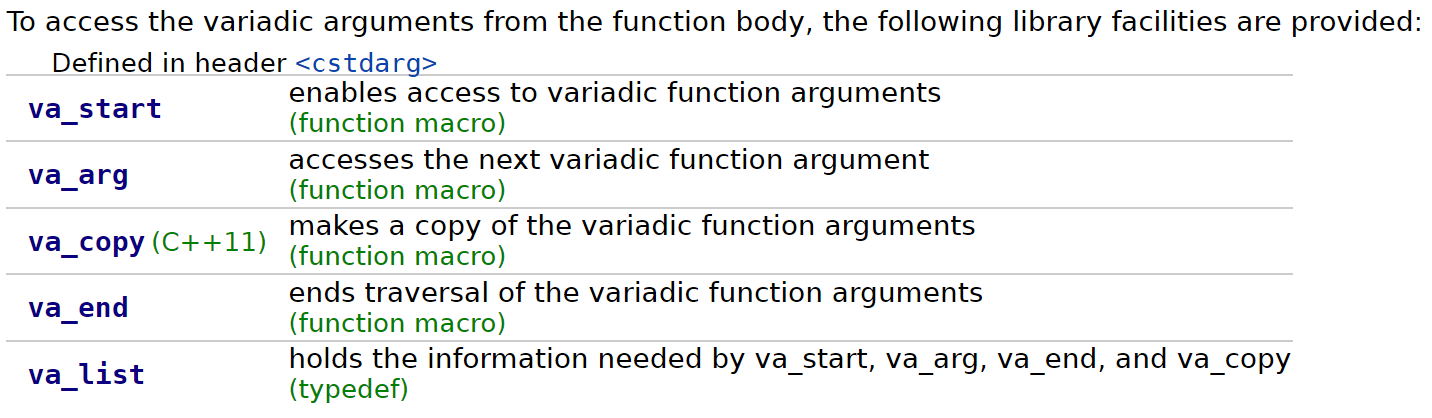void va_start( std::va_list ap, parm_n );


int printz(...)


fallback is a function that does not take any arguments and does not return anything.

#### Parameter pack

Note: this is different from a function parameter pack expansion, which is indicated by an ellipsis that is a part of a parameter declarator, rather than an ellipsis that appears after all parameter declarations. Both parameter pack expansion and the “variadic” ellipsis may appear in the declaration of a function template, as in the case of std::is_function.

// variadic arguments
int printx(const char* fmt...);
// parameter pack
void foo(const T &t, const Args&... rest);


parameter pack是构成variadic template的基础：

A template with at least one parameter pack is called a variadic template.

template <typename T, typename... Args> // a template parameter packet
void foo(const T &t, const Args&... rest); // a function parameter packet


#### Packet expansion

template<class ...Us> void f(Us... pargs) {}
template<class ...Ts> void g(Ts... args) {
f(&args...); // “&args...” is a pack expansion
// “&args” is its pattern
}
g(1, 0.2, "a"); // Ts... args expand to int E1, double E2, const char* E3
// &args... expands to &E1, &E2, &E3
// Us... pargs expand to int* E1, double* E2, const char** E3


f(&args...); // expands to f(&E1, &E2, &E3)
f(n, ++args...); // expands to f(n, ++E1, ++E2, ++E3);
f(++args..., n); // expands to f(++E1, ++E2, ++E3, n);
f(const_cast<const Args*>(&args)...);
// f(const_cast<const E1*>(&X1), const_cast<const E2*>(&X2), const_cast<const E3*>(&X3))
f(h(args...) + args...); // expands to
// f(h(E1,E2,E3) + E1, h(E1,E2,E3) + E2, h(E1,E2,E3) + E3)


// base case function
template<typename T>
ostream &print(ostream &os, const T &t) {
return os << t;
}

template<typename T, typename... Args>
// the first packet expansion, using pattern const Args&
ostream& print(ostream &os, const T &t, const Args&... rest) {
os << t << ", ";
// the second packet expansion, using pattern rest
// then peel off the first element in the expansion list to be const T &t
// recursion with the list with other elements
return print(os, rest...);
}


#### Performance

If you’re concerned with the performance of code that relies on variadic templates, worry not. As there’s no actual recursion involved, all we have is a sequence of function calls pre-generated at compile-time. This sequence is, in practice, fairly short (variadic calls with more than 5-6 arguments are rare). Since modern compilers are aggressively inlining code, it’s likely to end up being compiled to machine code that has absolutely no function calls. What you end up with, actually, is not unlike loop unrolling.

Compared to the C-style variadic functions, this is a marked win, because C-style variadic arguments have to be resolved at runtime. The va_ macros are literally manipulating the runtime stack. Therefore, variadic templates are often a performance optimization for variadic functions.

### range

C++20中，提出了range的概念，写了一个range库，其中包含range, view, range adaptor等……但这里不打算深究，具体详见官方文档以及这篇文章

## Object-Oriented Programming

### Const

// 指向整型常量的指针(可修改指针本身，不可修改指针指向的对象)
const int* a;
// or:
int const* a;

// 指向整形的常量指针(不可修改指针本身，可修改指针指向的对象)
int* const a;

// 根据《C++ Primer》，this指针默认为指向非常量对象的常量指针，
// 此操作将this指针设定为指向常量对象的常量指针
void func() const


#### Why initialization-list?

test(int num1, double str1): num(num1), str(str) {};


class foo {
public:
foo(size_t size):
size{size},
vec{size, nullptr} {}
private:
vector<int*> vec;
size_t size;
};


### friend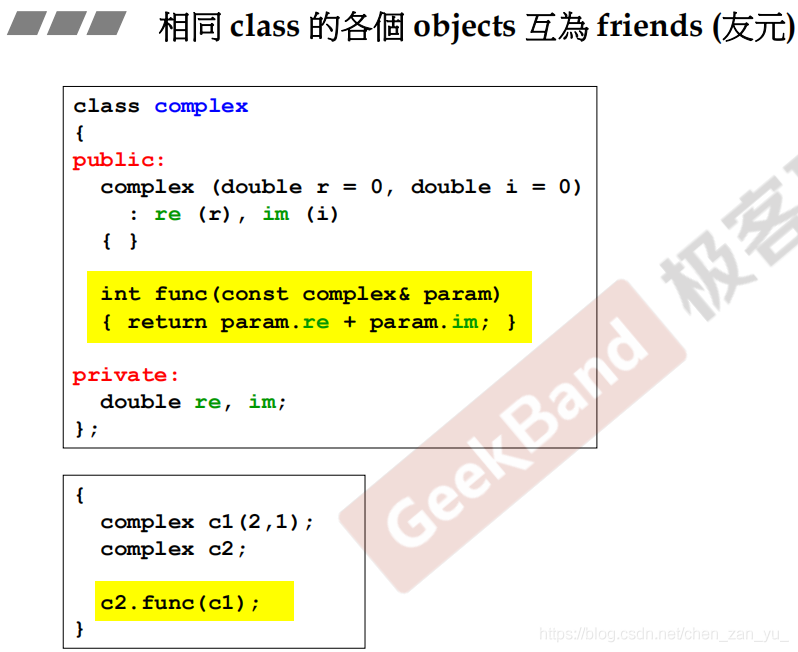#### Mutual friends class

A, B两个类互为友元时，我们称这两个类为Mutual Friends Classes。为了解决这个问题，我们首先要知道，当一个类仅有声明，而没有定义时，类为不完整类型。我们仅能够使用指向该类的指针、引用或者将类作为形参和返回值出现在函数声明中。

##### one file

1. 作类B前置声明forward declaration），告知类A存在一个名为B的类；
2. 定义类A，在类A中将类B声明为友元，对于需要用到类B的函数，仅提供函数声明，因为此时我们还不知道B的具体定义；
3. 定义类B，提供完整定义；
4. 将类A中需要调用B的方法定义补充完整
// Forward Declaration
class B;

// Class A
class A {

// Member of class A
int data;

public:
// Make B as a friend of class A
friend class B;

// Constructor to initialise member
// of class A
A(int d) { data = d; }

// Function to get data of friend
// class B
void get_data_B(B objb);
};

// Class B
class B {

// Member of class B
int dataB;

public:
// Making A a friend of class B
friend class A;

// Constructor to initialise member of
// class B
B(int d) { dataB = d; }

// Function to get the data of
// friend class A
void get_data_A(A obja)
{
cout << "Data of A is: "
<< obja.data;
}
};

// Function for accessing friend class
// B's object
void A::get_data_B(B objb)
{
cout << "Data of B is: "
<< objb.dataB;
}

##### separate file

• A.h
/* This is called a "forward declaration".  We use it to tell the compiler that
the identifier "B" will from now on stand for a class, and this class will be
defined later.  We will not be able to make any use of "B" before it has been
defined, but we will at least be able to declare pointers to it. */
class B;

class A
{
/* We cannot have a field of type "B" here, because it has not yet been
defined. However, with the forward declaration we have told the compiler
that "B" is a class, so we can at least have a field which is a pointer
to "B". */
B* pb;
}

• B.h
#include "A.h"

class B
{
/* the compiler now knows the size of "A", so we can have a field
of type "A". */
A a;
}

##### template mutual friends

hashmap的作业里，我们看到了这种用法：两个文件hashmap.hhashmap_iterator.h中，前一个文件对后一个文件，即提供了迭代器类的文件做了包含，但是在hashmap_iterator.h中我们发现，即使不提供HashMap的前置声明，运行也不会报错，这是为什么呢？

1. HashMap中的类型别名定义
2. HashMap的静态成员

// friend class, class type foo as template parameter
template<typename foo>
class foo_1 {
// since foo will be a complete type even if it's a template class,
// we can directly write 'foo'
friend foo;
// the type of vector, defined in the hashmap class
// since it's a type, we can directly use :: to get it here!
using bucket_array_type = foo::bucket_array_type;

// thyen, make a pointer!
bucket_array_type* _buckets_array;

// in iterator class, we shall use private constructor
// iterator's constructor, we will use it in the
// hashmap class
foo_1(bucket_array_type* _buckets_array):
_buckets_array(_buckets_array) {}

public:
// some member functions
};

class foo {
private:
vector<node*> _buckets_array;

using bucket_array_type = decltype(_buckets_array);
// foo_1's template specialization of <foo> type
friend class foo_1<foo>;

public:
foo(/* */);
foo_1 begin() {
// call iterator constructor here
/** give the address of the vector to initialize the
* pointer pointing to vector<> type in the iterator
* class */
return {&_buckets_array};
}
};


### Operators

#### General rule of thumb

1. Some operators must be implemented as members ( eg. [], (), ->, =) due to C++ semantics.

1. Some must be implemented as non-members (eg. «, if you are writing class for rhs, not lhs).

1. If unary operator (eg. ++), implement as member.

2. If binary operator and treats both operands equally (eg. both unchanged) implement as non-member (maybe friend). Examples: +, <.

Binary operators are typically implemented as non-members to maintain symmetry (for example, when adding a complex number and an integer, if operator+ is a member function of the complex type, then only complex+integer would compile, and not integer+complex).

A corresponding property exists for binary relations; a binary relation is said to be symmetric if the relation applies regardless of the order of its operands.

// friends defined inside class body are inline and are hidden from non-ADL lookup
friend X operator+(X lhs,        // passing lhs by value helps optimize chained a+b+c
const X& rhs) // otherwise, both parameters may be const references
{
lhs += rhs; // reuse compound assignment
return lhs; // return the result by value (uses move constructor)
}


template<typename K, typename M, typename H=std::hash<K>>
class foo {
// do something
template<typename K_, typename M_, typename H_>
friend std::ostream& operator<<(std::ostream& os, const foo<K_, M_, H_>& foo_1);
};

1. If binary operator and not both equally (changes lhs), implement as member (allows easy access to lhs private members). Examples: +=
  X& operator+=(const X& rhs) // compound assignment (does not need to be a member,
{                           // but often is, to modify the private members)
/* addition of rhs to *this takes place here */
return *this; // return the result by reference
}


#### ->

HashMap的作业中，我们需要在iterator类中对成员访问操作符进行重载。该运算符的重载可能看起来有些奇怪，实际上，运算符重载的结果不是简单的替换原则，比如我们可能认为obj->value重载替换之后应当被理解为ptrvalueptr是私有指针成员），但实际上该运算符的重载结果为：

(obj.operator->())->value;


obj是一个类对象，它使用运算符重载函数operator->()之后，我们知道一般是要返回一个指针（比如在迭代器的实现中，是利用迭代器内置指针指向对应的容器中的数据的一个指针），之后程序会在该运算符重载函数返回值上调用指针自己的->运算符来获取指向的数据成员

#### []

const string& vector<string>::operator[](size_t index) const {
// do something
}


#### Principle of Least Astonishment (POLA)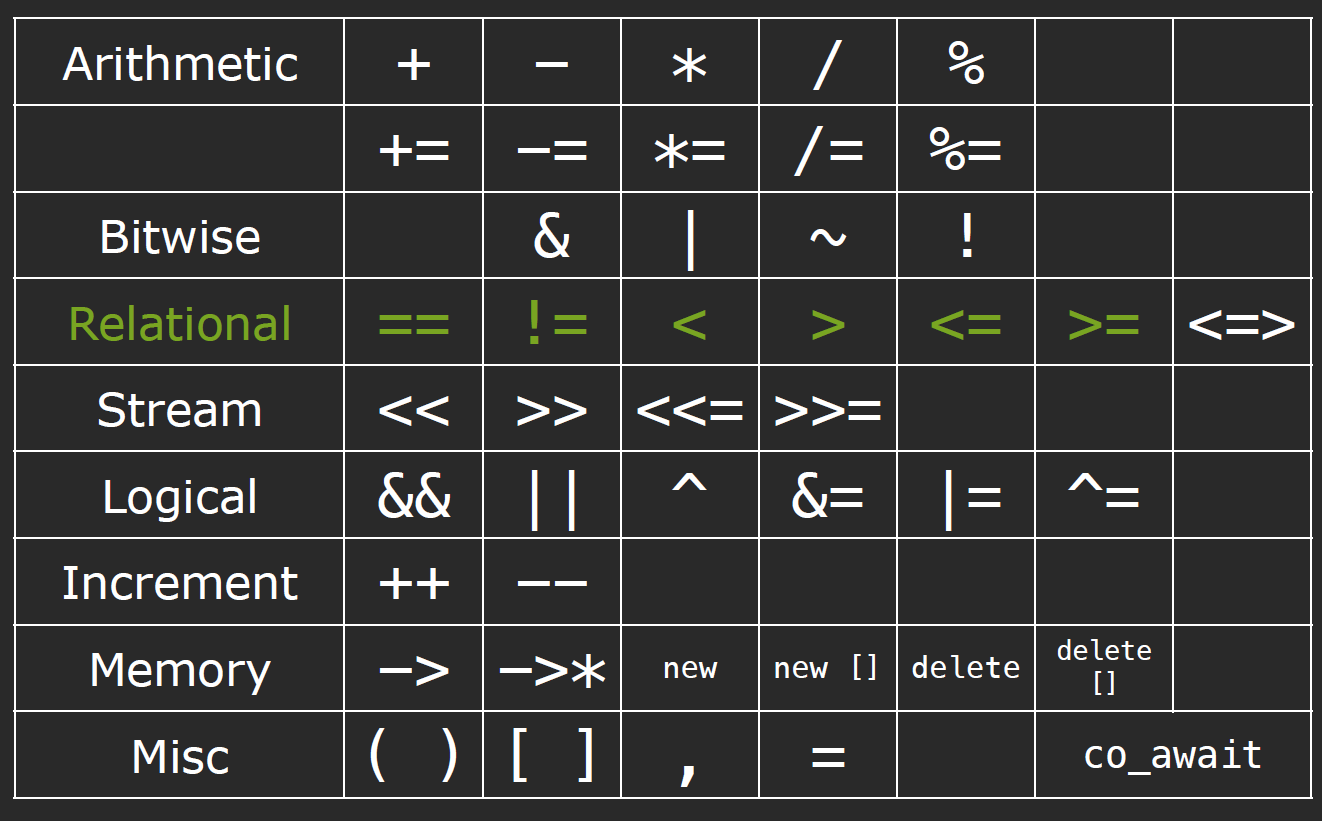ostream& operator<<(ostream& out, const Fraction& f) {
out << f.num << “/”<< f.denom;
return os;
}


#### Converting constructor & Conversion operator

##### Converting constructor

C++ PrimerP264:

class test_explicit {
public:
test_explicit(string text): text(text) {}
string combine(const test_explicit& new_text) {
return text + new_text.text;
}
private:
string text;
};
// OK, implicit conversion:
string new_value = "9999";
cout << "Combine value: " << foo_1.combine(new_value) << endl;

// error, only permit one conversion:
cout << "Combine value: " << foo_1.combine("9999") << endl;


class test_explicit {
public:
explicit test_explicit(int i): data(i) {}
int combine(const test_explicit& new_value) {
return data + new_value.data;
}
private:
int data;
};

// error, try to converting implicitly from int to test_explicit type
string new_value = "9999";
cout << "Combine value: " << foo_1.combine(new_value) << endl;


string null_book = "9999";
Sales_data item2 = null_book; //error


explicit可以避免我们写出二义性的代码。

##### Conversion operator

explicit关键字除了可以用在构造函数之前外，还可以用在类型转换运算符前：

explicit operator double() const {
// do something
}


operator type() const;


int i = 42;
cin << i;


### Special member functions

• Default construction
• Copy construction
• Copy assignment
• Destruction

#### Most vexing parse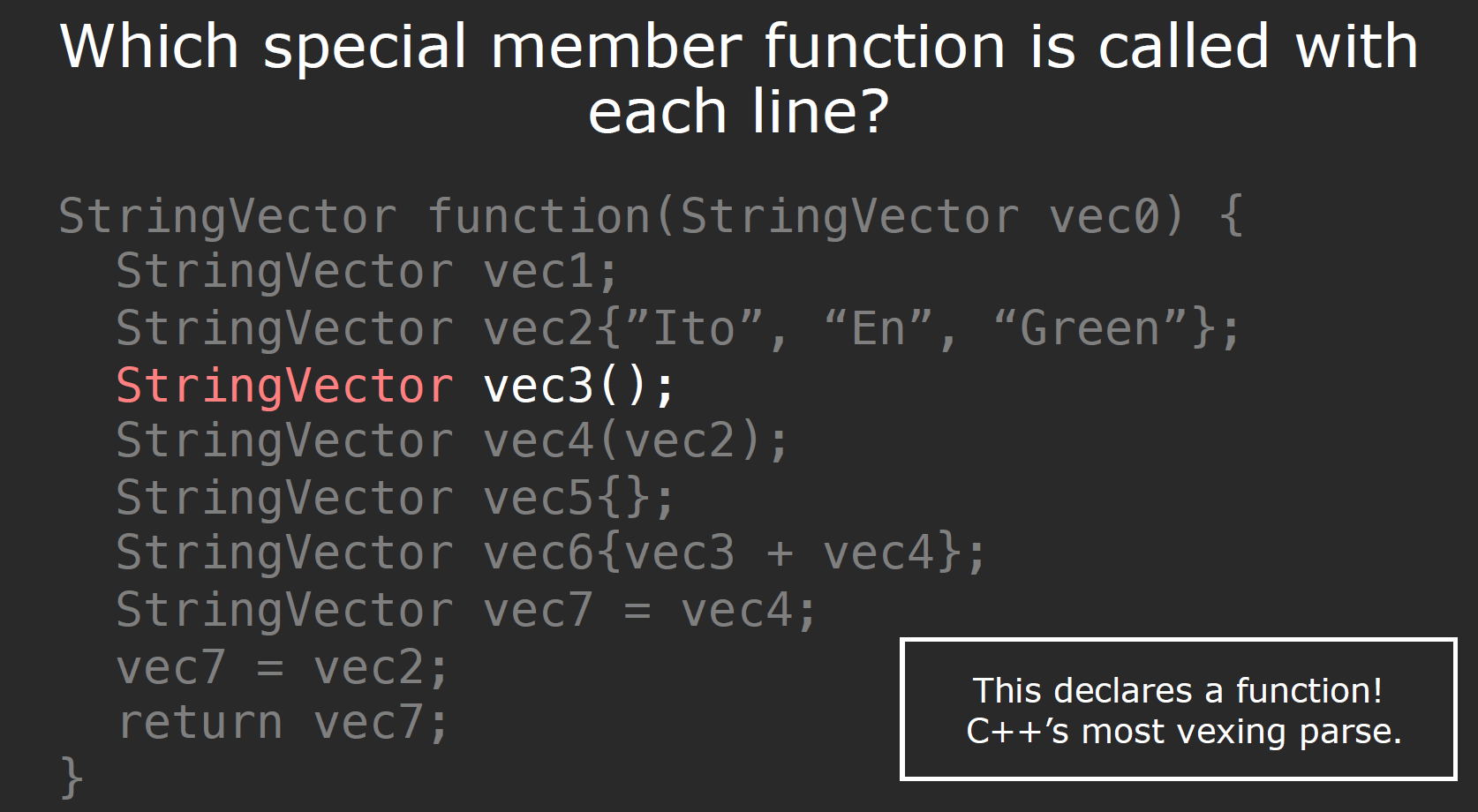The most vexing parse is a counterintuitive form of syntactic ambiguity resolution in the C++ programming language. In certain situations, the C++ grammar cannot distinguish between the creation of an object parameter and specification of a function’s type. In those situations, the compiler is required to interpret the line as a function type specification.

#### shallow copy & deep copy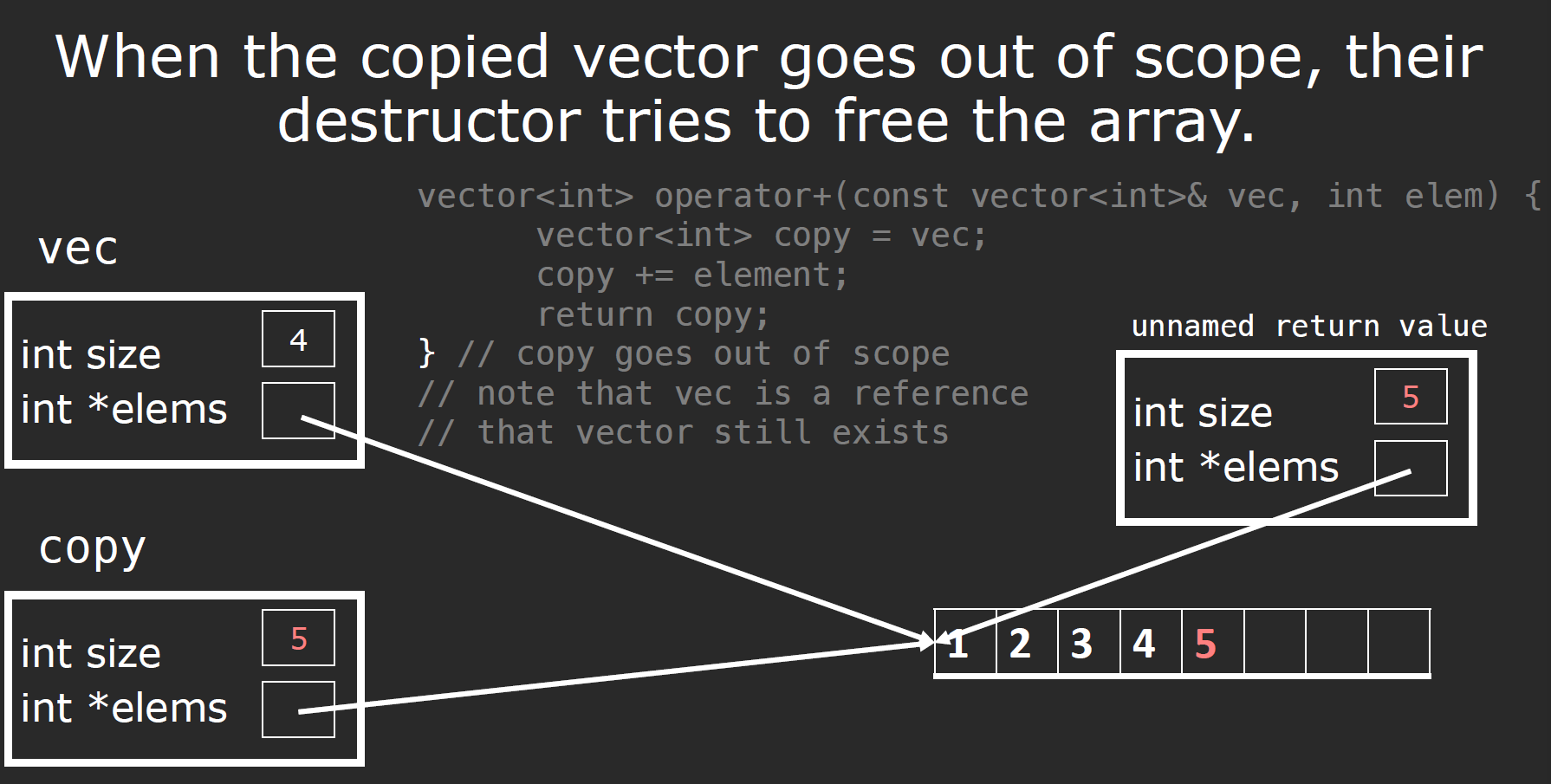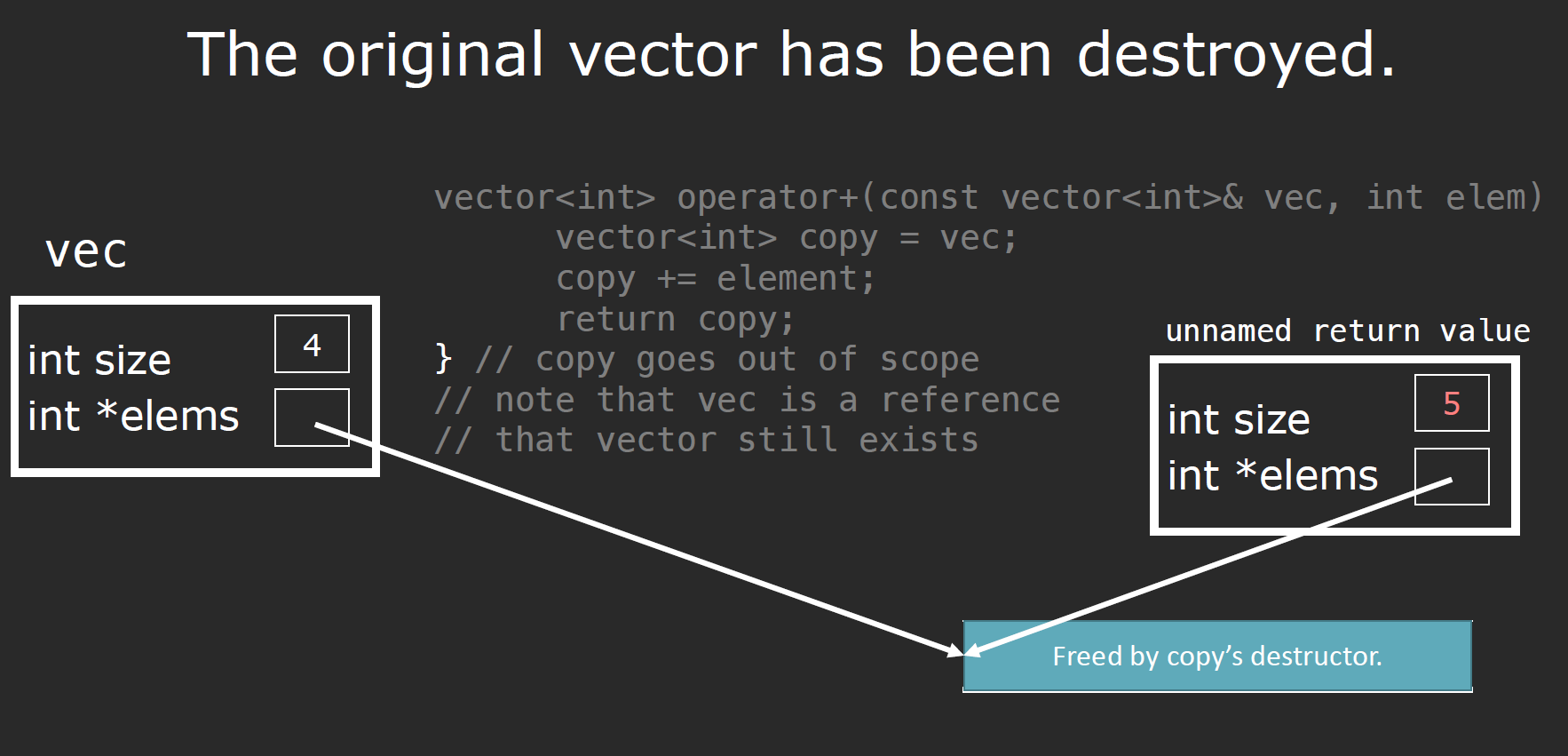#### Copy constructor

StringVector::StringVector(const StringVector& other) :
_logicalSize(other._logicalSize),
_allocatedSize(other._allocatedSize) {

_elems = new ValueType[_allocatedSize];
std::copy(other.begin(), other.end(), begin());
}


#### Copy assignment

StringVector& StringVector::operator=(const StringVector& rhs) {
if (this != &rhs) {
delete [] _elems;
_logicalSize = rhs._logicalSize;
_allocatedSize = rhs._allocatedSize;
_elems = new ValueType[_allocatedSize];
std::copy(other.begin(), other.end(), begin());
}
return *this;
}


#### =delete & =default

test(const test&) =delete;
test& operator=(const test&) =delete;


test() = default;


#### Write our own?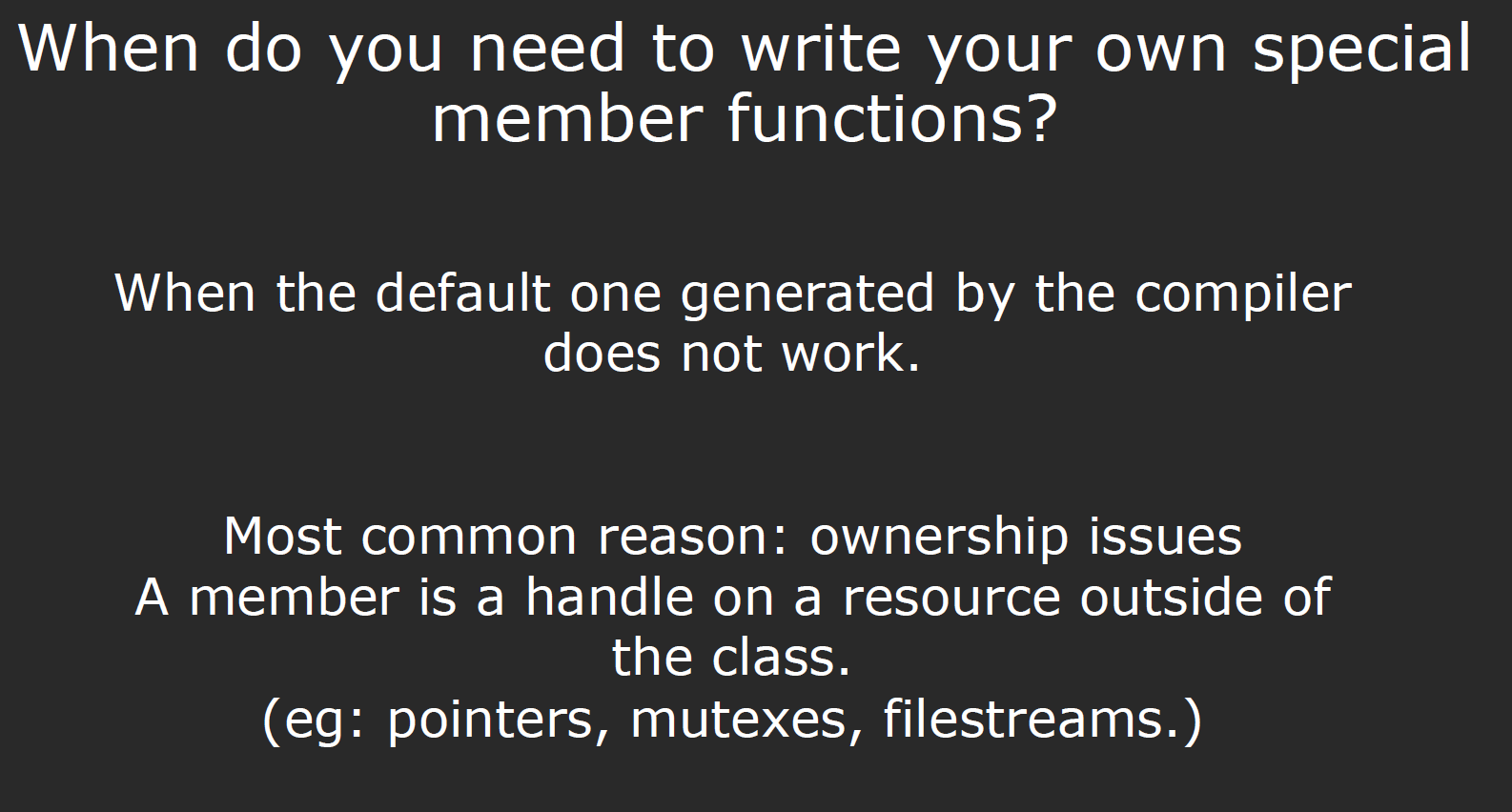#### Rule Of Three

If you explicitly define (or delete) a copy constructor, copy assignment, or destructor, you should define (or delete) all three.

#### Rules of Zero

If the default operations work, then don’t define your own custom ones.

### Delegating Constructor

C++11引入了委托构造函数–使用它所属类的其他构造函数执行他自己的初始化过程，或者将自己的职责委托给其他构造函数：

class Sales_data {
public:
Sales_data(): Sales_data("", 0, 0) {}
// other constructors
}


### inline & constexpr

constexpr函数是指能用于常量表达式的函数：它的返回类型、所有的形参类型都是字面值类型，而且函数体中除了using/typedef等在运行时不执行任何操作的语句外，只能有一条return语句：

constexpr int new_sz() {return 42;}
constexpr int foo = new_sz(); // foo is a const expression


constexpr size_t scale(size_t cnt) { return new_sz() * cnt;}
// OK
int arr[scale(2)];
// ERROR
int i = 2;
int arr[scale(i)];


### Move semantics

#### emplace_back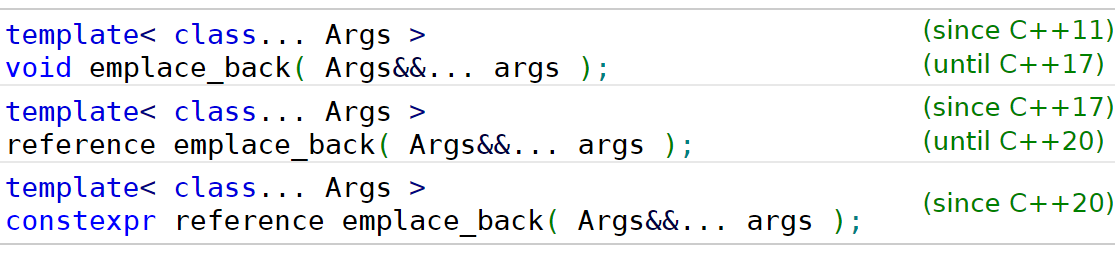std::vector<President> elections;
std::cout << "emplace_back:\n";
auto& ref = elections.emplace_back("Nelson Mandela", "South Africa", 1994);
assert(ref.year == 1994 && "uses a reference to the created object (C++17)");

std::vector<President> reElections;
std::cout << "\npush_back:\n";
reElections.push_back(President("Franklin Delano Roosevelt", "the USA", 1936));


#### Without copy elision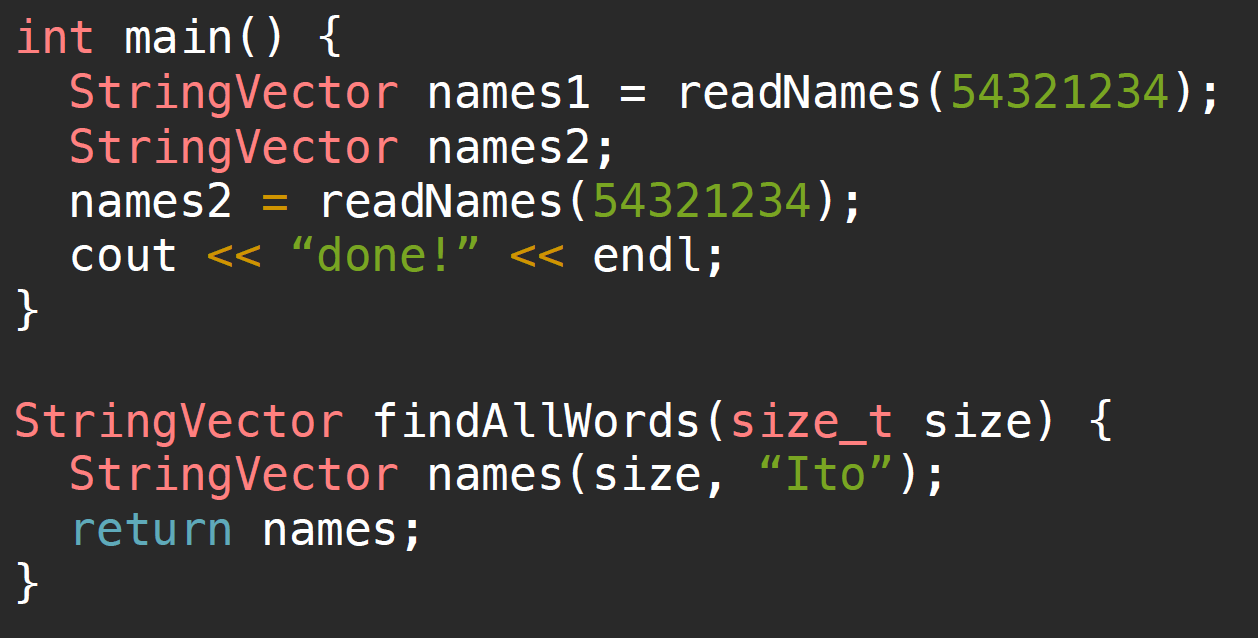#### Basic idea

X& X::operator=(X const & rhs)
{
// [...]
// Make a clone of what rhs.m_pResource refers to.
// Destruct the resource that m_pResource refers to.
// Attach the clone to m_pResource.
// [...]
}


x foo();
// do something
x = foo();


X& X::operator=(<mystery type> rhs)
{
// [...]
// swap this->m_pResource and rhs.m_pResource
// [...]
}


rvalues must prefer the mystery type, while lvalues must prefer the ordinary reference.

Rvalue references allow a function to branch at compile time (via overload resolution) on the condition “Am I being called on an lvalue or an rvalue?”

X& X::operator=(X&& rhs)
{

// Perform a cleanup that takes care of at least those parts of the
// destructor that have side effects. Be sure to leave the object
// in a destructible and assignable state.

// Move semantics: exchange content between this and rhs

return *this;
}


std::move正是通过强制将左值转换为右值的方法，使得外部函数可以调用如上所示的右值重载，使得等式左边的对象获得给入的右对象的实际控制权：

struct A {
A(A&& a) {
this->data = a->data;
a->data = nullptr;
}
};


PS: 这也是为什么我们说在进行移动后，我们不能对源对象的值做任何假设（在后边有提到）的原因，右对象的控制权（指针）已经被交出去了.

#### lvalues & rvalues

1. type(e.g. int, double, class, …)// forward:
template<class S>
S&& forward(typename remove_reference<S>::type& a) noexcept
{
return static_cast<S&&>(a);
}


1. value category

##### lvalue

An lvalue is an expression that has a name/identity. In other words, we can find address using address-of operator(&var)

##### rvalue

An rvalue is an expression that does not have a name/identity.

• temporaray values

##### l/rvalue reference

// rvalue reference
// here, v1 + v2 is a temporary object, which is a rvalue
auto&& v4 = v1 + v2;


const auto& ptr3 = ptr + 5;


Normally, a temporary object lasts only until the end of the full expression in which it appears. However, C++ deliberately specifies that binding a temporary object to a reference to const on the stack lengthens the lifetime of the temporary to the lifetime of the reference itself, and thus avoids what would otherwise be a common dangling-reference error. In the example above, the temporary returned by f() lives until the closing curly brace. (Note this only applies to stack-based references. It doesn’t work for references that are members of objects.)

Sandbox(const string& n): member(n) {};


• Whenever a reference is bound to a temporary object or to a subobject thereof, the lifetime of the temporary object is extended to match the lifetime of the reference!
• Rvalue references can be used to extend the lifetimes of temporary objects (note, lvalue references to const can extend the lifetimes of temporary objects too, but they are not modifiable through them)

##### l/rvalue reference & l/rvalue

• type
• value category

Things that are declared as rvalue reference can be lvalues or rvalues. The distinguishing criterion is: if it has a name, then it is an lvalue. Otherwise, it is an rvalue.

void foo(X&& x) {
X anotherX = x; // calls X(X const & rhs)
}

X&& goo();
X x = goo(); // calls X(X&& rhs) because the thing on the righ side has no name


1. An object that is an l-value is NOT disposable（一次性的）, so you can copy from, but definitely cannot move from.
2. An object that is an r-value is disposable, so you can either copy or move from.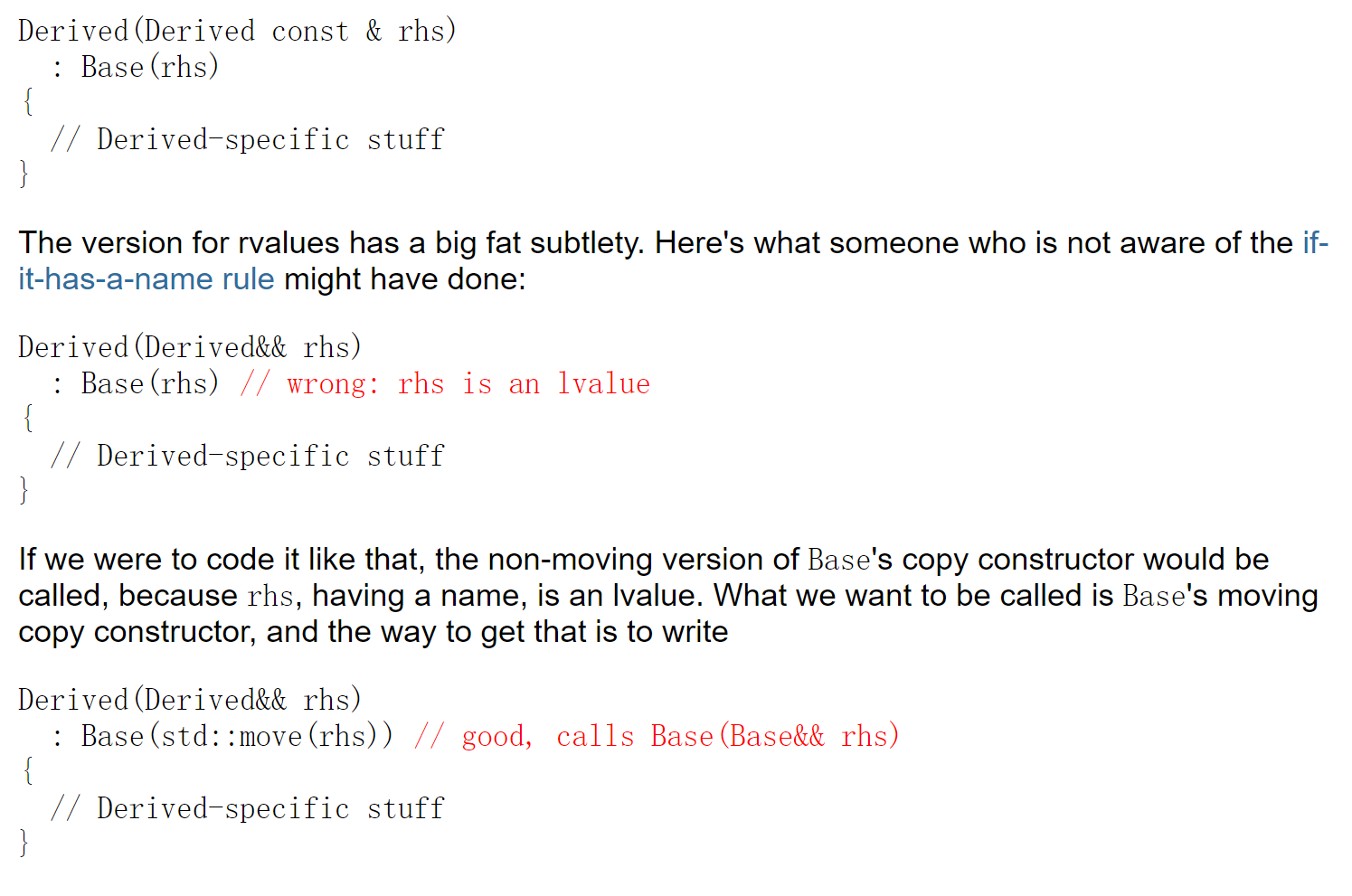#### Move Constructor & Move assignment

StringVector(StringVector&& other);
StringVector& operator=(StringVector&& rhs);


Axess& operator=(Axess&& rhs) {
students = rhs.students;
}


StringVector& StringVector::operator=(const StringVector& rhs) noexcept {
if (this != &rhs) { // IMPORTANT: prevent self-assignment
delete [] _elems;
_logicalSize = rhs._logicalSize;
_allocatedSize = rhs._allocatedSize;
_elems = new std::string[_allocatedSize];
std::copy(rhs.begin(), rhs.end(), begin());
}
return *this;
}

StringVector::StringVector(StringVector&& other) noexcept :
// IMPORTANT: need to move all members, not copy.
// 事实上，对于内置类型使用move没有意义，这里只是用于演示
_logicalSize(std::move(other._logicalSize)),
_allocatedSize(std::move(other._allocatedSize)),
// elems是一个指向string数组的指针
_elems(std::move(other._elems)) {
other._elems = nullptr; // IMPORTANT: set other to valid undetermined state.
}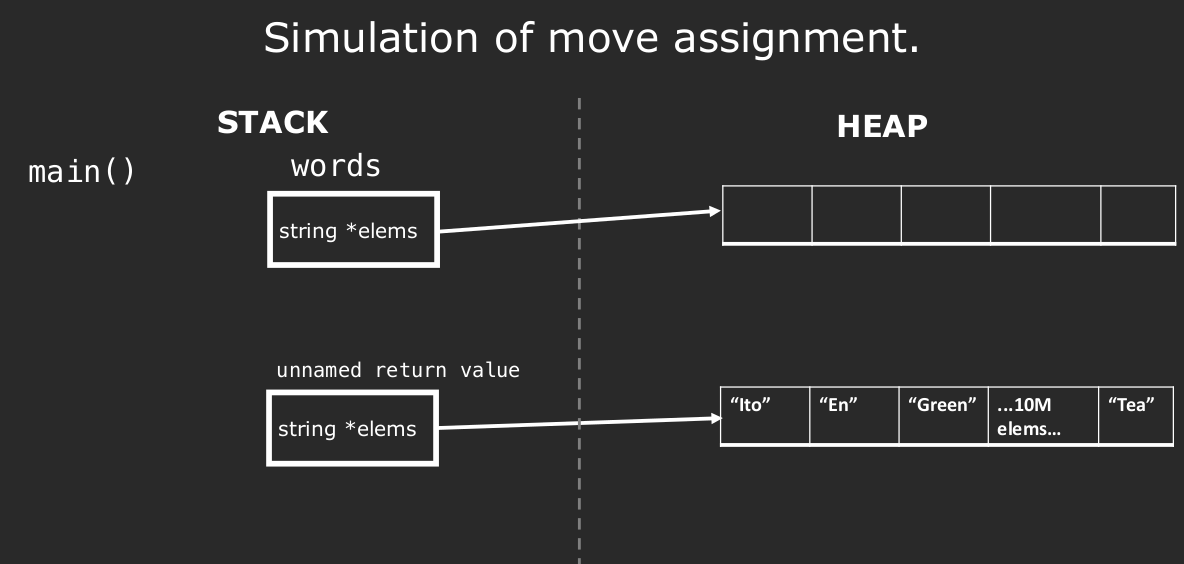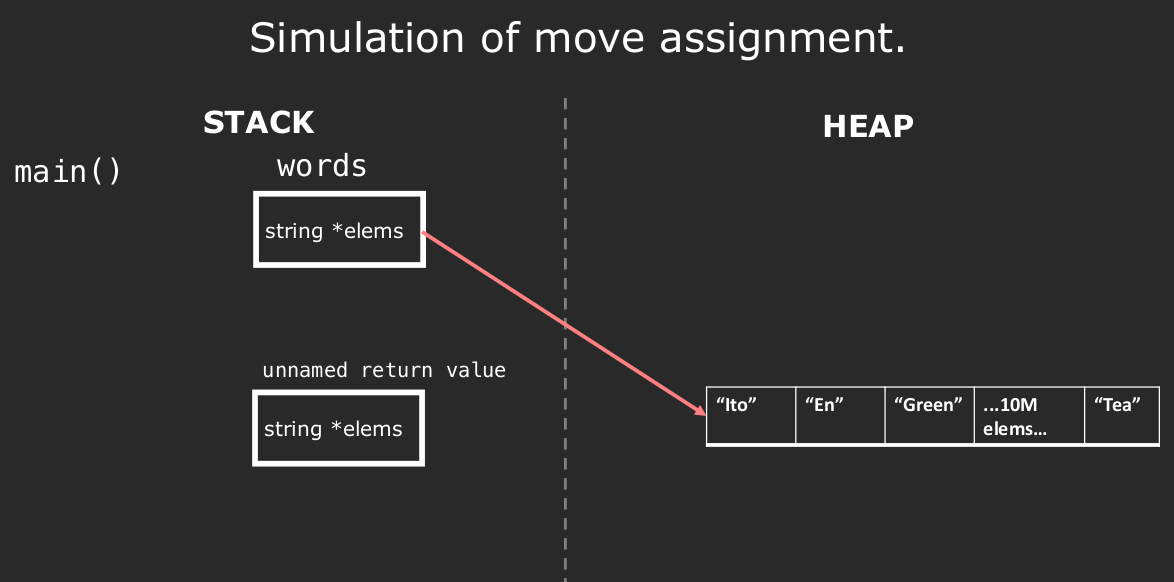1. 移后源对象处于这样一个状态–销毁他是无害的。特别是，一旦资源完成移动，源对象必须不再指向被移动的资源–这些资源的所有权已经归属于新的对象;
2. 移后源对象进入一个有效且可析构的状态，但用户不能对其值做任何假设.

##### vector’s move operation

• Move Constructor

Move constructor. Constructs the container with the contents of other using move semantics. Allocator is obtained by move-construction from the allocator belonging to other. After the move, other is guaranteed to be empty().

• Move assignment

Move assignment operator. Replaces the contents with those of other using move semantics (i.e. the data in other is moved from other into this container). other is in a valid but unspecified state afterwards.

#### move()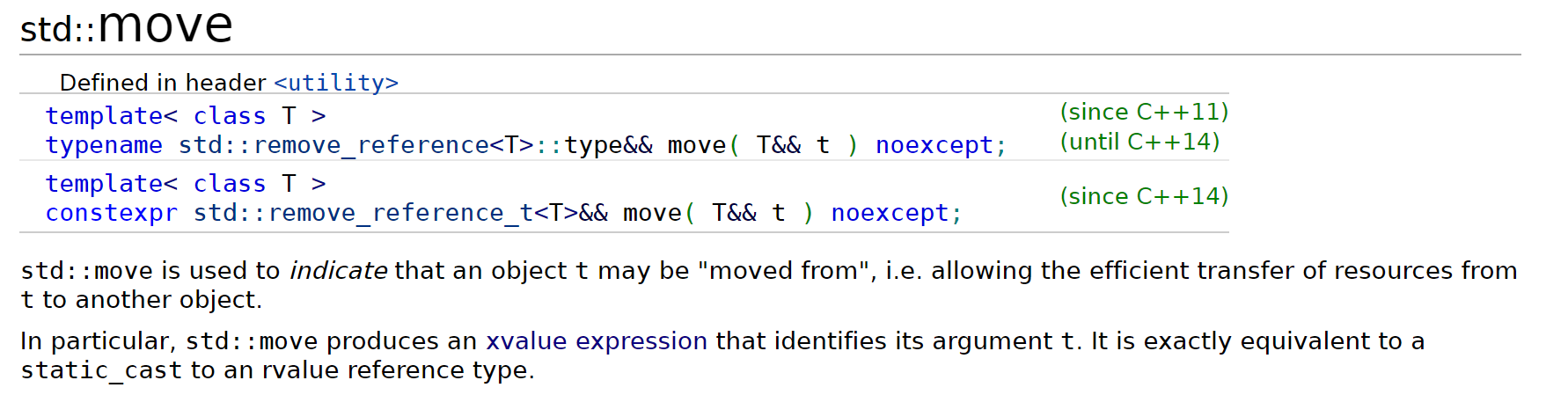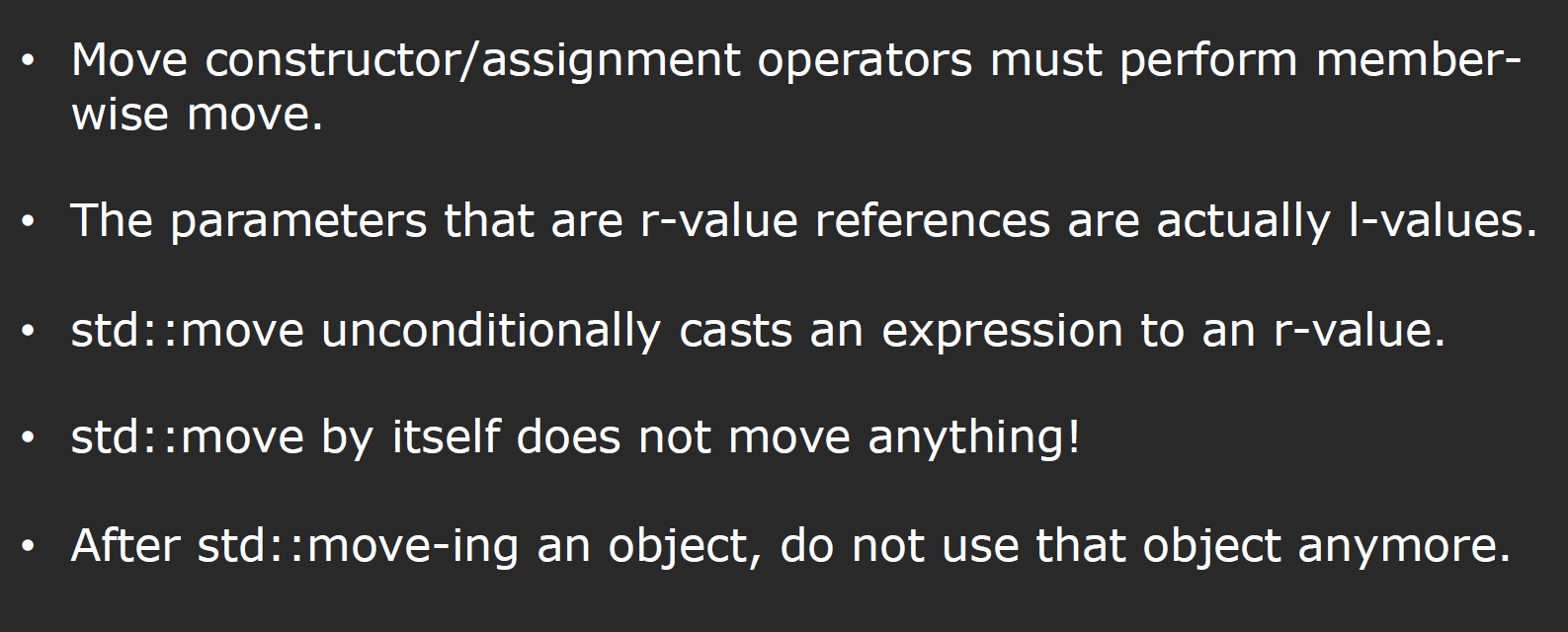template<class T>
typename remove_reference<T>::type&&
std::move(T&& a) noexcept
{
typedef typename remove_reference<T>::type&& RvalRef;
return static_cast<RvalRef>(a);
}


Static_cast: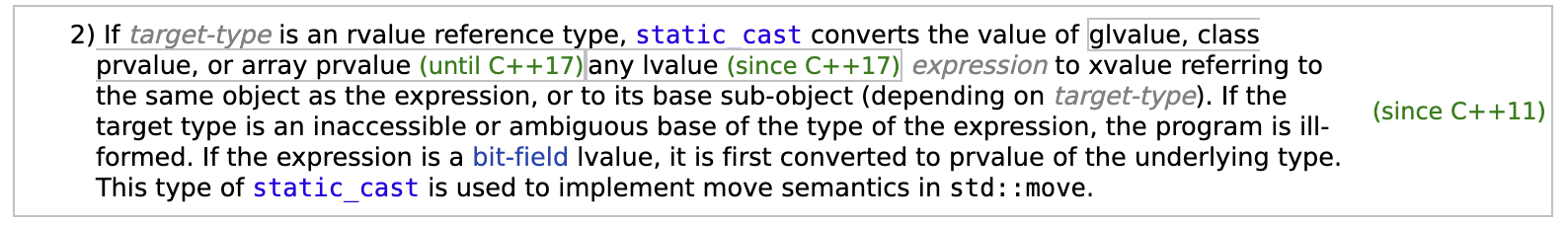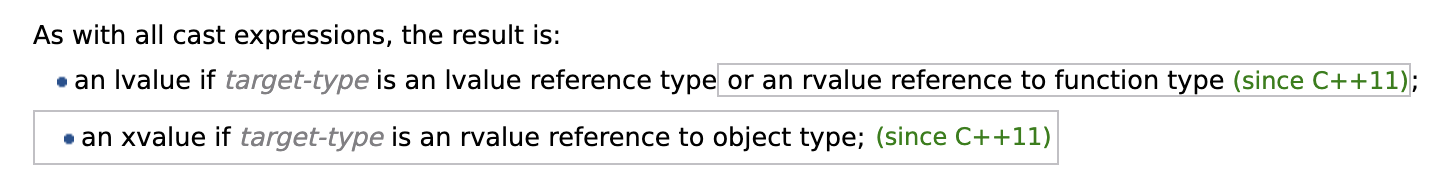std::vector<int> v0{1,2,3};
std::vector<int> v2 = static_cast<std::vector<int>&&>(v0);
std::cout << "2) after move, v0.size() = " << v0.size() << '\n';


remove_reference的实现：利用模板偏特化来实现引用的去除。

template<class T> struct remove_reference { typedef T type; };
template<class T> struct remove_reference<T&> { typedef T type; };
template<class T> struct remove_reference<T&&> { typedef T type; };


#### Universal References

• If the expression initializing a universal reference is an lvalue, the universal reference becomes an lvalue reference.
• If the expression initializing the universal reference is an rvalue, the universal reference becomes an rvalue reference.

Forwarding references are a special kind of references that preserve the value category of a function argument, making it possible to forward it by means of std::forward. Forwarding references are either:

1. function parameter of a function template declared as rvalue reference to cv-unqualified type template parameter of that same function template.
2. auto&& except when deduced from a brace-enclosed initializer list:

cv-unqualified:

A type is “cv-unqualified” if it doesn’t have any cv-qualifiers. A cv-qualifer is either const or volatile

std::vector<int> v;
...
auto&& val = v;


##### Notice! Where type decution takes place?

template<typename T>
void f(const T&& param); // const T rather than T

template<typename T>
void f(std::vector<T>&& param); // vector<T> rather than T


template<typename MyTemplateParamType>
void f(MyTemplateParamType&& param);  // “&&” means universal reference


template <class T, class Allocator = allocator<T> >
class vector {
public:
...
void push_back(T&& x);       // fully specified parameter type ⇒ no type deduction;
...                          // && ≡ rvalue reference
// push_back()无法脱离vector<T>而存在
};


template <class T, class Allocator = allocator<T> >
class vector {
public:
...
template <class... Args>
void emplace_back(Args&&... args); // deduced parameter types ⇒ type deduction;
...                                // && ≡ universal references
};


##### reference to reference

Widget w1;
Widget& & w2 = w1;


##### reference-collapsing

template<typename T>
void f(T&& param);

f(10);
f(x);


• An rvalue reference to an rvalue reference becomes (“collapses into”) an rvalue reference.
• All other references to references (i.e., all combinations involving an lvalue reference) collapse into an lvalue reference.

int x;
int&& r1 = 10;                   // r1’s type is int&&
int& r2 = x;                     // r2’s type is int&

f(r1);
f(r2);


// typedef:
template<typename T>
class Widget {
typedef T& LvalueRefType;
};
// use
Widget<int&> w;


#### Perfect Forwarding

template<typename T, typename Arg>
shared_ptr<T> factory(Arg arg)
{
return shared_ptr<T>(new T(arg));
}

// another example
template <typename A, typename B, typename C>
void f(A& a, B& b, C& c)
{
E(a, b, c);
}


1. std::forward()
2. reference-collapsing
// perfect forwarding
template<typename T, typename Arg>
shared_ptr<T> factory(Arg&& arg)
{
return shared_ptr<T>(new T(std::forward<Arg>(arg)));
}


std::forward()的定义如下：

// forward:
template<class S>
// universal reference only happens in the param list, not at the return value position
S&& forward(typename remove_reference<S>::type& a) noexcept
{
return static_cast<S&&>(a);
}

// called on lvalue
X x;
factory<A>(x);
// called on rvalue
X foo();
factory<A>(foo());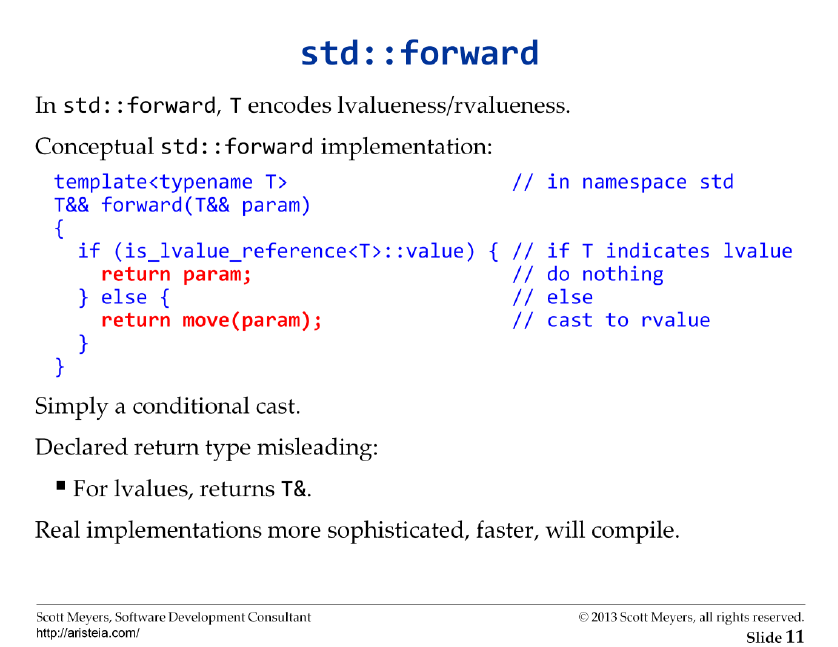• 如果经过引用折叠，此类函数的参数的typervalue reference，参数本身的value category一定是lvalue。在此之后我们使用std::forward，并作为返回值，那么返回值的value category一定与进入“工厂函数”之前相同 —- 依靠std::forward内的static_cast<s&&>value category为左值的传入forward的“工厂函数”参数转化为xvaluevalue category来实现。
• 左值同理。

HashMap作业中，我们实现了一个简易版本的try_emplace，正是利用了完美转发的特性，保证传入函数的参数被完整的保留到数据类型的构造函数中，代码参见仓库：

template<typename K, typename M, typename H>
template<class... Args>
std::pair<typename HashMap<K, M, H>::iterator, bool> HashMap<K, M, H>::try_emplace(K&& key, Args&&... args) {
/** support Automatic Rehash */
rehash(2 * bucket_count() + 1);
}

auto it = find(key);
if (it != end()) {
return {it, false};
}
size_t index = _hash_function(key) % bucket_count();
// Now, we can move key's contents
_buckets_array[index] = std::move(new node({std::move(key), M(std::forward<Args>(args)...)}, _buckets_array[index]));

++_size;

return {{&_buckets_array, _buckets_array[index], index}, true};
}


#### noexcept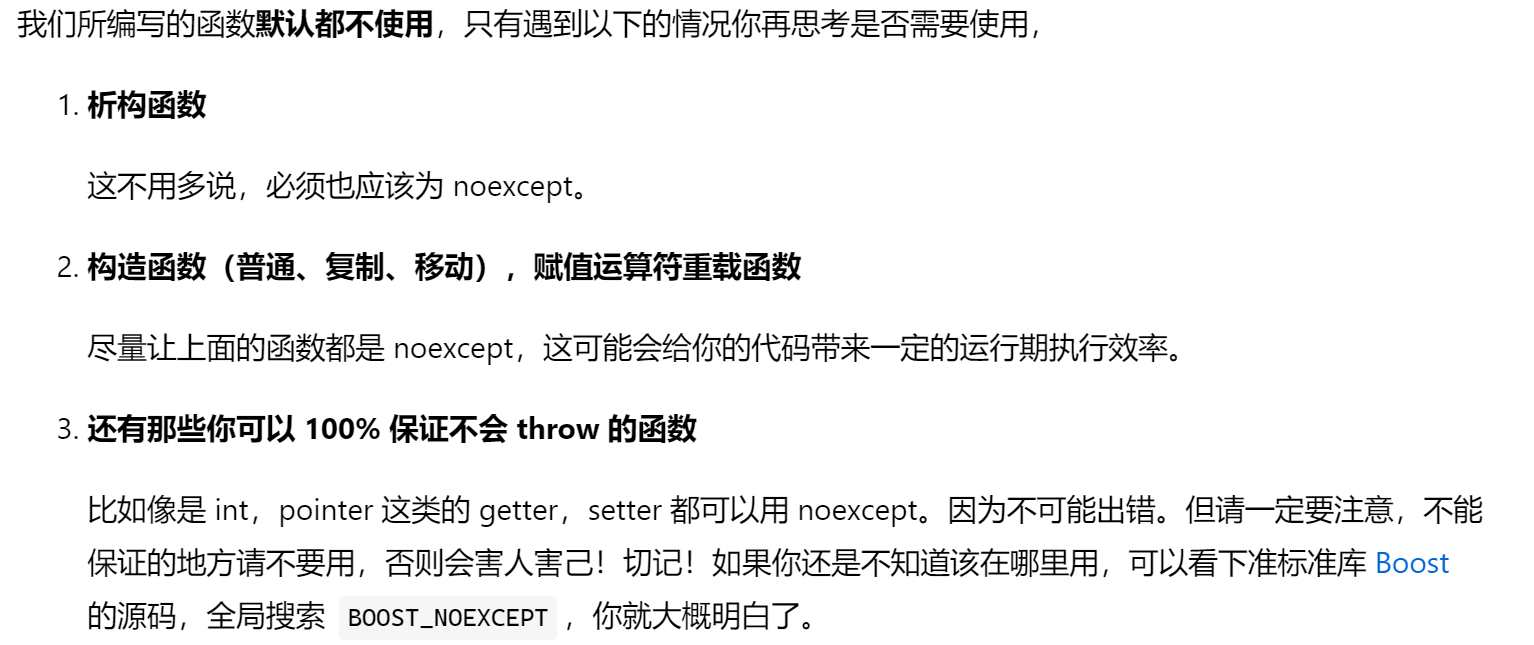#### Rule Of Five

If you explicitly define (or delete) a copy constructor, copy assignment, move constructor, move assignment, or destructor, you should define (or delete) all five.

The fact that you defined one of these means one of your members has ownership issues that need to be resolved.

### Copy elision & RVO

copy elision，也叫复制省略，复制省略常出现我们使用临时对象初始化一个新对象时

• 在 “return” 语句中，当操作数为与函数返回类型为同一类类型
• 在变量的初始化中，当初始化表达式为与变量类型为同一类类型

1. 一个函数以值传递参数，当调用时，我们选择传入一个临时对象作为参数：
void foo(MyClass param) {
// do something
}

int main() {
// pass a temporary object to initialize the param
foo(MyClass());
}


1. 还有一种情况，是函数返回一个临时对象，这里**C++**引出了重要的编译器优化方法：RVO.

struct C {
C() {}
C(const C&) { std::cout << "A copy was made.\n"; }
};

C f() {
return C();
}

int main() {
std::cout << "Hello World!\n";
C obj = f();
}


Hello World!

Hello World!

Hello World!


MyClass foo() {
MyClass obj;
// do something
return obj;
}

// amother case: as a parameter
MyClass bar(MyClass obj) {
// do something
return obj;
}


NRVO目前仍被列为可选优化，也就是说如果开启NRVO，我们仍需保证存在合适的复制构造函数/移动构造函数。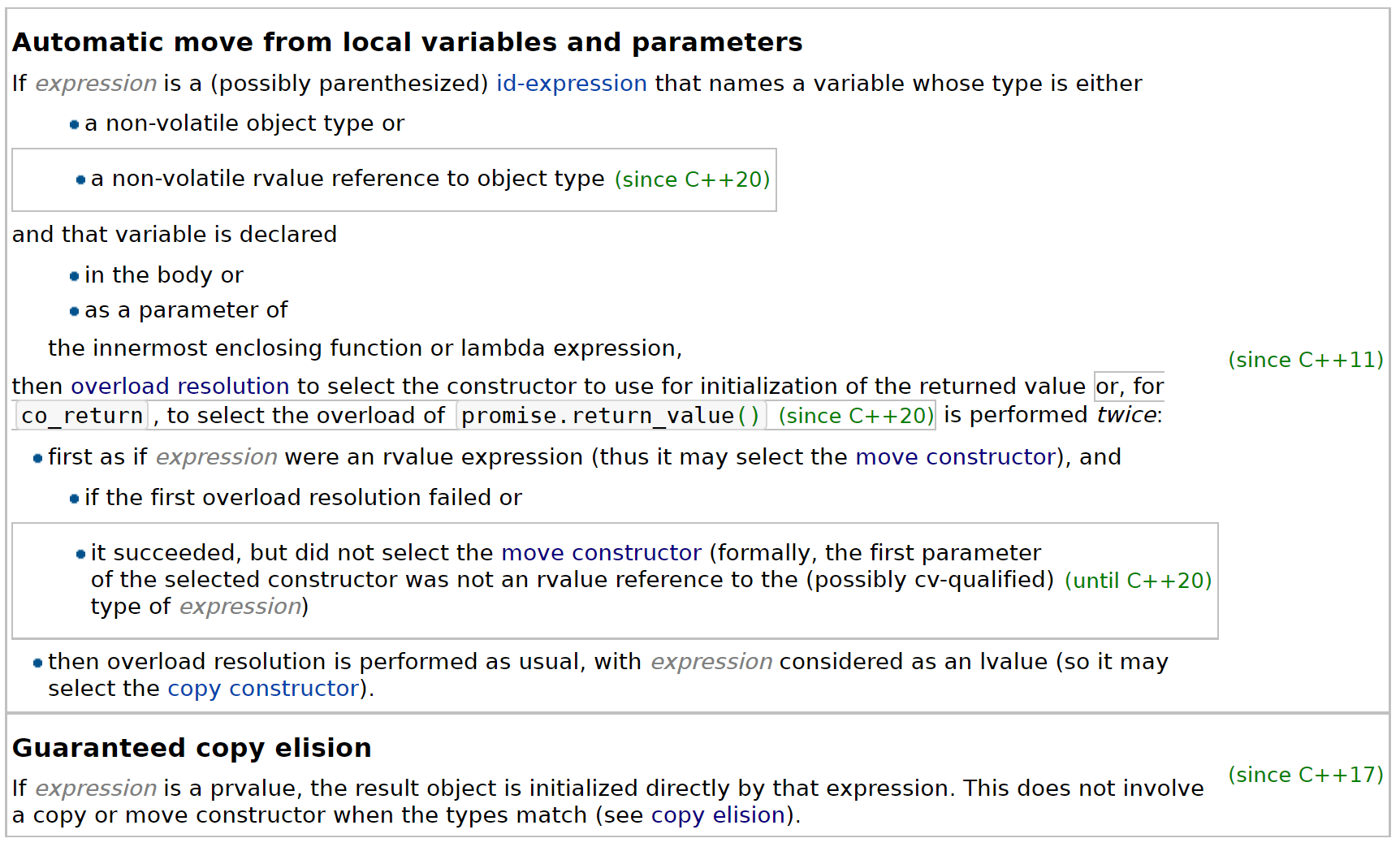### Polymorphism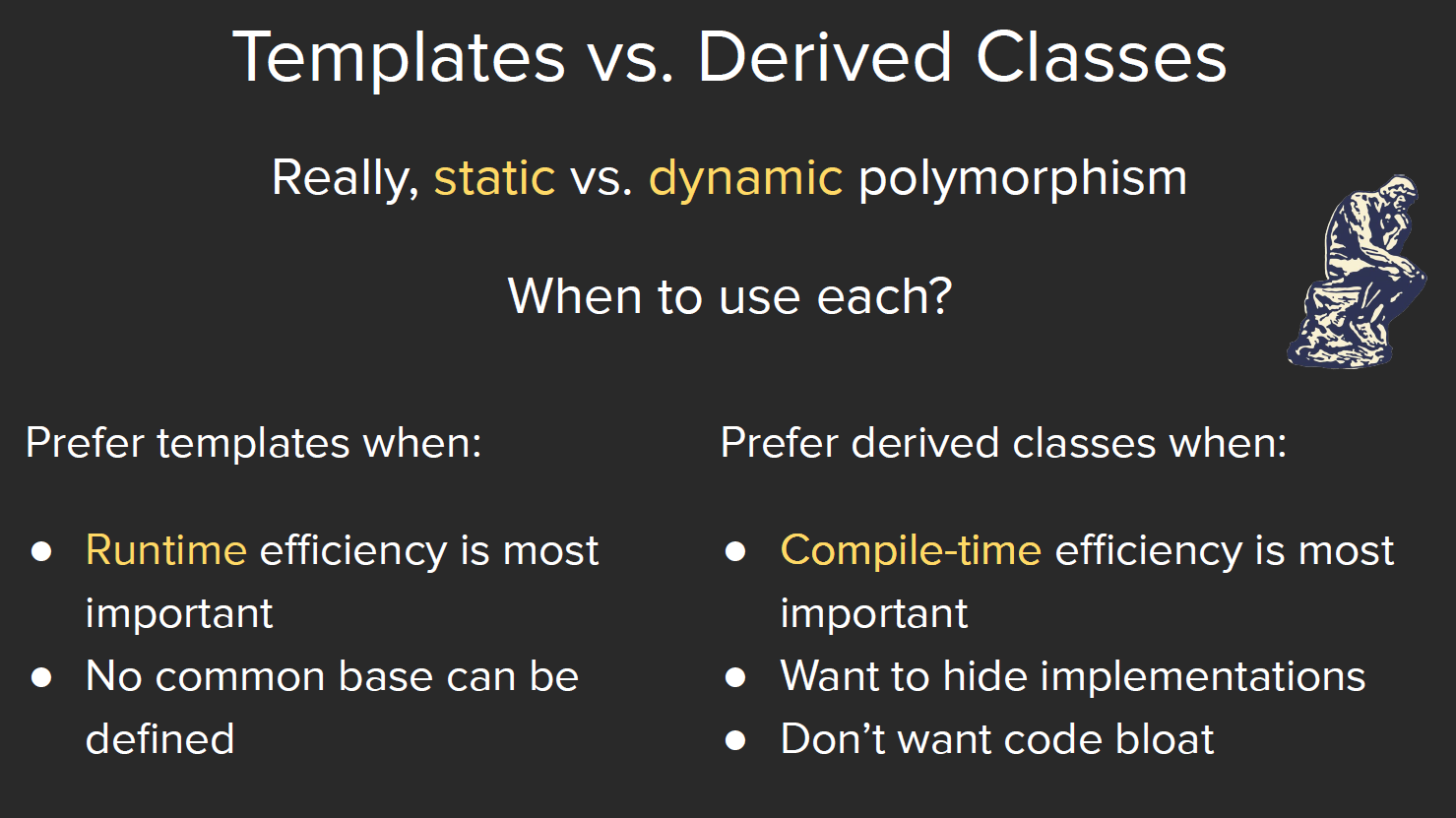CS 106X的课程中，我们详细讨论了关于从派生类到基类的隐式转换过程。在这一节中我们即将提到动态绑定（Dynamic Binding），以及许多其他的继承（Inheritance）的注意事项。

#### Inheritance

class A : public B {

}


##### static

static对象可以分为non-local staticlocal static.non-local static对象的初始化发生在加载阶段，即main函数执行前。但C++没有规定多个non-local static对象的初始化顺序，尤其是来自多个编译单元的non-local static对象，他们的初始化顺序是随机的.

1. 类的静态成员独立于任何对象之外，对象中不包含任何与静态成员有关的数据
2. 类内静态成员和静态成员函数均不与对象绑定在一起，故静态成员函数不包含this指针，不能声明成const的，我们也不能在static函数体内使用this指针。
3. 成员函数不需要作用域运算符即可访问静态成员。
4. 一般来讲，我们只能在类内声明静态成员；类外初始化、定义静态成员，但存在一些例外：
• static const int成员可以在类内声明+初始化，如果static const不是整型，则不可以类内初始化;
• static constexpr成员必须在类内初始化；若编译时static constexpr数据成员可用它的替代（如表示数组个数等），它可以不需要类外定义。若不能替代（如作为参数等），类外必须含有一条定义语句(所以习惯上无论如何都在类外定义一下)
1. 当在类的外部定义静态函数时，不能重复static关键字，该关键字只出现在类内的声明语句;

2. 我们可以使用静态数据成员作为成员函数的默认实参，但非静态成员不行，因为他们的值是对象的一部分，如果非静态成员如果在成员函数被调用前没有被初始化，将导致在函数调用时编译器无法得知默认实参的值是多少。所以编译器做了这条规定，将可能出现的错误及时避免在编译期。

虚函数也可以拥有默认实参，但是虚函数的默认实参由本次调用的静态类型决定。所以，虚函数的默认实参在基类和派生类中最好一致。

##### final & override

class A final {/* */}


void foo() const final;


void foo() const override;


##### Accessible？

• 普通用户
• 类的实现者
• 派生类

class Base {
protected:
int prot_mem;
};

class sneaky : public Base {
friend void clobber(sneaky&);	// can access sneaky::prot_mem
friend void clobber(Base&);     // can NOT access Base::prot_mem
};


class Base {
public:
void pub_mem();
protected:
int prot_mem;
private:
char priv_mem;
};
struct Pub_Derv : public Base {
int f() { return prot_mem; }  // OK, can access protected members
char g() { return priv_mem; } // error, can NOT access Base's private members
};
struct Priv_Derv : private Base {
int f1() const { return prot_mem; } // ok
}

Pub_Derv d1;
Priv_Derv d2;
d1.pub_mem();		// ok
d2.pub_mem();		// error


##### virtual dtor

Quote *itemP = new Quote;	// static type = dynamic type
delete itemP;				// call Quote's dtor
itemP = new Bulk_quote;		// static type != dynamic type
delete itemP;				// call Bulk_quote's dtor


##### Dynamic Binding

double print_total(const Quote &item) {
double ret = item.net_price(n);
}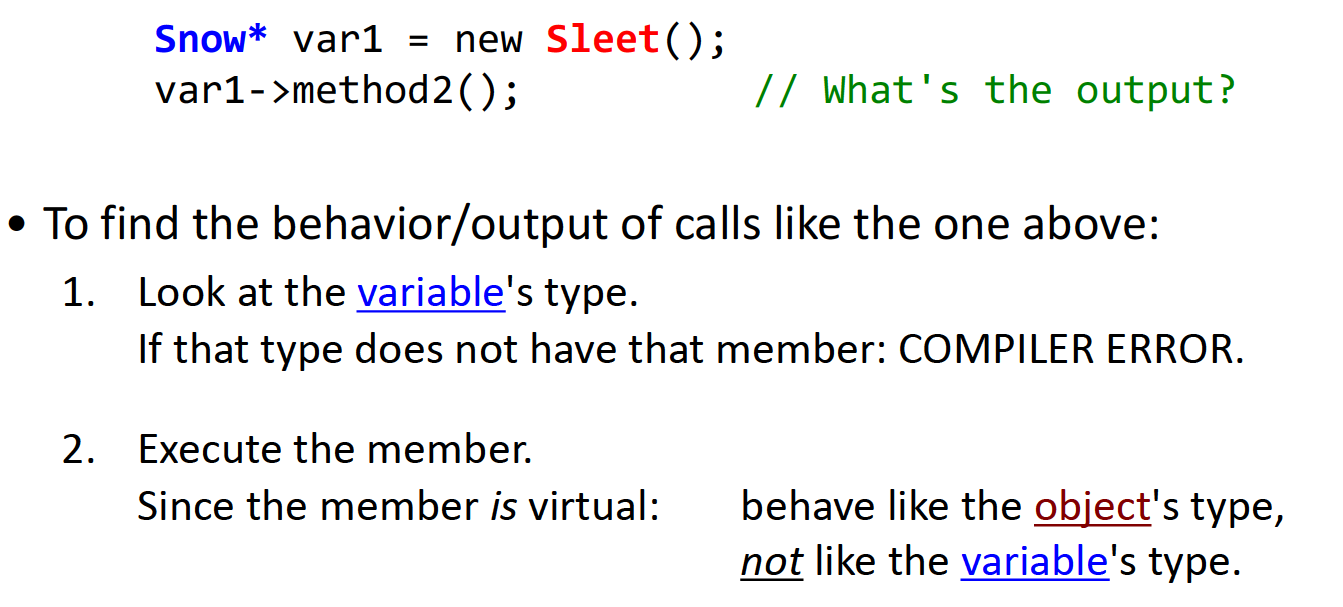Quote base;
Bulk_quote* bulkP = &base;    //error, base->derive class
Bulk_quote& bulkRef = base;   //error, base->derive class


• dynamic_cast:RTTI(run-time type identification)

if (Derived *dp = dynamic_cast<Derived*>(bp)) {
// use dp pointing to Derived
}
else {
// use bp pointing to Base
}

void f(const Base &b) {
try {
const Derived &d = dynamic_cast<const Derived&>(b);
}
// deal with failure
}
}

• static_cast:已知转换安全，强制覆盖掉编译器的检查工作

##### vptr & vtbl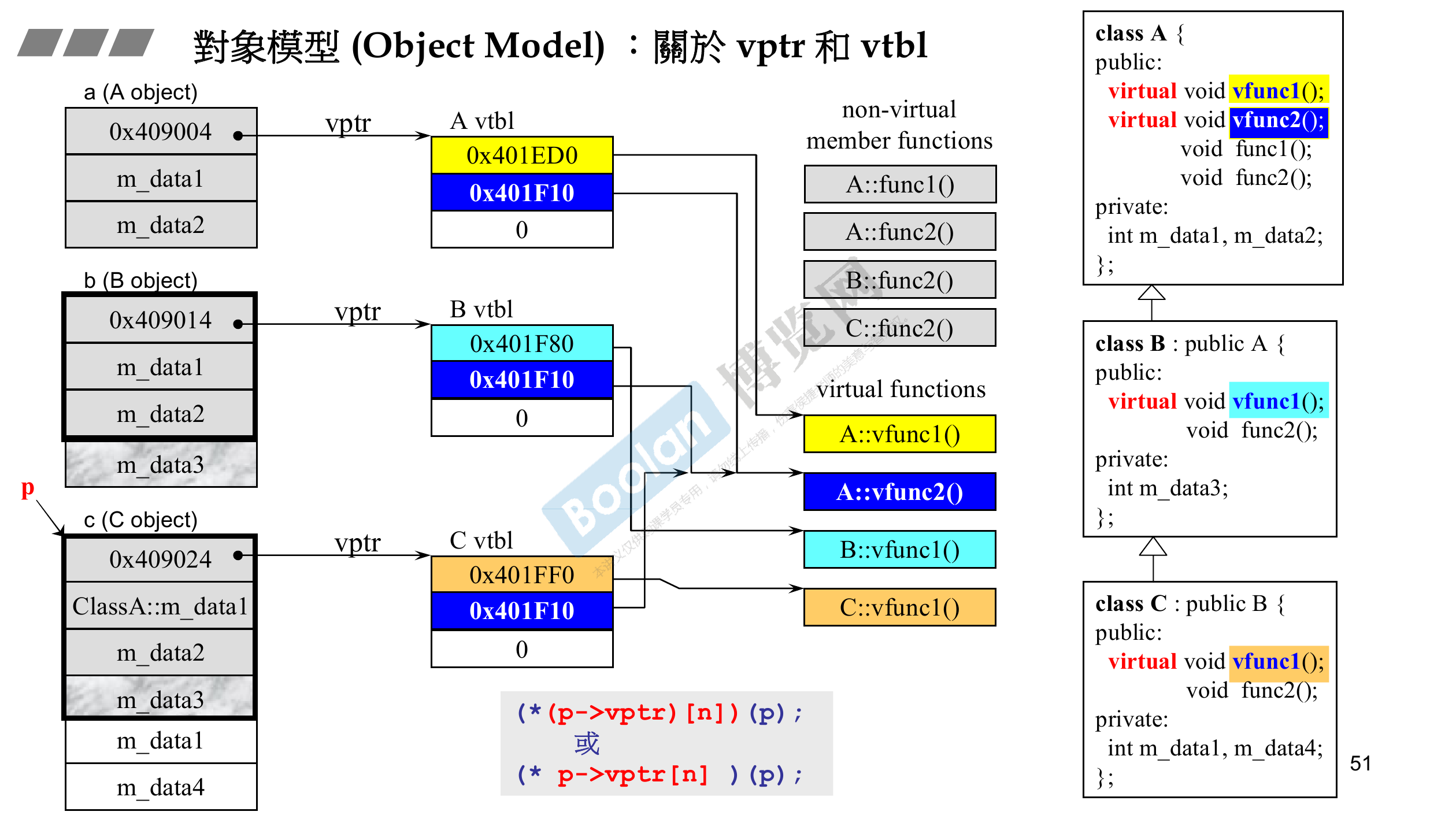##### Inheritance on Templates

- 依赖性基类

- 非依赖性基类

template <typename X>
class Base
{
public:
int basefield;
typedef  int  T;
};

template <typename T>
class D2 : public Base<double> // INdependent on template T
{T strange; };


C++规定，派生类查找一个非受限名称时，会先在非依赖型基类(Base<double>)中查找，然后才查找模板参数列表(template<typename T>)。所以上例中的strangeBase<double>::T而非模板定义中的T.

template <typename T>
class D2 : public Base<T> // dependent on template T
{T strange; };


// This is a declaration
template<typename X>
class Base
{
public:
int basefield;
typedef int T;
};

// This is a definition
template <typename T>
class DD : public Base<T>
{
public:
void f() { basefield = 0; }     // (1)problem……
};

template<>    // 显式特化
class Base<bool>
{
public:
double basefield = 1.0;
};

void g(DD<bool>& d)
{
d.f();
}


// 修改方案1
template <typename T>
class DD1 : public Base<T>
{
public:
void f() { this->basefield = 0; }     // 查找被延迟了
};

// 修改方案2：利用受限名称来引入依赖性
template <typename T>
class DD2 : public Base<T>
{
public:
void f() { Base<T>::basefield = 0; }
};


// 修改方案3:重复的限定让代码不雅观，可以在派生类中只引入依赖型基类
template <typename T>
class DD3 : public Base<T>
{
public:
using Base<T>::basefield;       // (1)依赖型名称现在位于作用域
void f() { basefield = 0; }     // 正确
};


## RAII & Smart Pointers

### RAII

RAIIC++的一种重要机制。什么是RAII？中文翻译为资源获取即初始化–使用局部对象来管理资源的技术。他还有几个名字，比如SBRMScope Based Resource Management），CADREConstructor Acquires, Destructor Releases）。这样做有什么好处？根据下图所示的例子：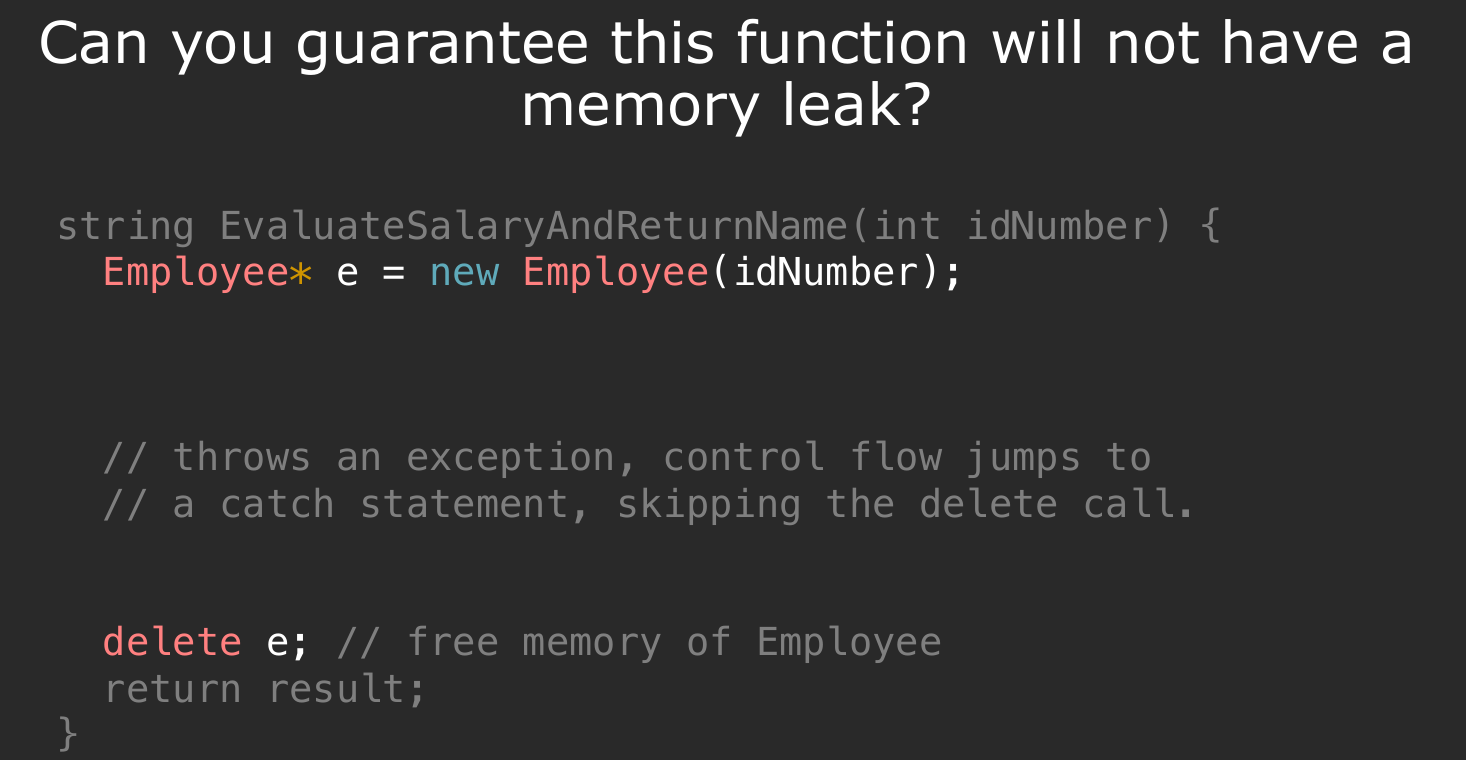There should never be a ”half-valid” state of the object. Object immediately useable after its creation.

The destructor is always called (even with exceptions), so the resource is always freed.

RAII机制在多处都有体现，包括我们经常使用的newdelete过程，以及：

• 文件的打开与关闭-C++内部为我们提供了具备RAII机制的文件处理操作：
// DO NOT WRITE IN THIS WAY:
{
ifstream input();
input.open("test.txt");
// do something
input.close();
}

// with RAII provided
{
ifstream input("test.txt");
// do something
// no need to close the file anymore!
}

// NO NO NO
void cleanDatabase (mutex& databaseLock, map<int, int>& database) {
databaseLock.lock();
// other threads will not modify database
// modify the database
// if exception thrown, mutex never unlocked!
databaseLock.unlock();
}

void cleanDatabase (mutex& databaseLock, map<int, int>& database) {
lock_guard<mutex>(databaseLock);
}
class lock_guard {
public:
lock_guard(mutex& lock) : acquired_lock(lock) {
acquired_lock.lock()
}
~lock_guard() {
acquired_lock.unlock();
}
private:
mutex& acquired_lock;
}


### new & delete

Complex* pc = new Complex(1, 2);
String* str = new String("Hello");


#### new

• 分配内存->使用operator new的内置函数
String* str;
void* mem = operator new(sizeof(String));


• 转型->使用static_cast
str = static_cast<String*>(mem);

• 使用构造函数
str->String::String("Hello");


1. 分配内存，分配的是谁的内存？需要注意的是，这一步分配的并不是我们传入的Hello字符串的内存，而是类，也就是对象本身的内存。
2. 使用构造函数时，我们也会分配内存，在这里，我们分配的是所传入的对象，即C式字符串的内存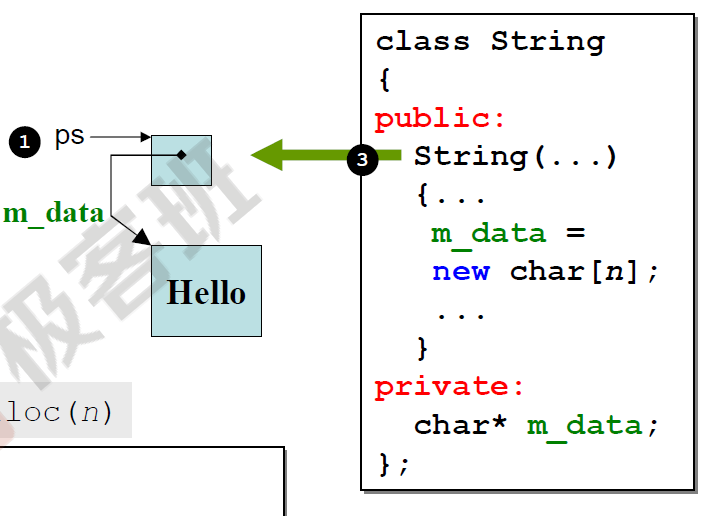ps是指向类对象的指针，而每个类对象中有一个私有成员指针用来表示传入的字符串（一个char数组）的头指针，指向另一块内存空间。

Complex* pc;
pc->Complex::Complex(1, 2);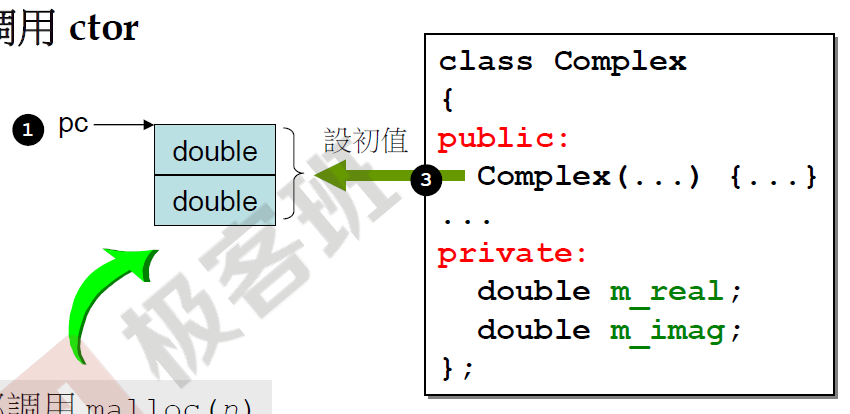auto p1 = new auto(obj); // OK
auto p2 = new auto{a, b ,c}; // error


const int *pc1 = new const int(1024);


#### delete

Calling a destructor releases the resources owned by the object, but it does not release the memory allocated to the object itself.

• 调用析构函数
String::~String(str);


~String() {
delete [] m_data;
}

• 释放对象内存->使用内置的operator delete函数
operator delete(str);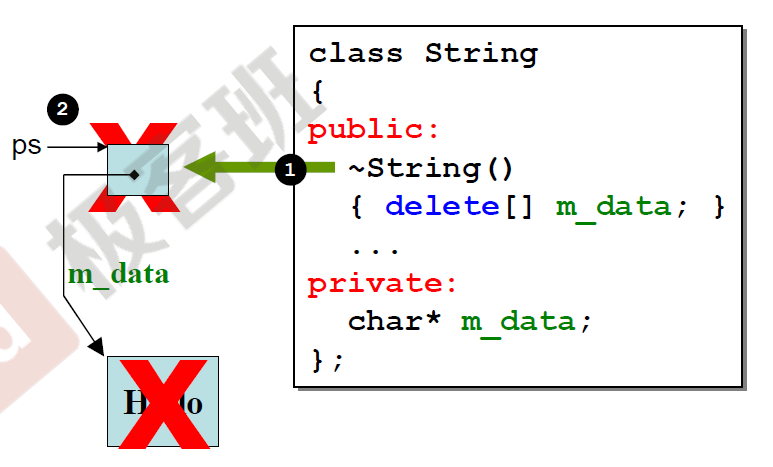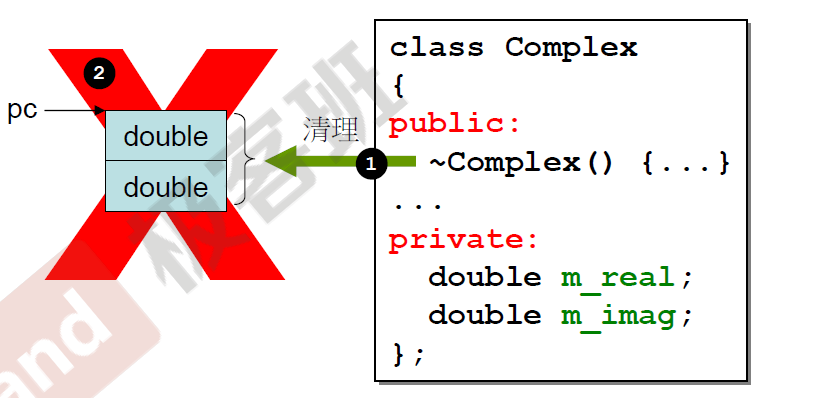#### array new & array delete?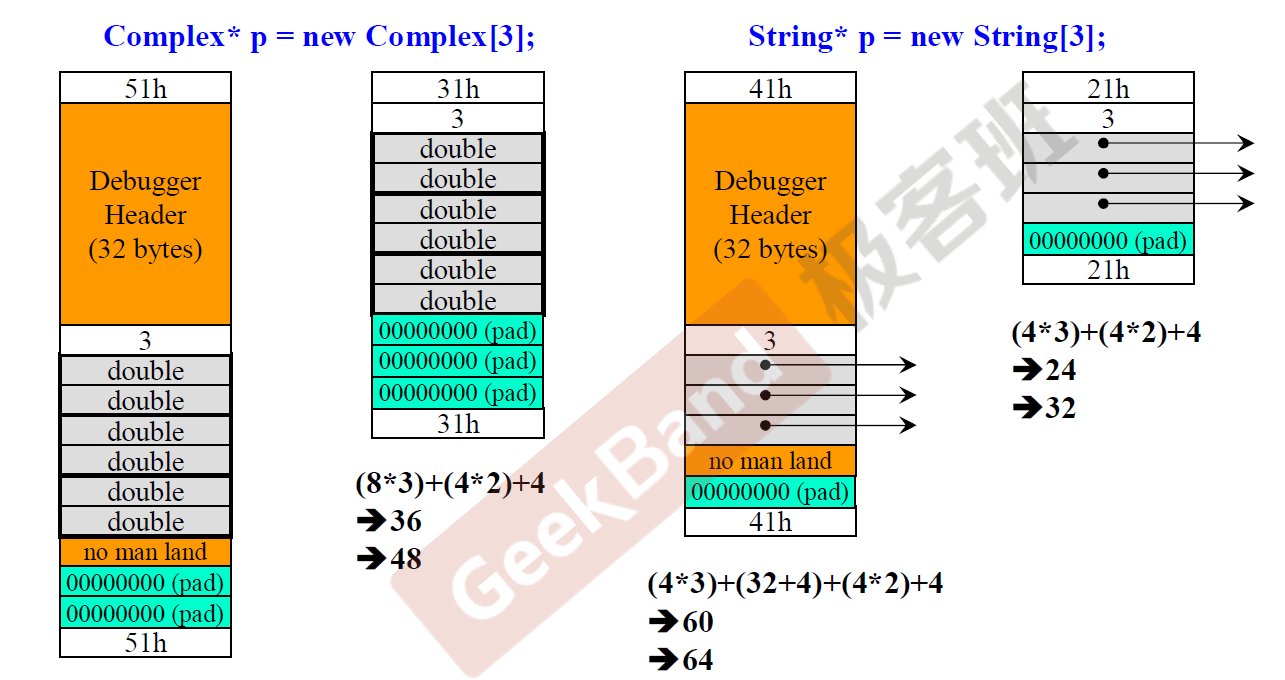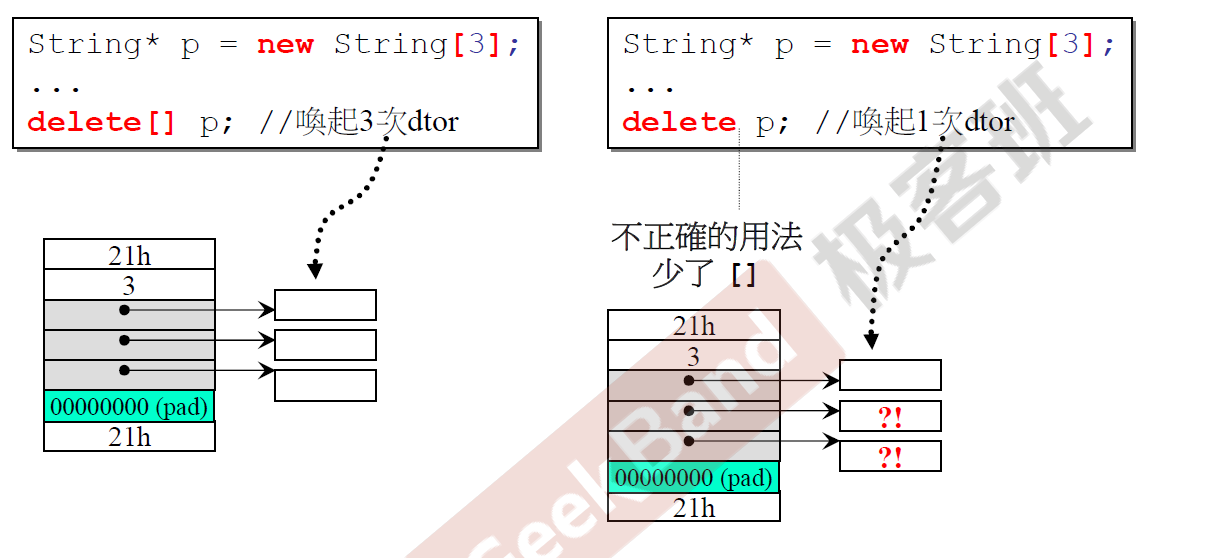newdelete是一个表达式，行为无法重载，但其中调用的operator new(operator new[])operator delete(operator delete[])是一个函数，可以被重载。

// look for members, if no member, use global one
Foo* pf = new Foo;
// force to use the global one
Foo* pf = ::new Foo;
// delete 同理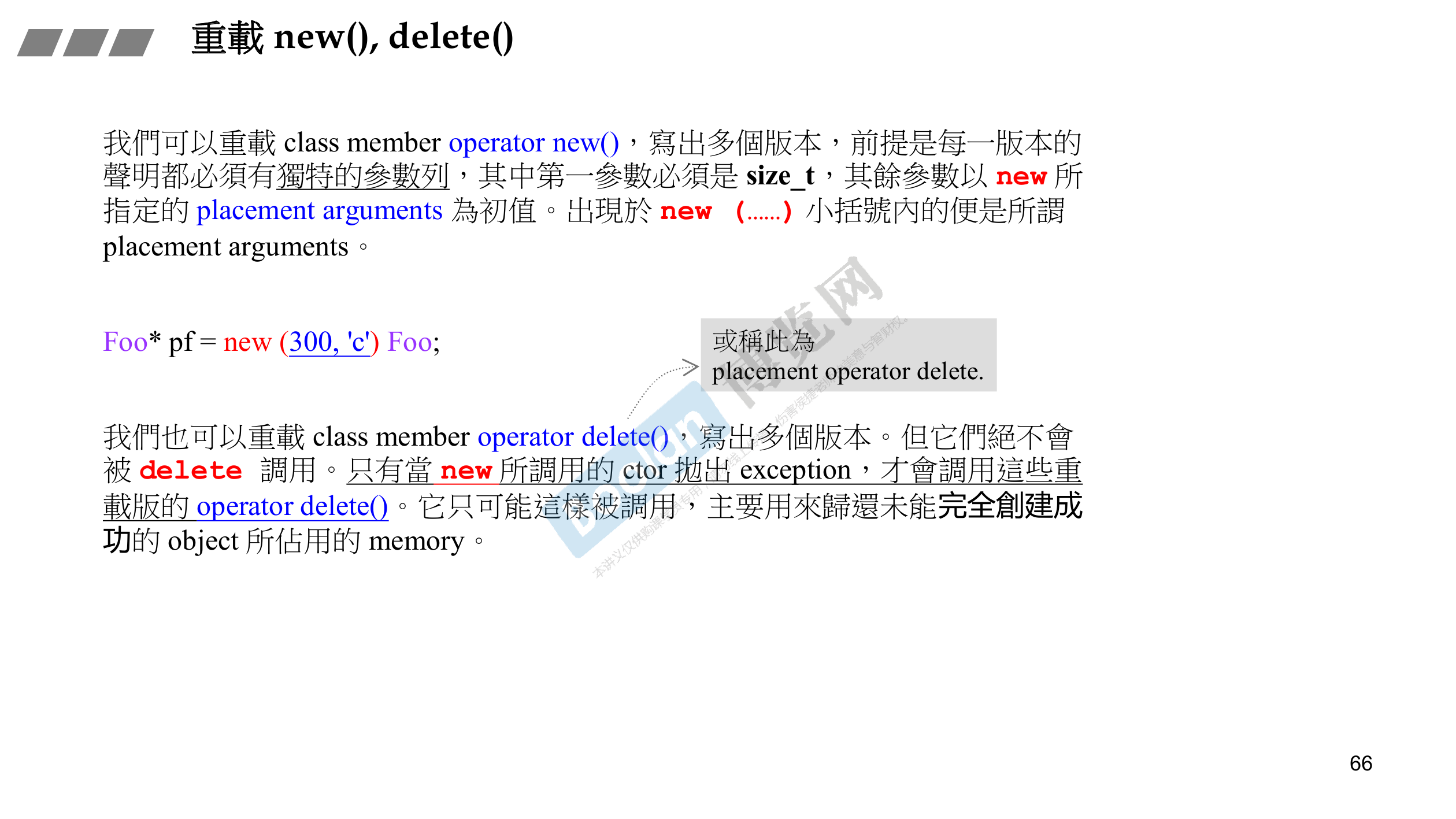### Smart Pointers

• shared_ptr
• unique_ptr
• weak_ptr

#### shared_ptr

if (p1 && p1->empty()) {
// do something
}


// we can use auto here
auto p = make_shared<string>(10, '9');


##### reference_counting

shared_ptr的一大特征是允许多个指针指向同一个对象，为了操作这一特性，每一个shared_ptr都有一个关联的计数器，即引用计数reference counting）功能。当我们进行拷贝或者赋值操作时，计数器都会作相应的变化：

• 创建一个shared_ptr对象，计数器为1；
• 将对象q赋值给pp=q），则相应地，p的计数器-1q的计数器+1
• 对于两个shared_ptr对象，当我们使用q初始化p时（p(q)），q的计数器+1，并传递给p
• 当指向某对象的任意一个shared_ptr计数器为0时，销毁对象

##### NOTICE!
• 接受指针参数的智能指针构造函数是explicit的，这意味着我们不能将一个内置指针形式隐式转化为智能指针，必须使用直接初始化
shared_ptr<int> p1 = new int(3); // error
shared_ptr<int> p2(new int(3)); // OK

shared_ptr<int> clone(int p) {
return new int(2); // error
}

• 不要混合使用智能指针和普通指针

// a function
void process(shared_ptr<int> p) {
// do something
} // then the temp p will be destroied (go out of scope)


shared_ptr<int> ptr(new int(3));
process(ptr);


int *ptr = new int(3);
process(ptr);


int *x = new int(3);
process(shared_ptr<int> ptr(x));
int j = *x; // oop, x is a dangling pointer

• 不要使用get初始化另一个智能指针或者为智能指针赋值

shared_ptr<int> p(new int(42));
int *q = p.get();
// undefined:
shared_ptr<int>(q);


• reset

reset会更新计数器，如果需要会释放对象：

p = new int(1024);
p.reset(new int(3));


#### unique_ptr

shared_ptr所不同的是，unique_ptr只允许一个该类型指针指向某个对象。正因为此，其不支持普通的拷贝或者赋值操作，我们只能将其直接初始化:

unique_ptr<int> pInt(new int(5));	// 显然这里用的是move语义
cout << *pInt;
unique_ptr<int> pInt2(pInt);    // error
unique_ptr<int> pInt3 = pInt;   // error

// OR
auto ptr = std::make_unique<int>(...);


unique_ptr<string> p2(p1.release());


unique_ptr<int> pInt3(std::move(pInt2));


release返回当前的unique_ptr保存的指针并将其置空，并将p1的控制权转移给p2.

p3.reset(p2.release());


exception:

unique_ptr<int[]> up(new int(10));
up.release(); // use delete[]


## Function Pointers

### Function

#### Function pointer declaration

bool foo(const string&, cosnt string&);


bool (*pf)(const string&, const string&);


bool *pf(const string&, const string&);


#### Use function pointers

pf = foo;
pf = &foo; // the same

pf("1", "2");
(*pf)("1", "2"); // the same

##### As parameters
void useBigger(bool pf(const string&, const string& ));
void useBigger(bool (*pf)(const string&, const string& )); // the same

##### As return values

• 函数返回类型
• 函数参数类型
• 函数参数个数

using F = int(int*, int);
F f(int);


int (*) (int*, int);


int (*foo(int, int)) (int*, int);


auto f1(int)->int(*)(int*, int);


### Array

#### Decalaration

int *p1; //p1 is an array, which has 10 pointers that pointing to int.
int (*p1); // p1 is a pointer, pointing to an array which has 10 ints.


int (*func(int i));


auto func(int i) -> int(*);


int(*pf)(int*, int);


### typedef & decltype

#### typedef

##### variable

typedef double wages; // wages is a type alias of double
typedef wages base, *p; // ???


typedef char *pstring; // the same as above

##### array & struct

typedef int arrs;


##### function & function pointers
typedef bool func(const string&, const string&);


typedef bool (*func)(const string&, const string&)


#### decltype

decltype的简单用法这里不提，这里首先说一条关键的语法规则：

delcltype((variable))（双层括号）的结果永远是引用，而decltype(variable)的结果只有当variable的结果本身是引用时才是引用。（是引用意味着我们必须对其初始化.）

int foo(int i) {
// do something
}
decltype(foo(3));


int foo(int i) {
// do something
}
decltype(foo);


int(int);


typedef decltype(foo) *ptr;


int odd[] = {1,2,3,4,5};
int even[] = {6,7,8,9,10};
decltype(odd) *arrPtr(int i) {
return (i % 2) ? &odd : &even;
}


int(*);


cannot convert ‘int*’ to ‘int (*)’ in return


*odd; // 1
*(&odd); // the same as above

&odd + 1; // we will move to the next variable position (go beyond sizeof(odd) length)
odd + 1; // we will get into the next posision in the current odd array (move sizeof(odd) length)


Array names in a C program are (in most cases) converted to pointers. One exception is when we use the sizeof operator on an array. If a was converted to a pointer in this context, sizeof a would give the size of a pointer and not of the actual array, which would be rather useless, so in that case a means the array itself.